0

# 机器学习（Machine Learning）

## 简介

bili 吴恩达 机器学习

（1）机器学习是一门人工智能的科学，该领域的主要研究对象是人工智能，特别是如何在经验学习中改善具体算法的性能。
（2）机器学习是对能通过经验自动改进的计算机算法的研究。
（3）机器学习是用数据或以往的经验，以此优化计算机程序的性能标准。

• 监督学习
• 无监督学习

## 监督学习 Supervised Learning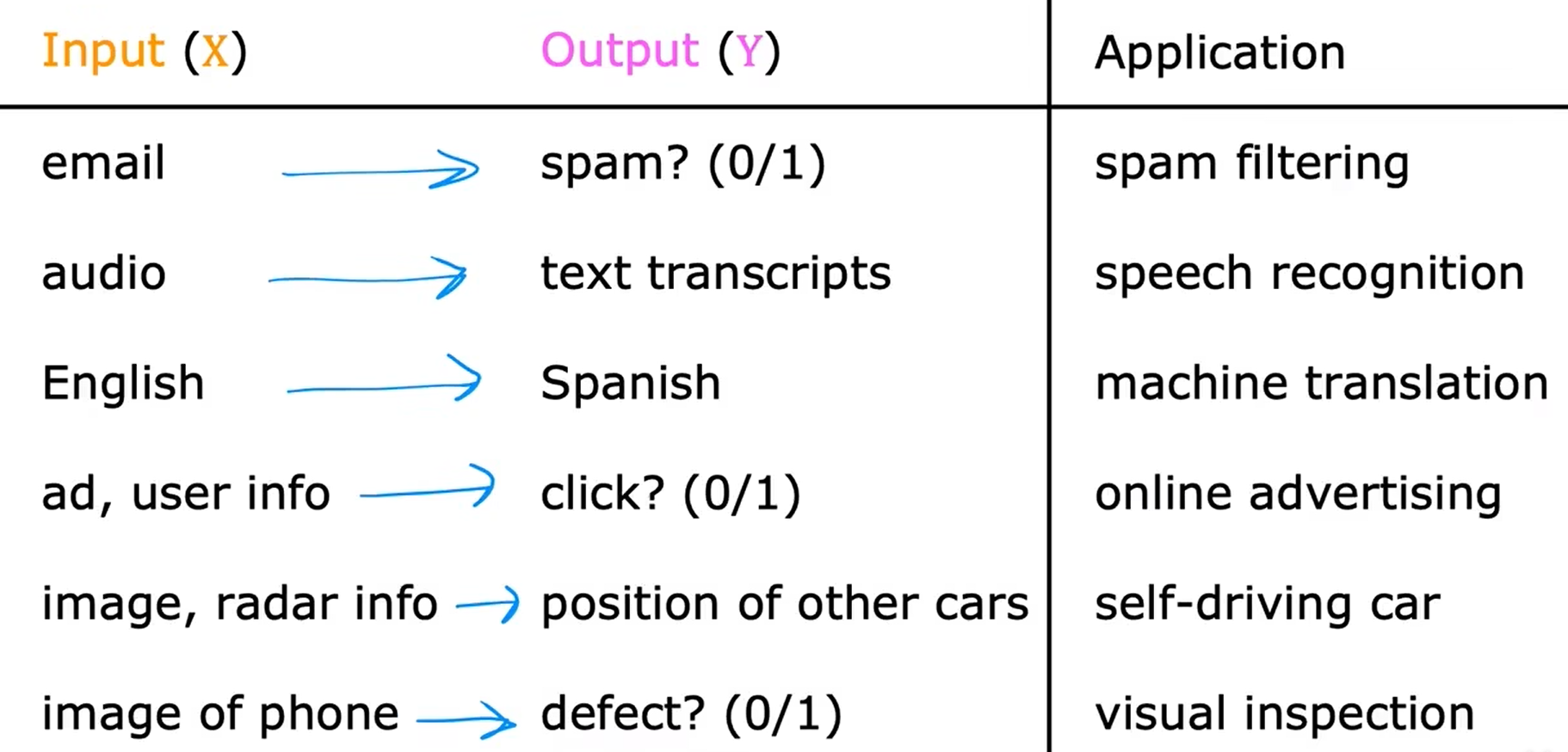• 回归 - 回归试图预测无限多个可能的数字中的任何一个。
• 分类 - 分类是指只预测少量可能的输出或类别。### 回归 Regression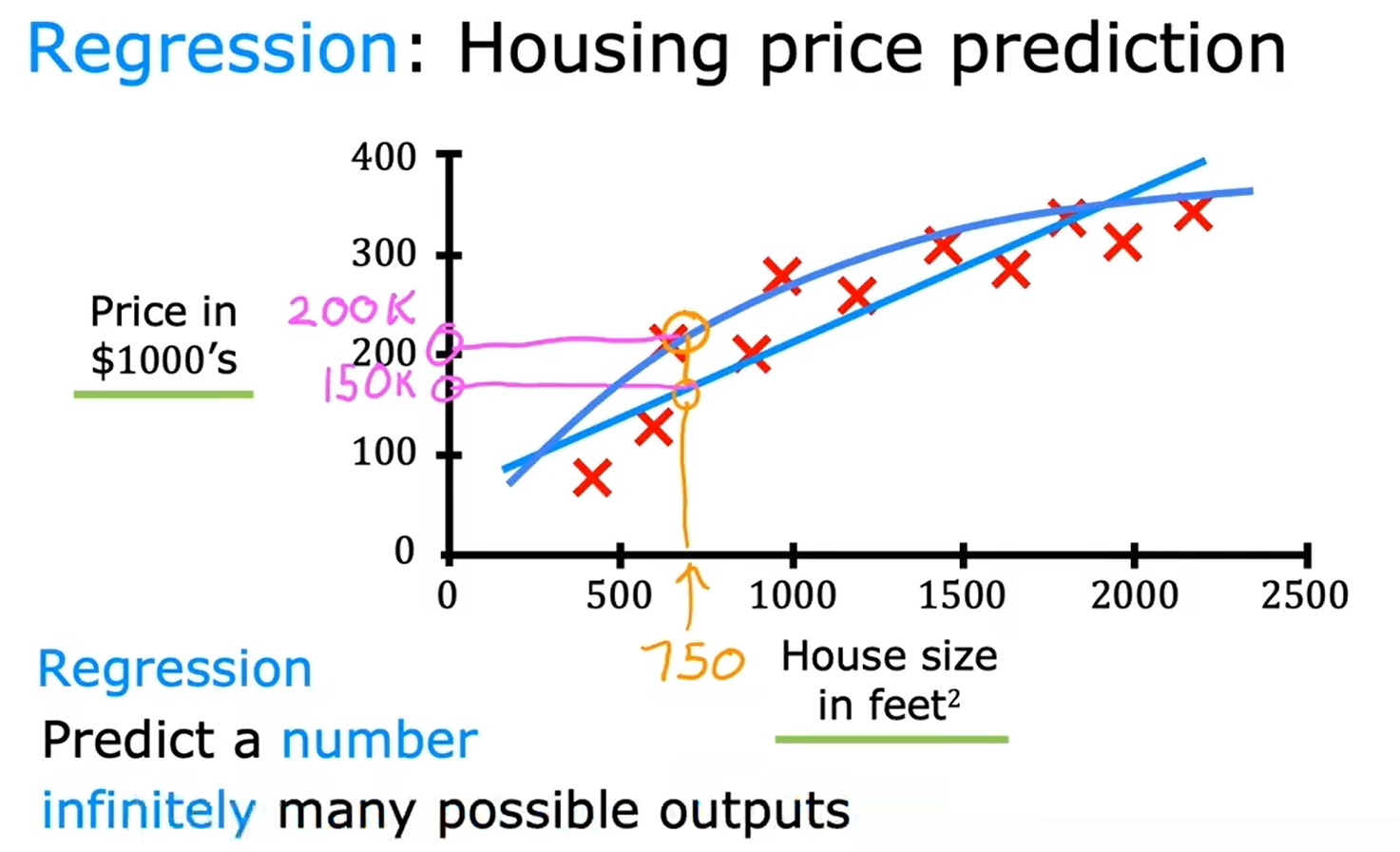### 分类 Classification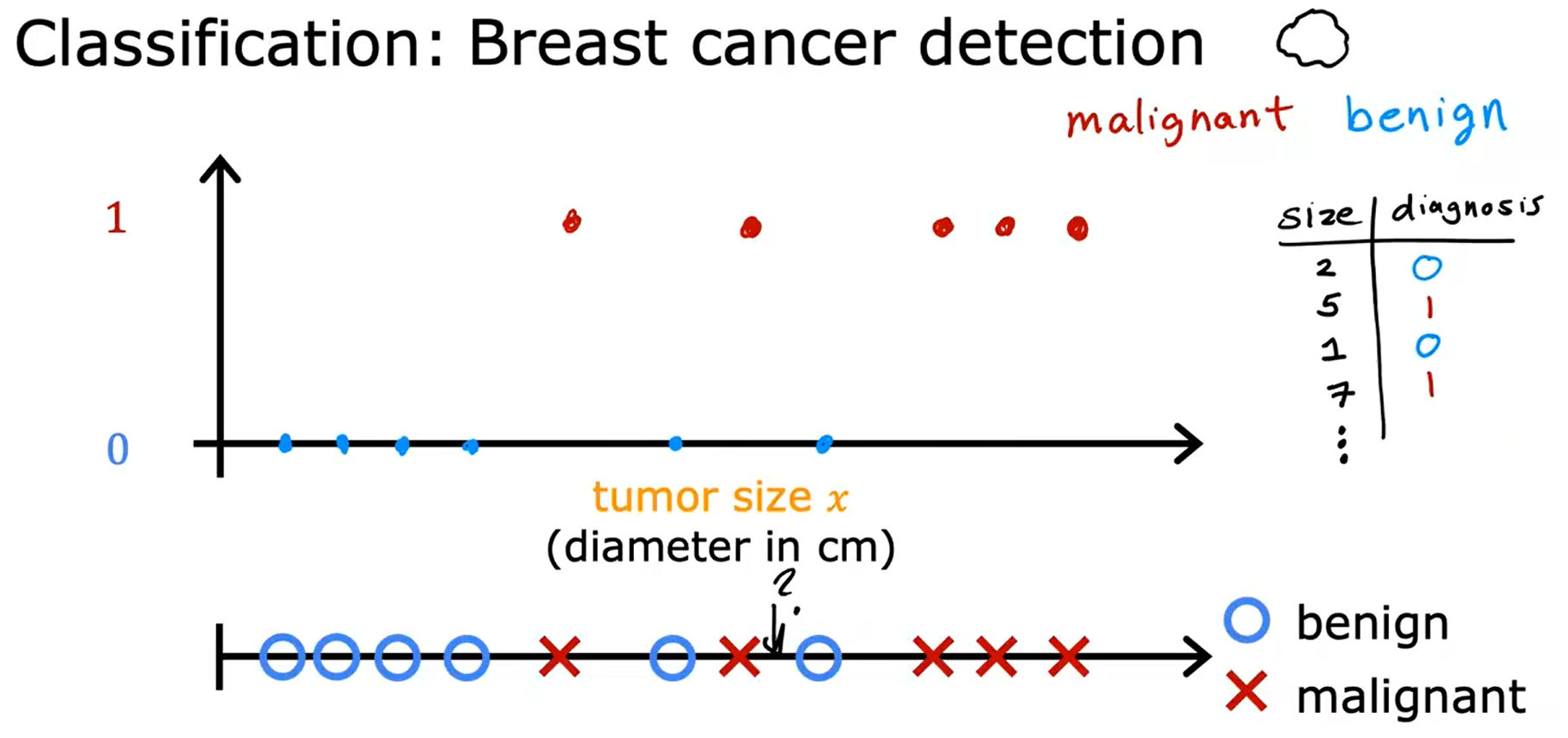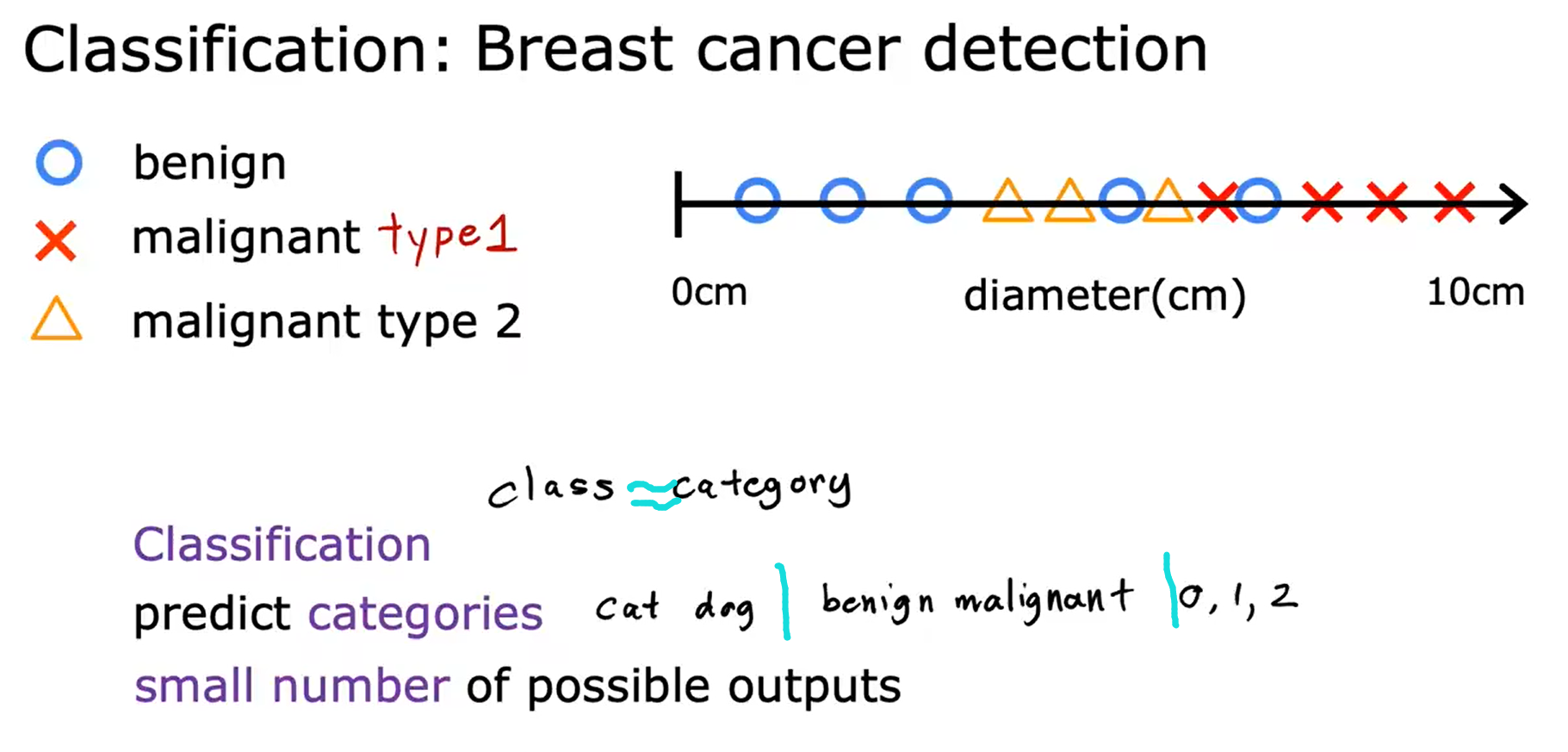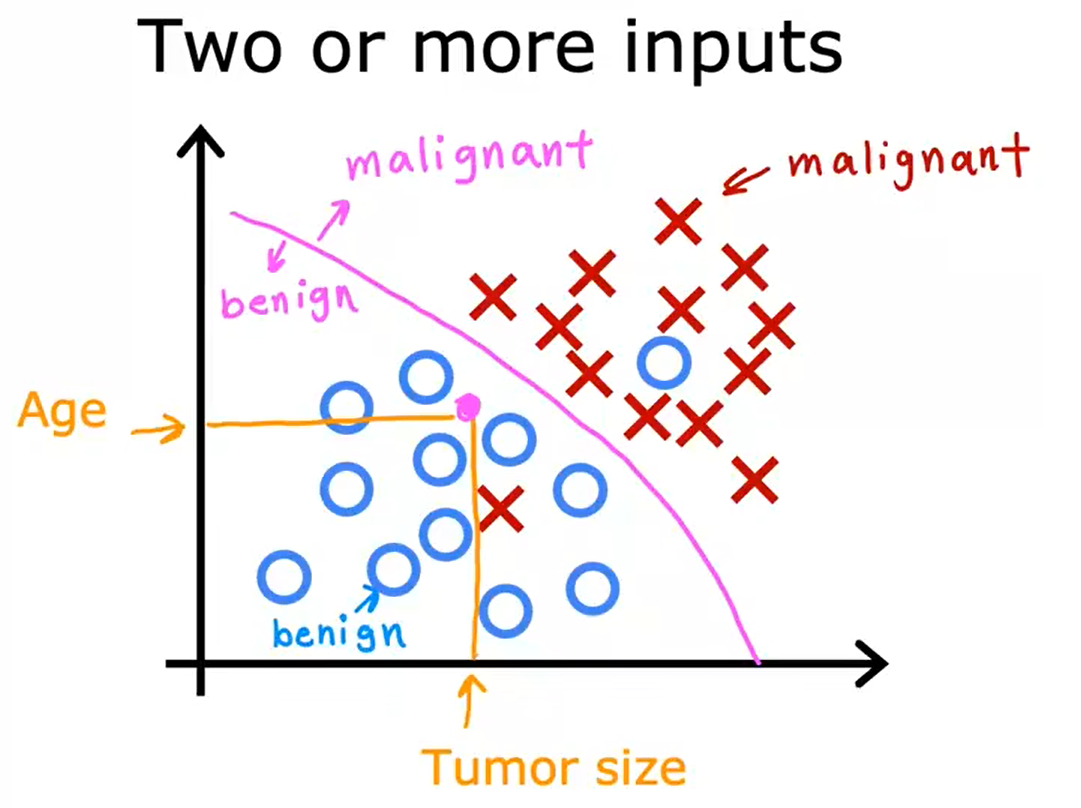## 无监督学习 Unsupervised Learning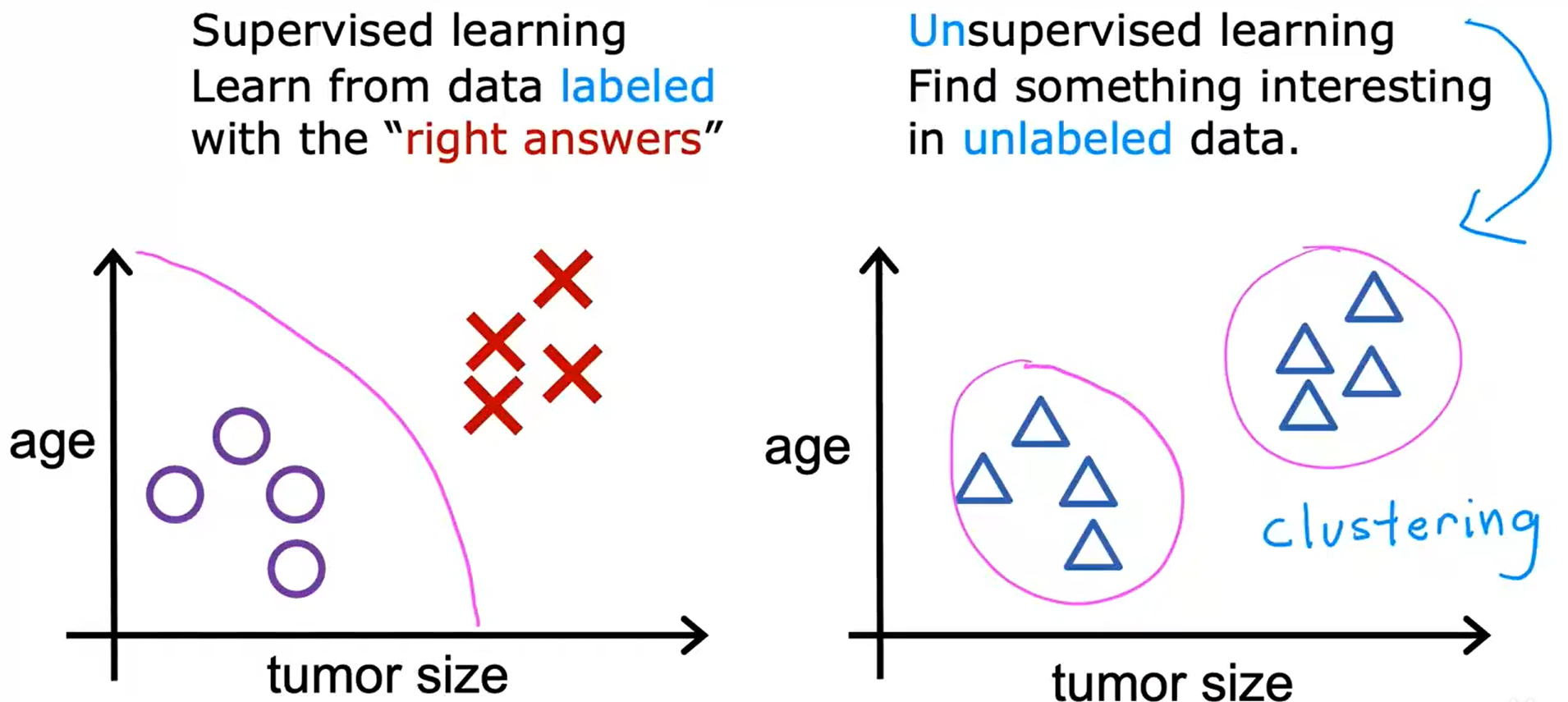• 聚类 - 将相似的数据点分组在一起。
• 异常检测 - 发现异常数据点（金融系统中的欺诈检测）
• 降维 - 使用更少的数字压缩数据，尽可能少的丢失信息

### 聚类 Clustering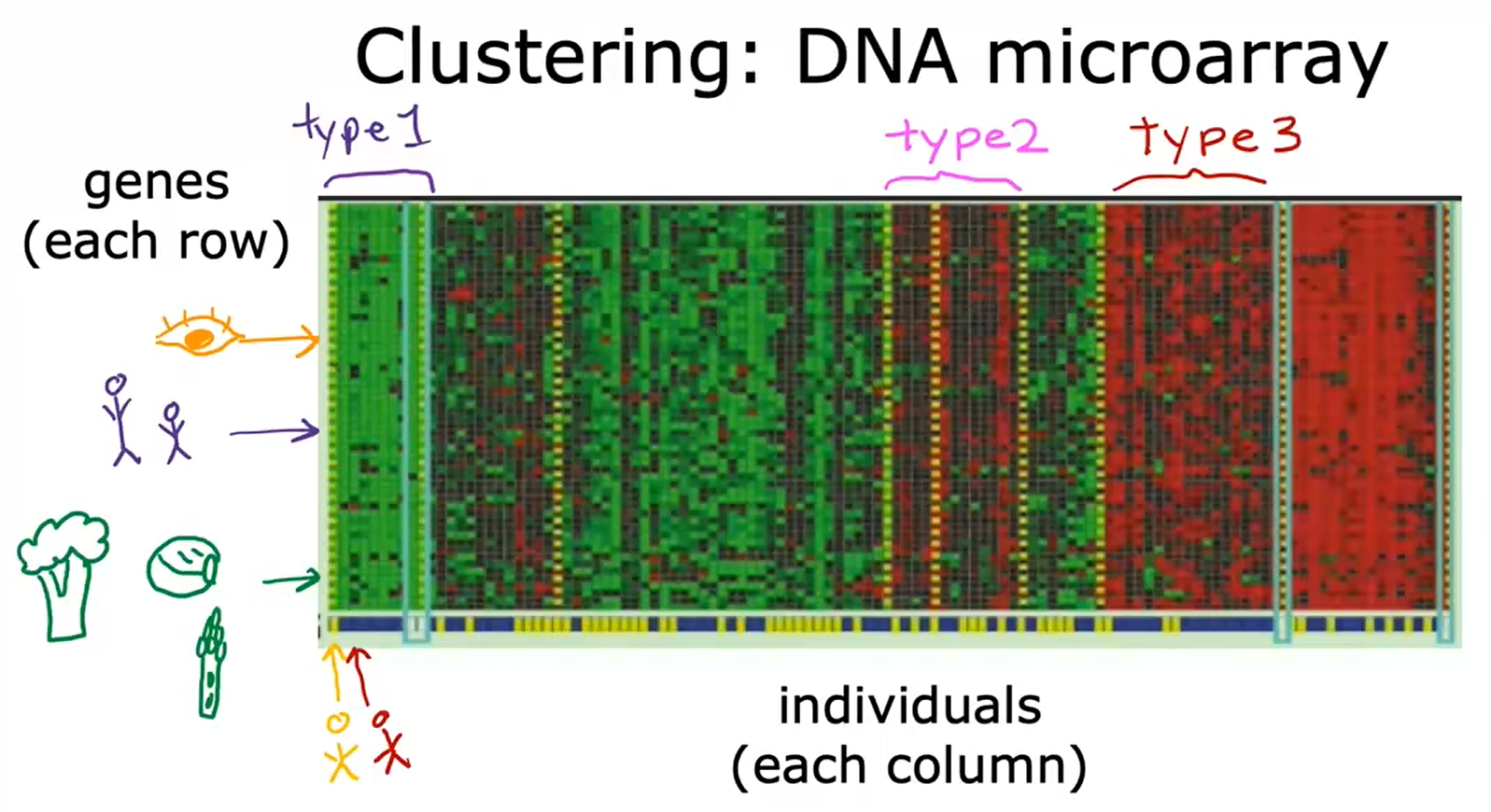## 线性回归模型 Linear Regression Model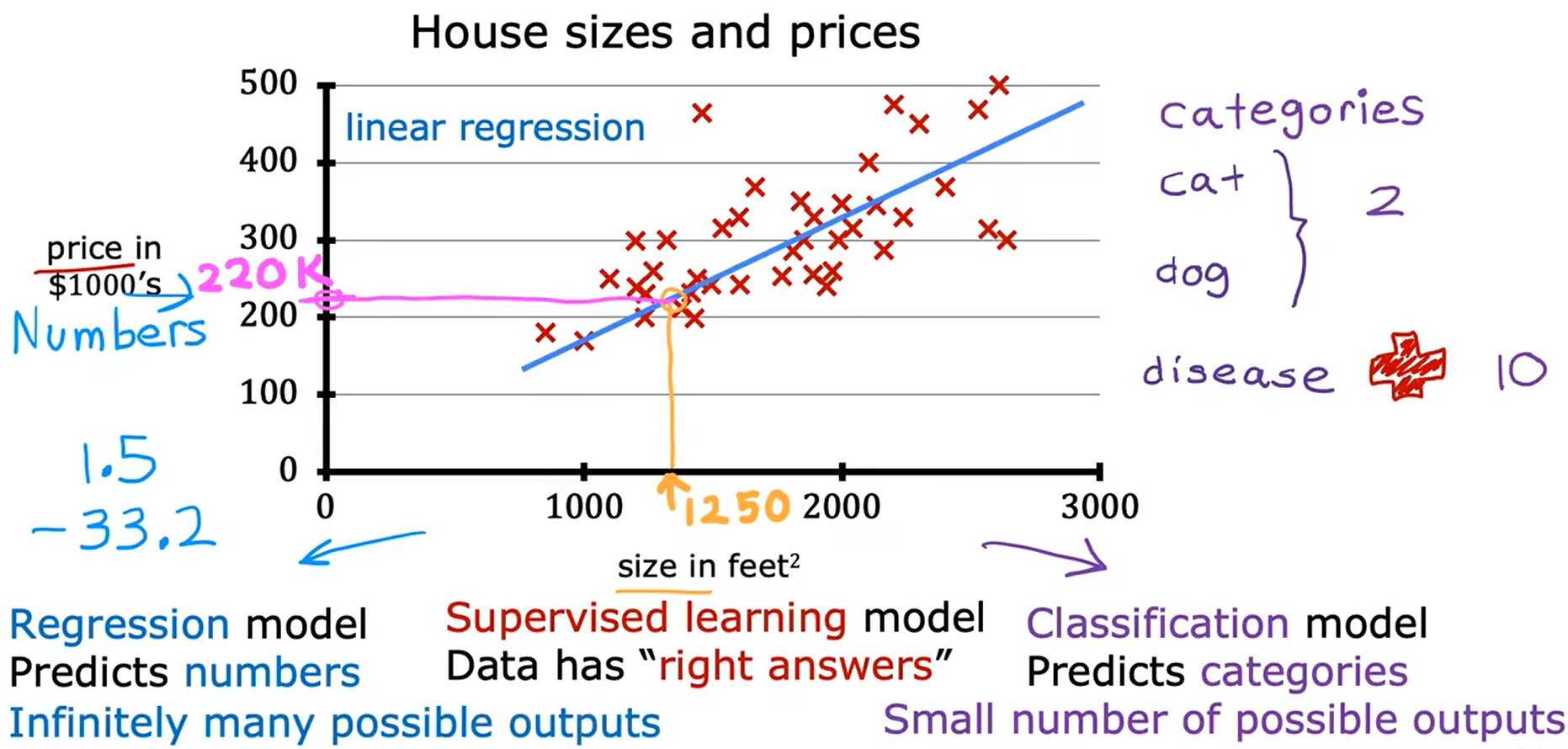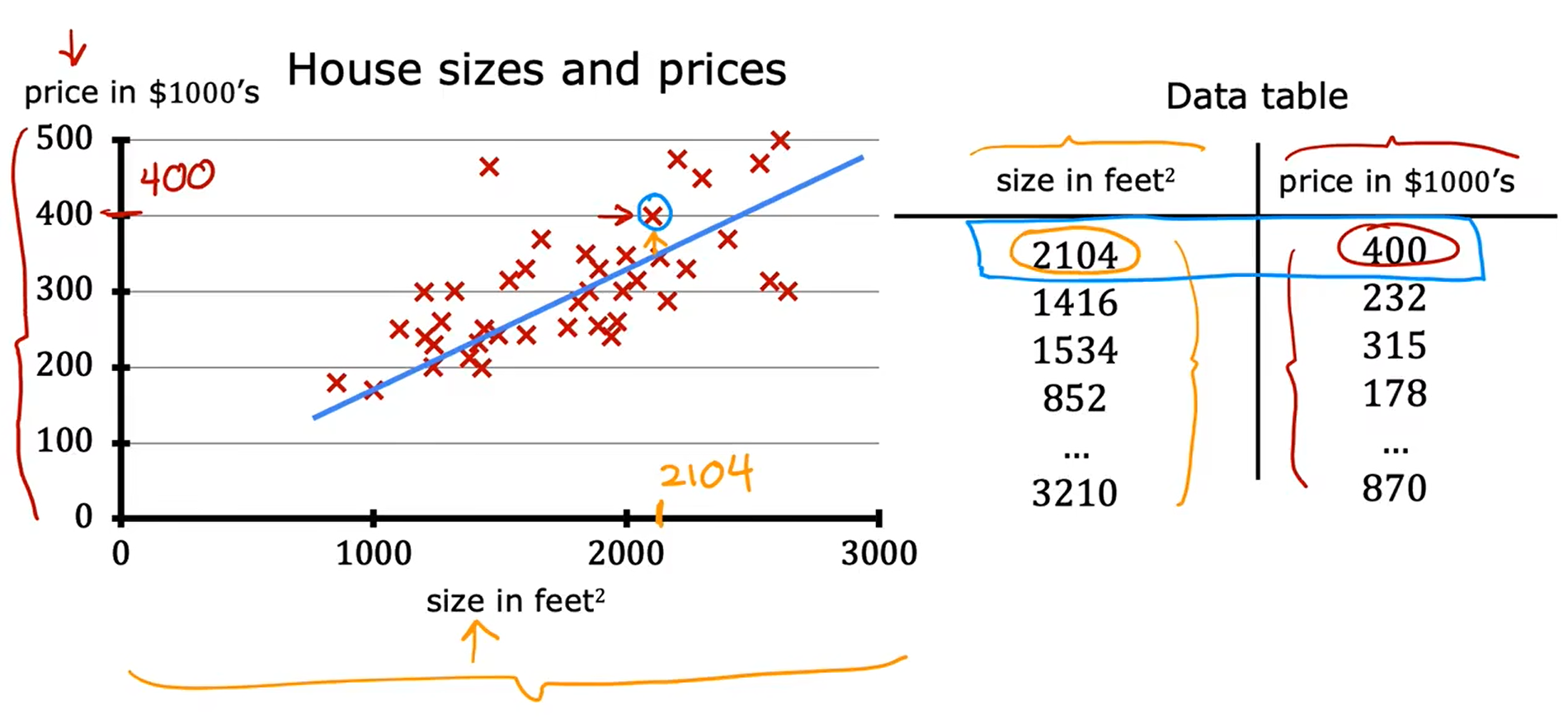## 术语

• 用来训练模型的数据集叫作 训练集
• 输入变量也叫作特征或者 输入特征
• 输出变量（目标变量、输出目标）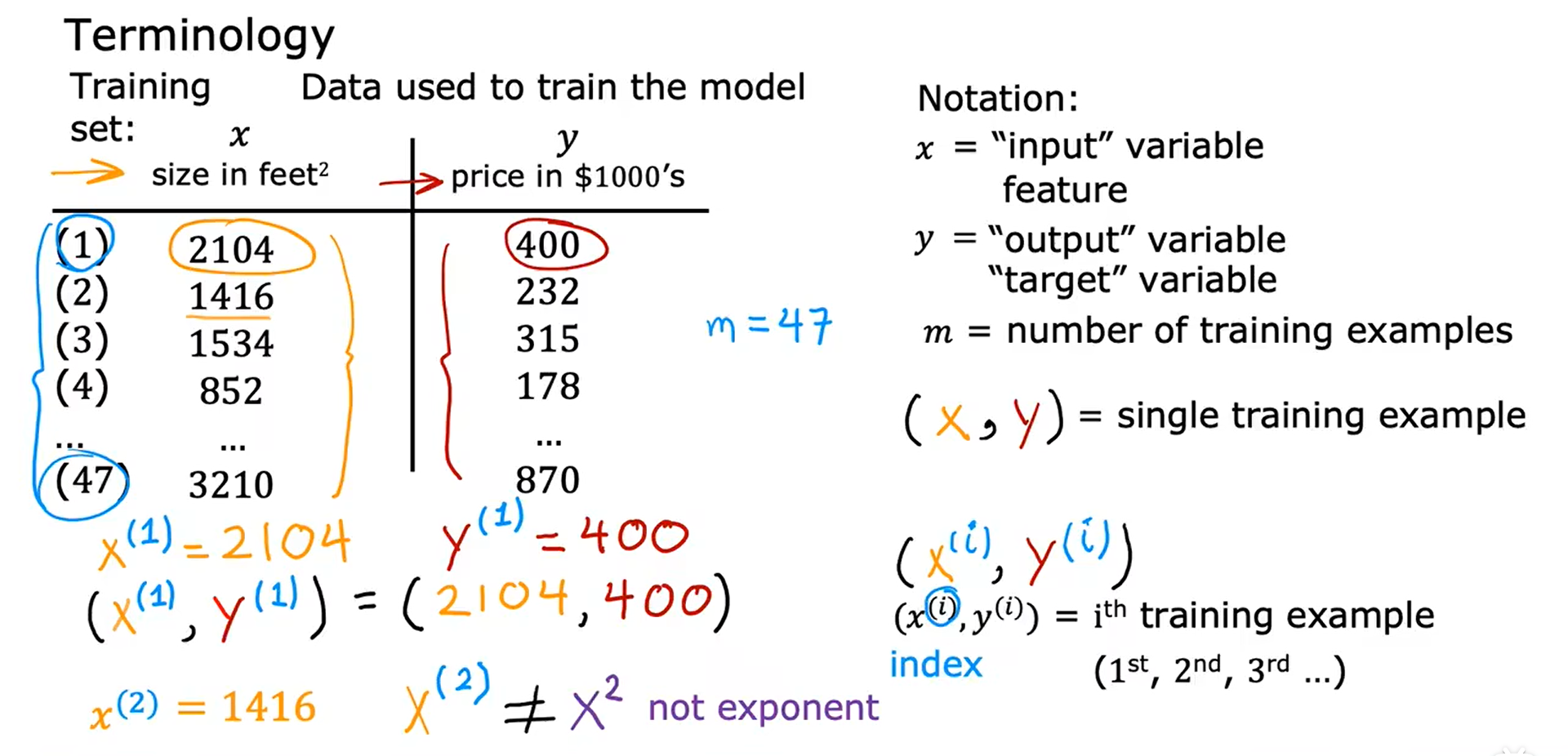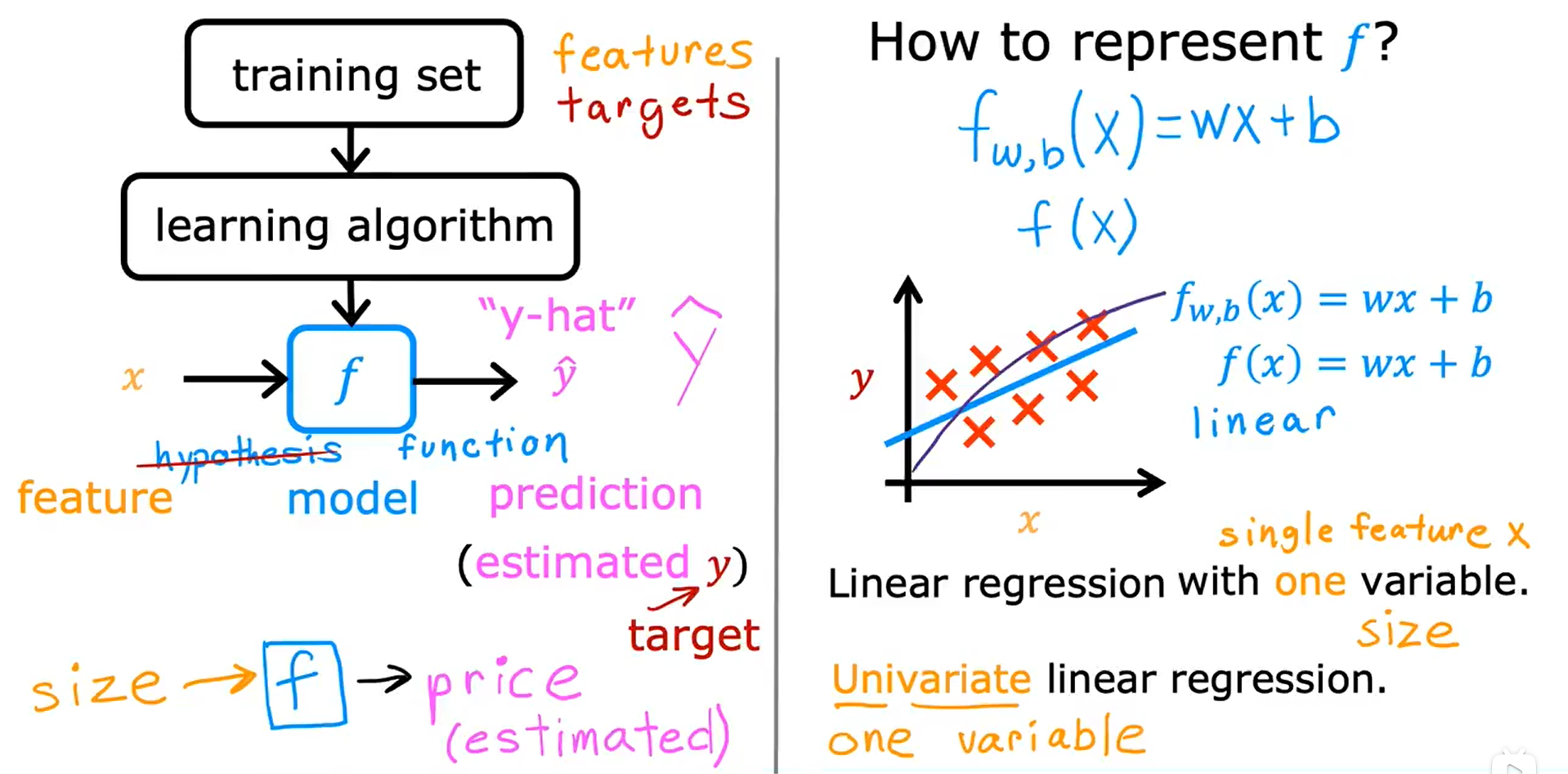## 成本（代价）函数 Cost Function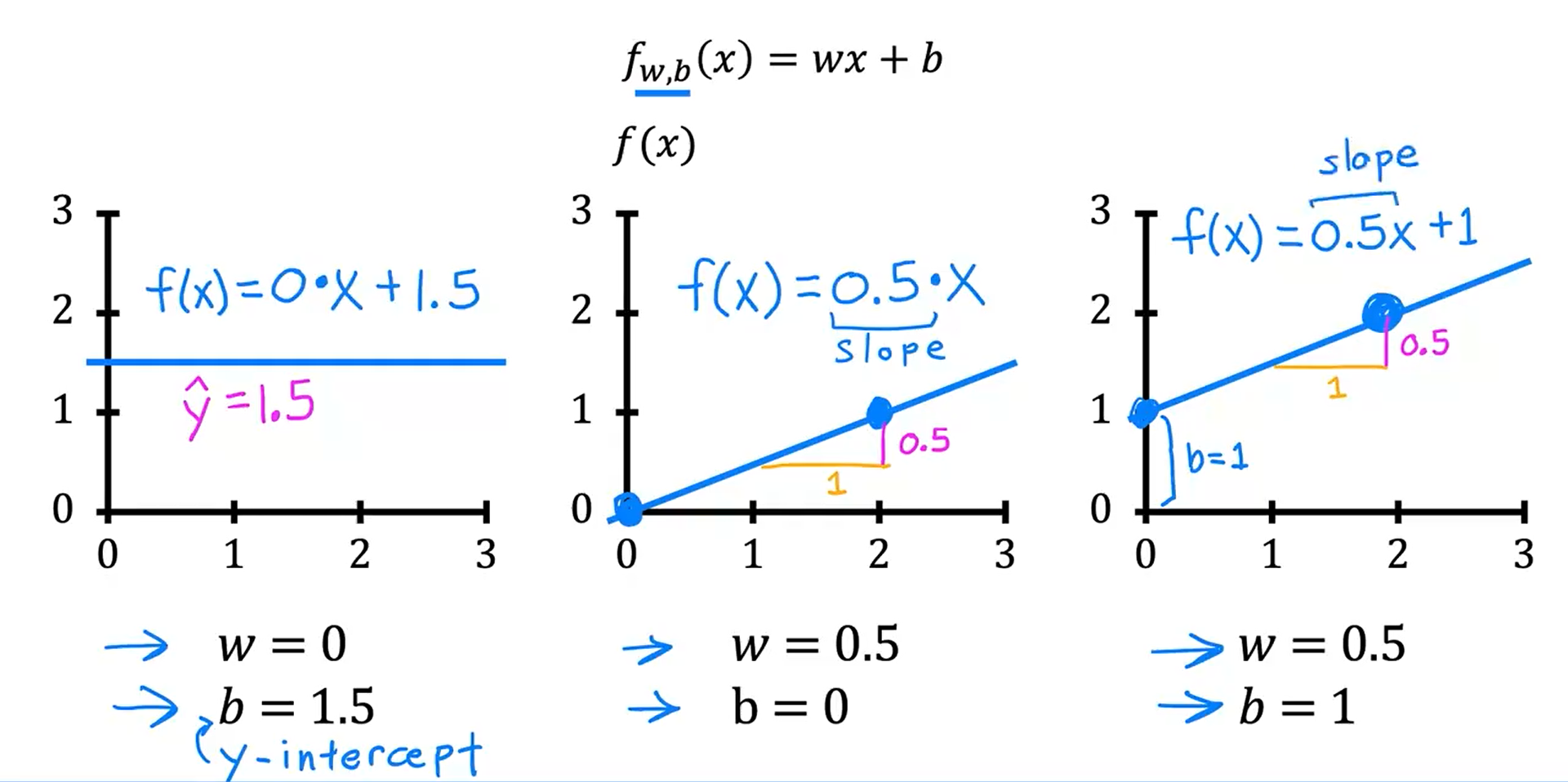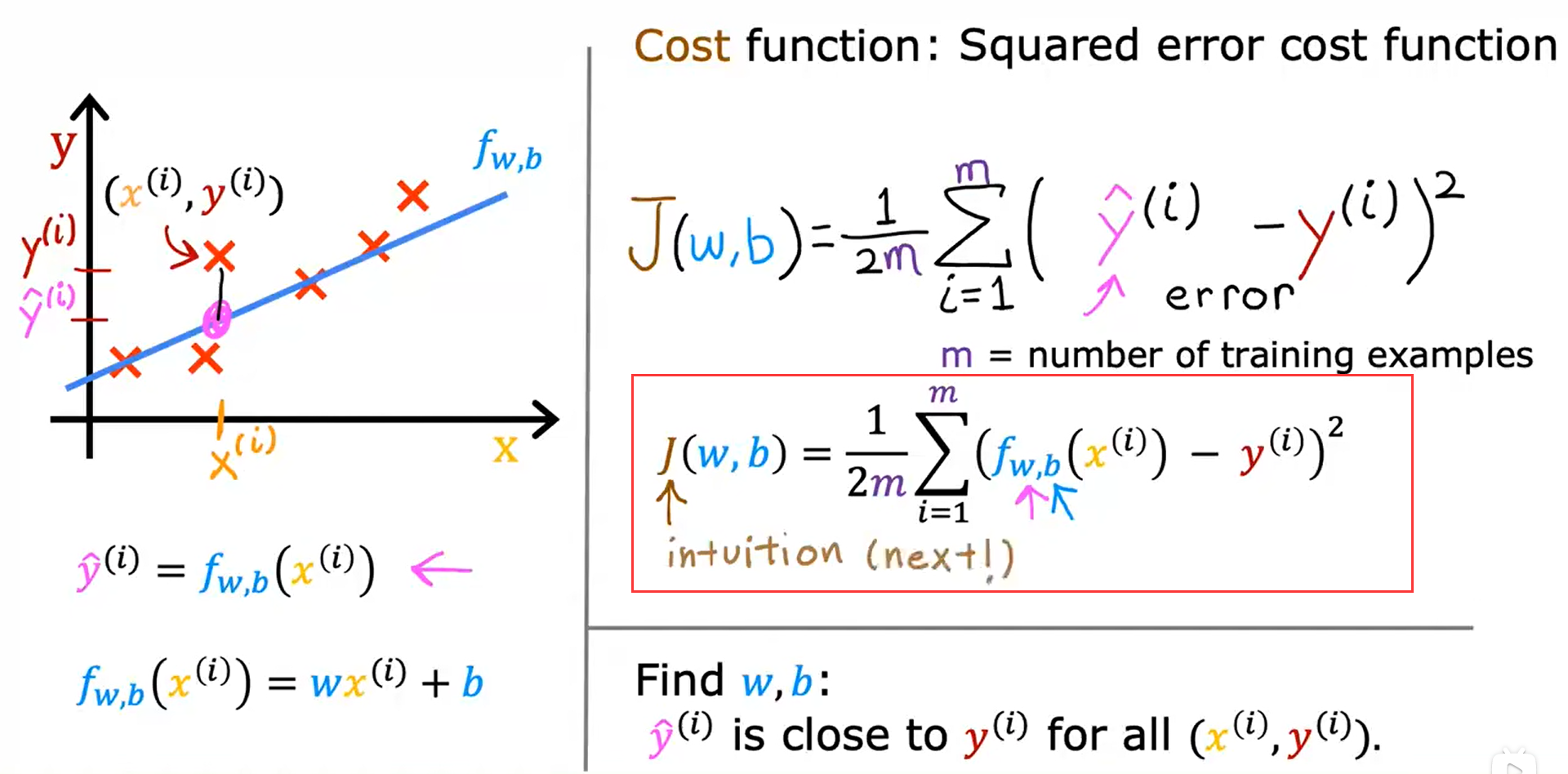## 代价函数的直观理解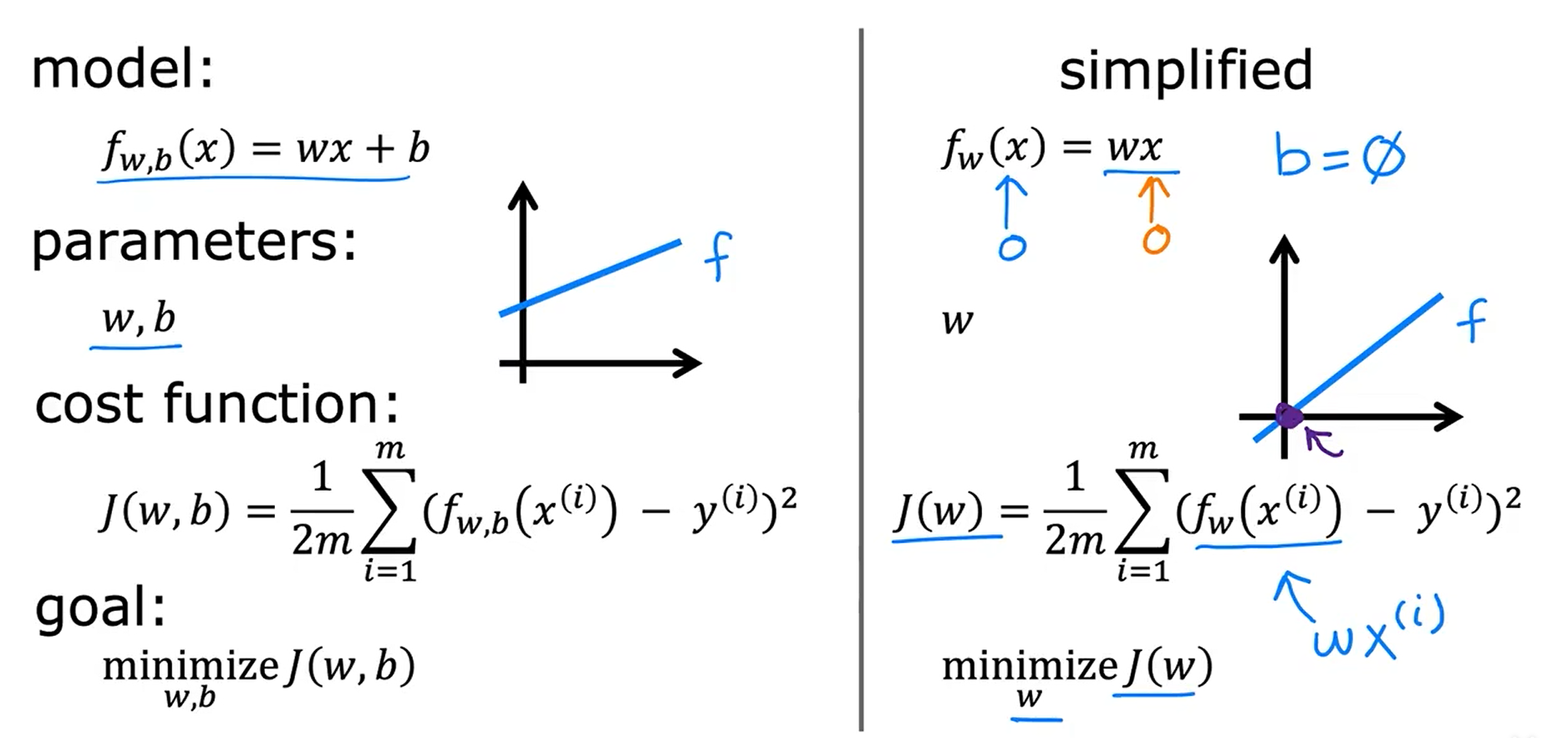w = 1 ===> J(w) = 0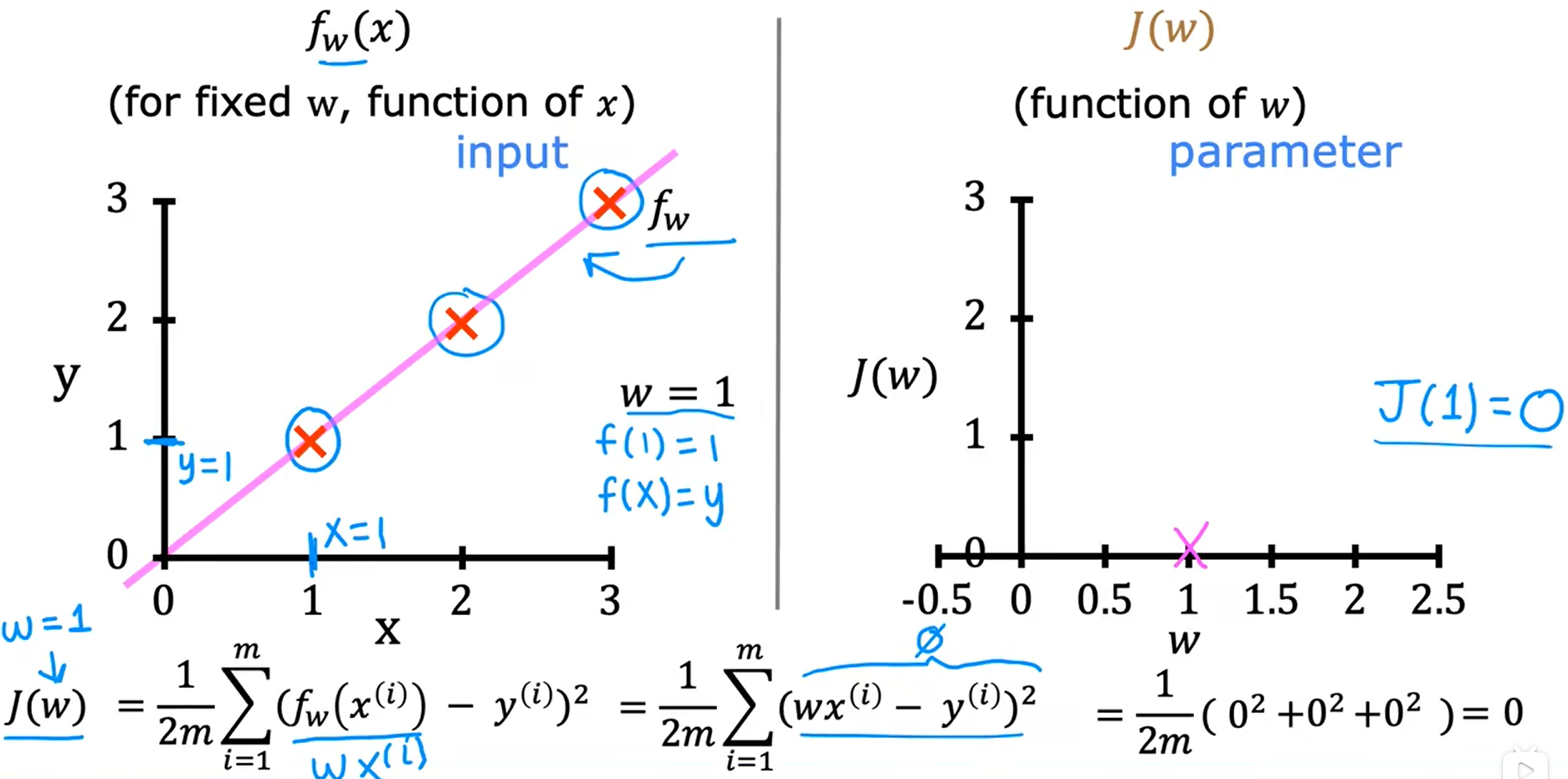w = 0.5 ===> J(w) = 0.58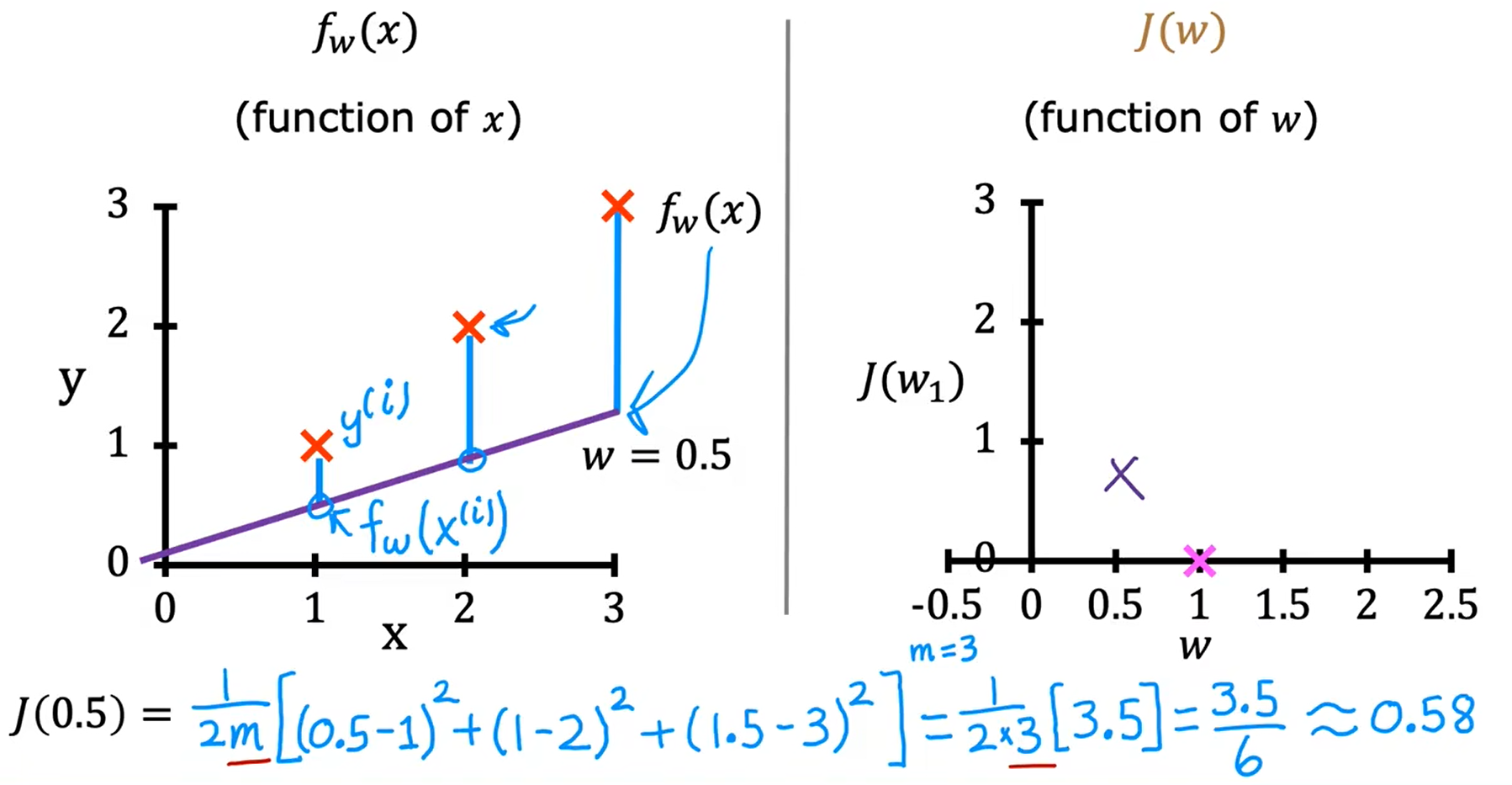w = 0 ===> J(w) = 2.3

w = -0.5 ===> J(w) = 5.25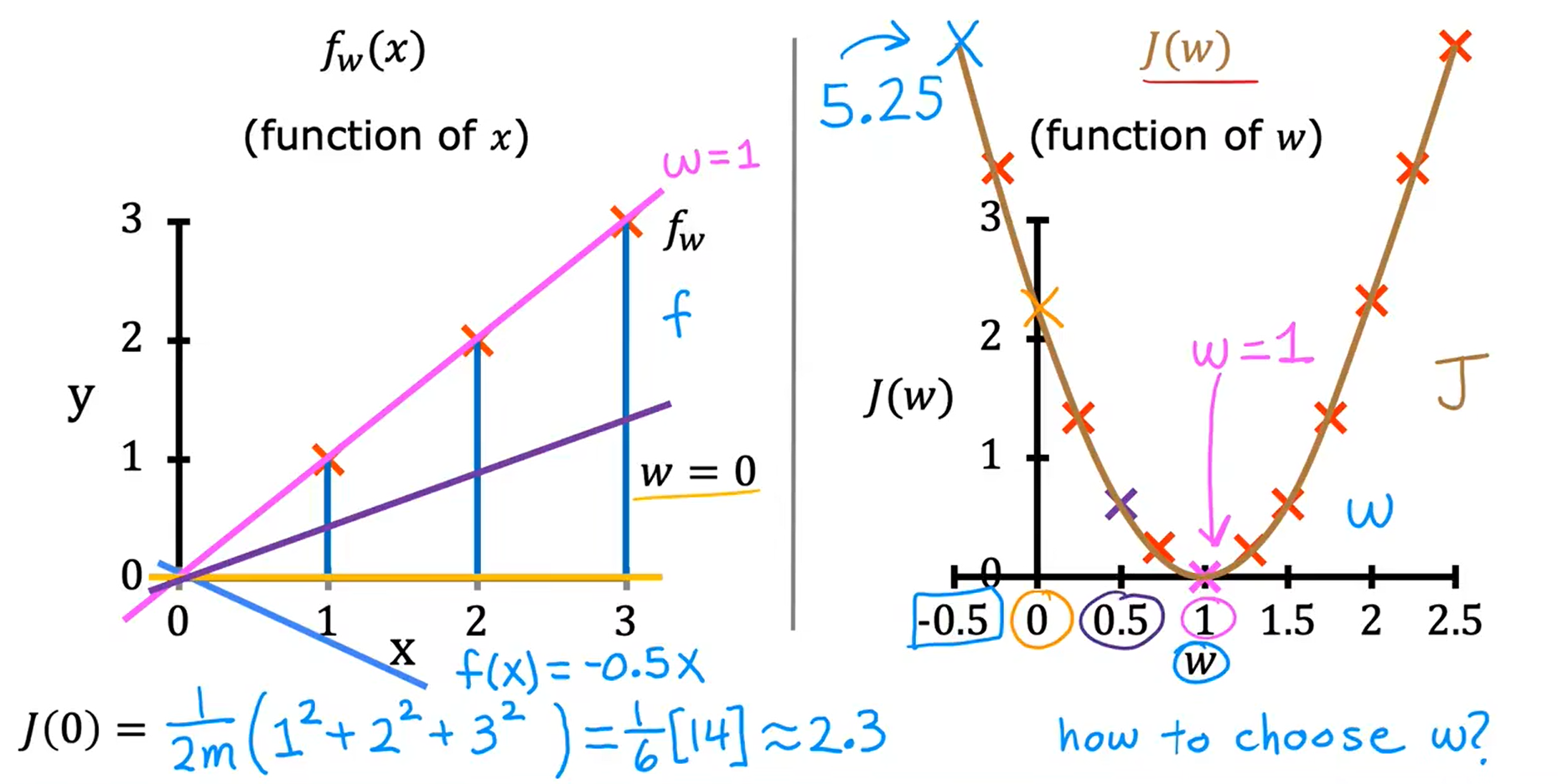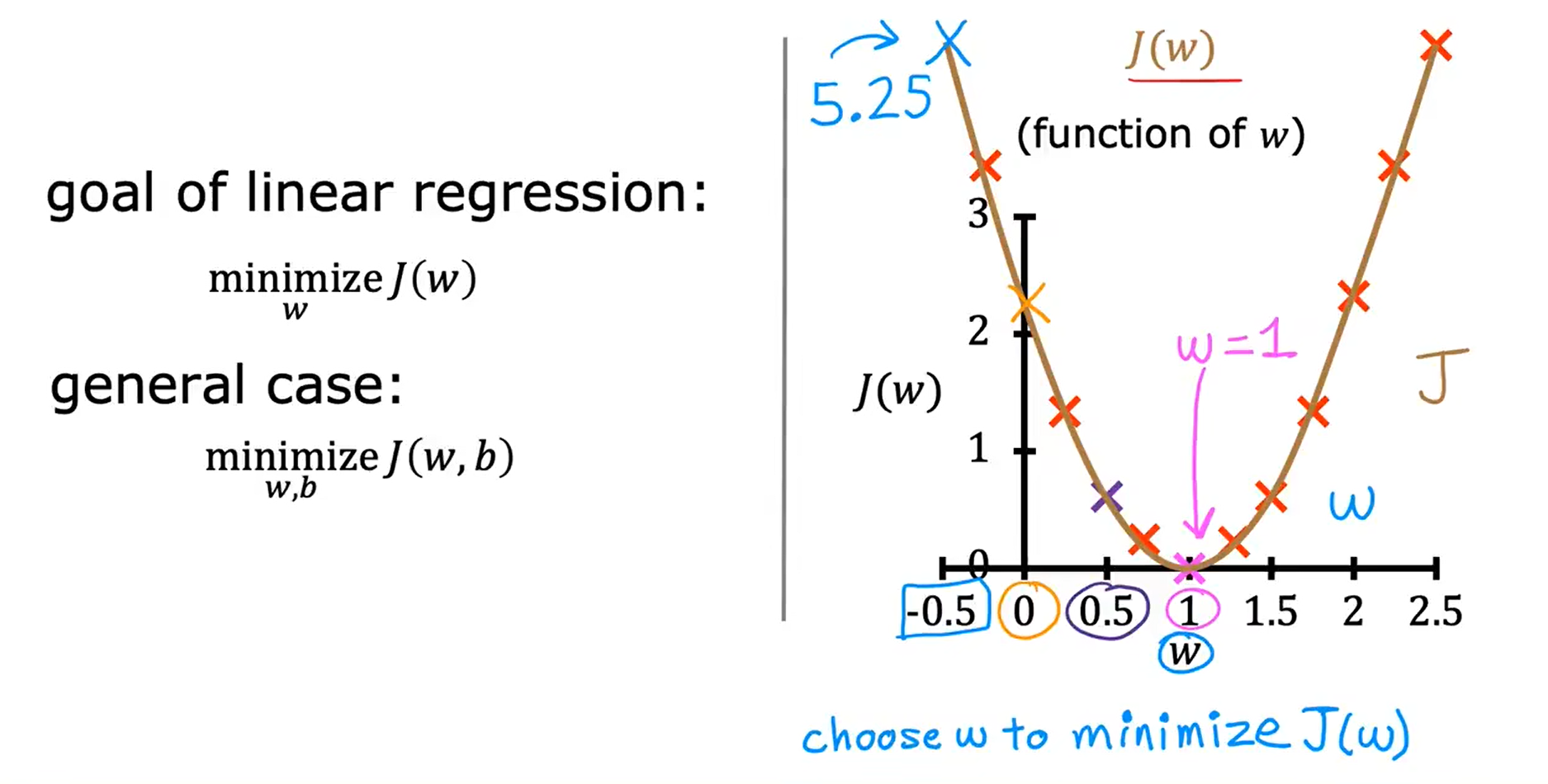## 可视化代价函数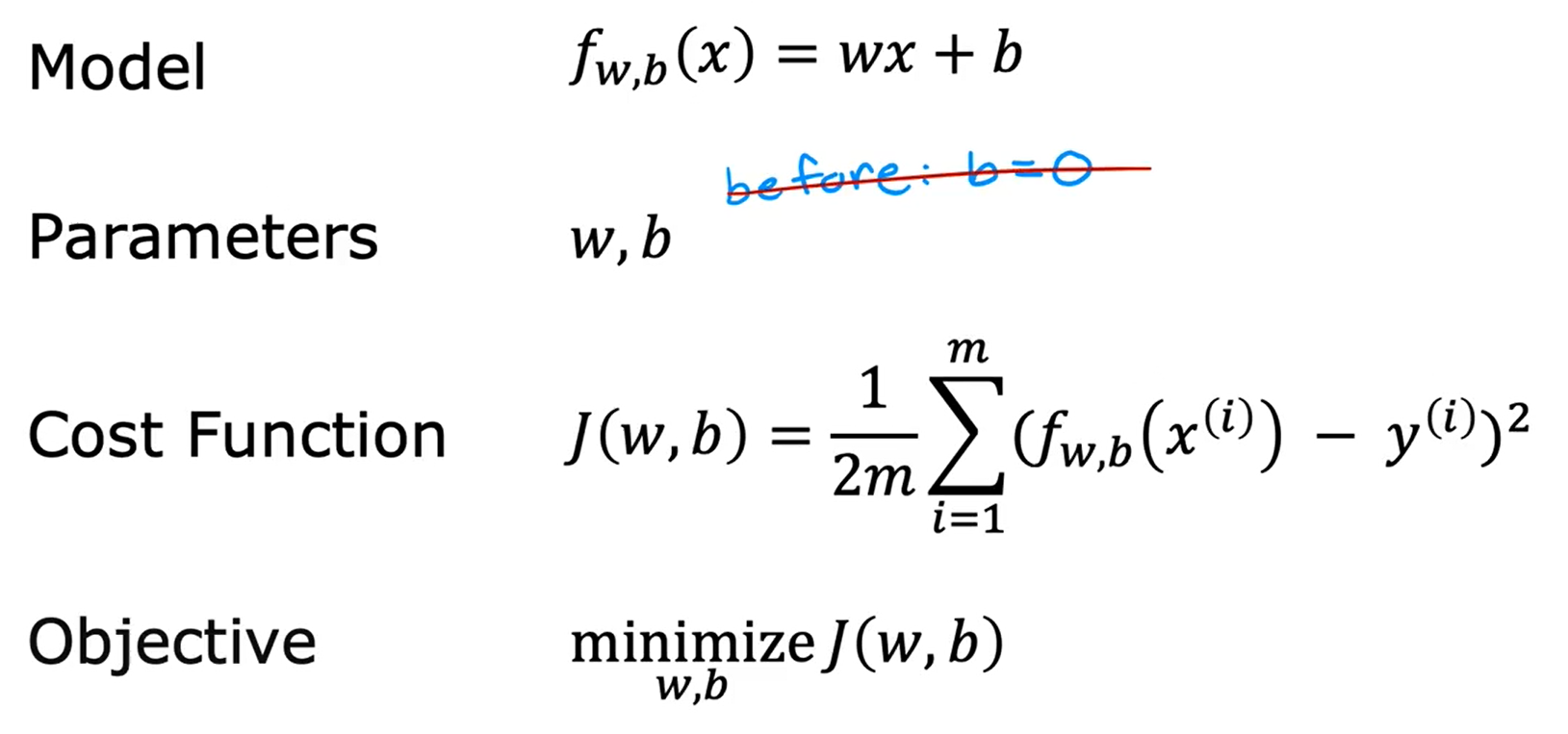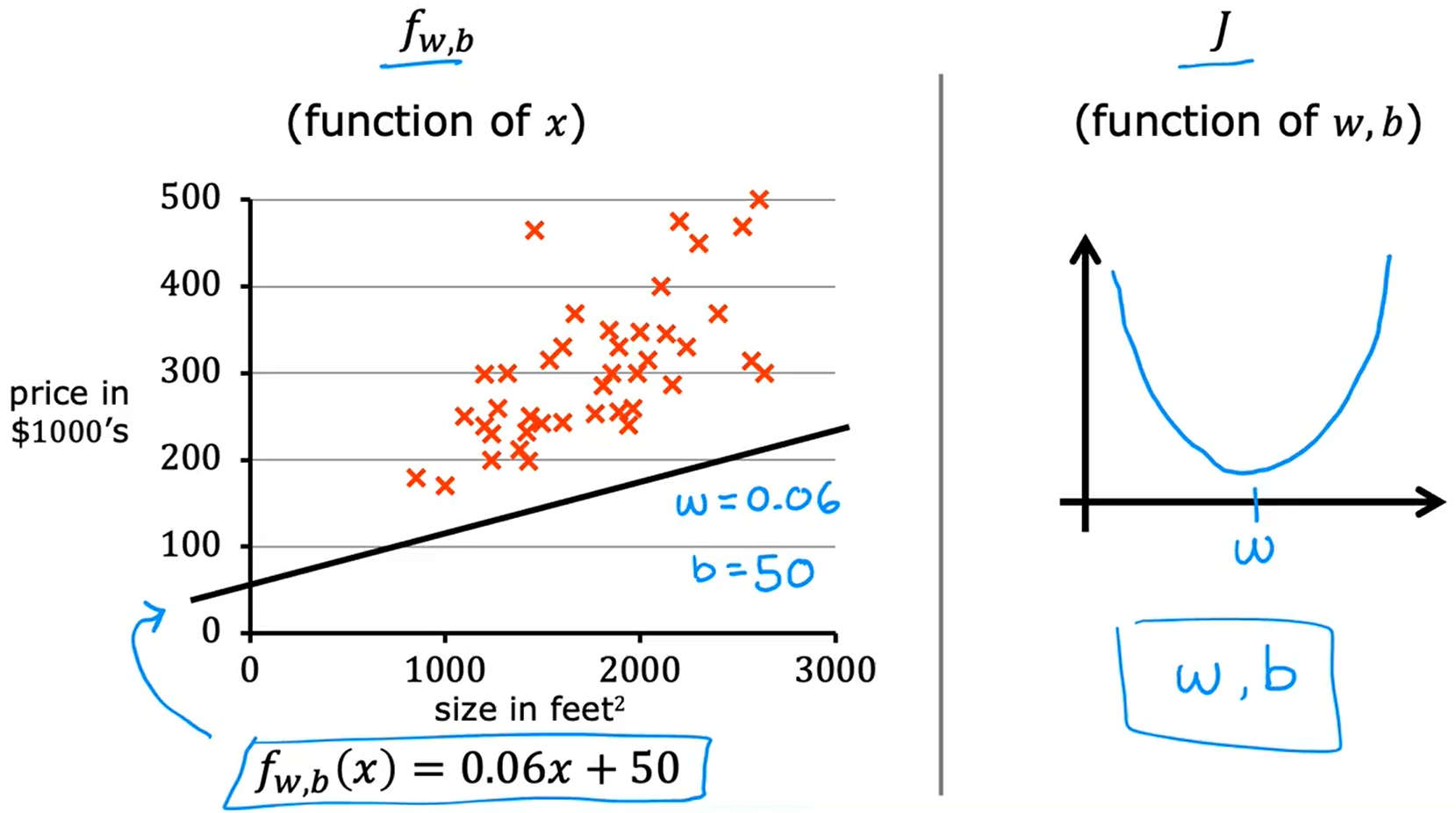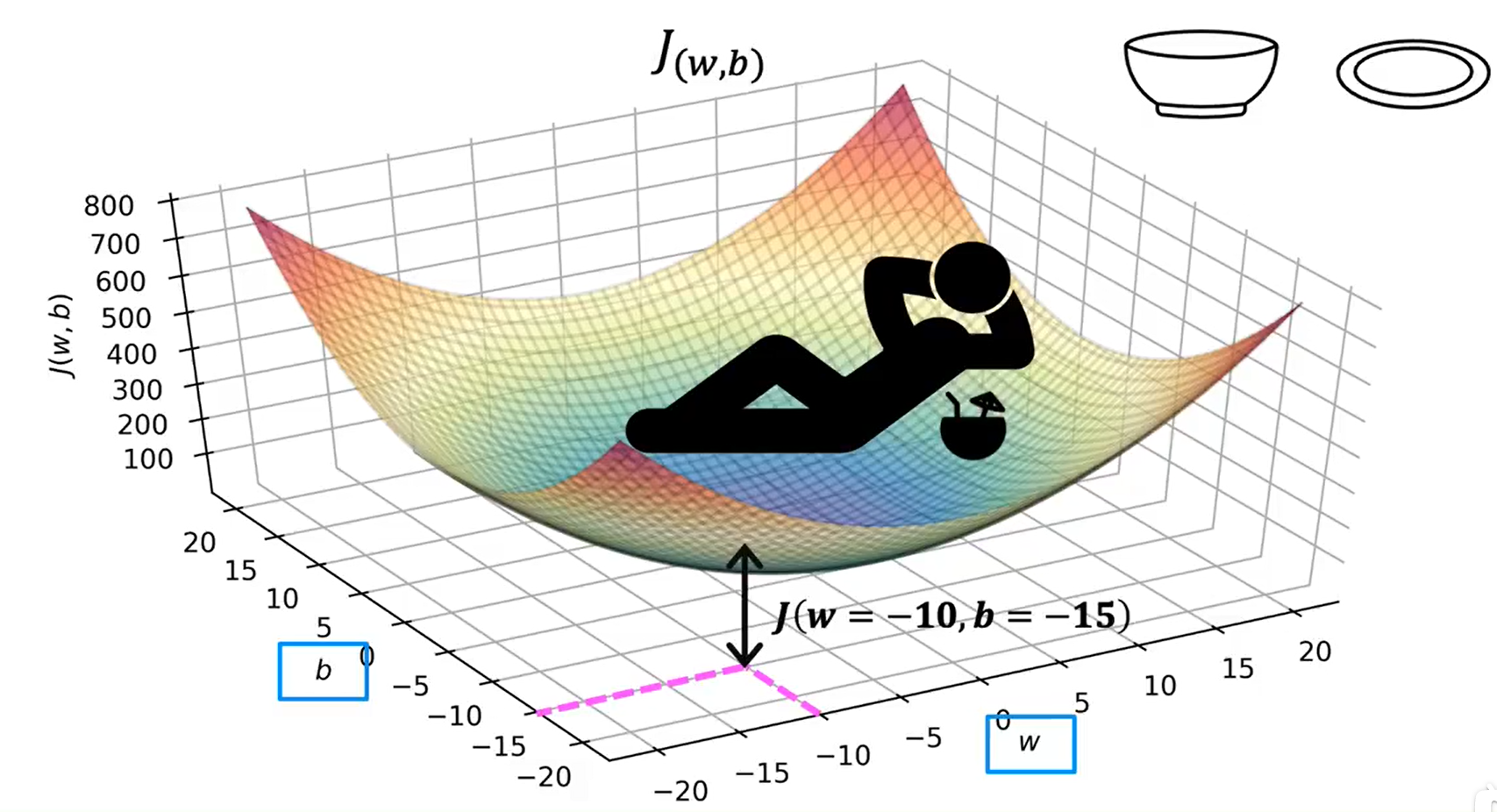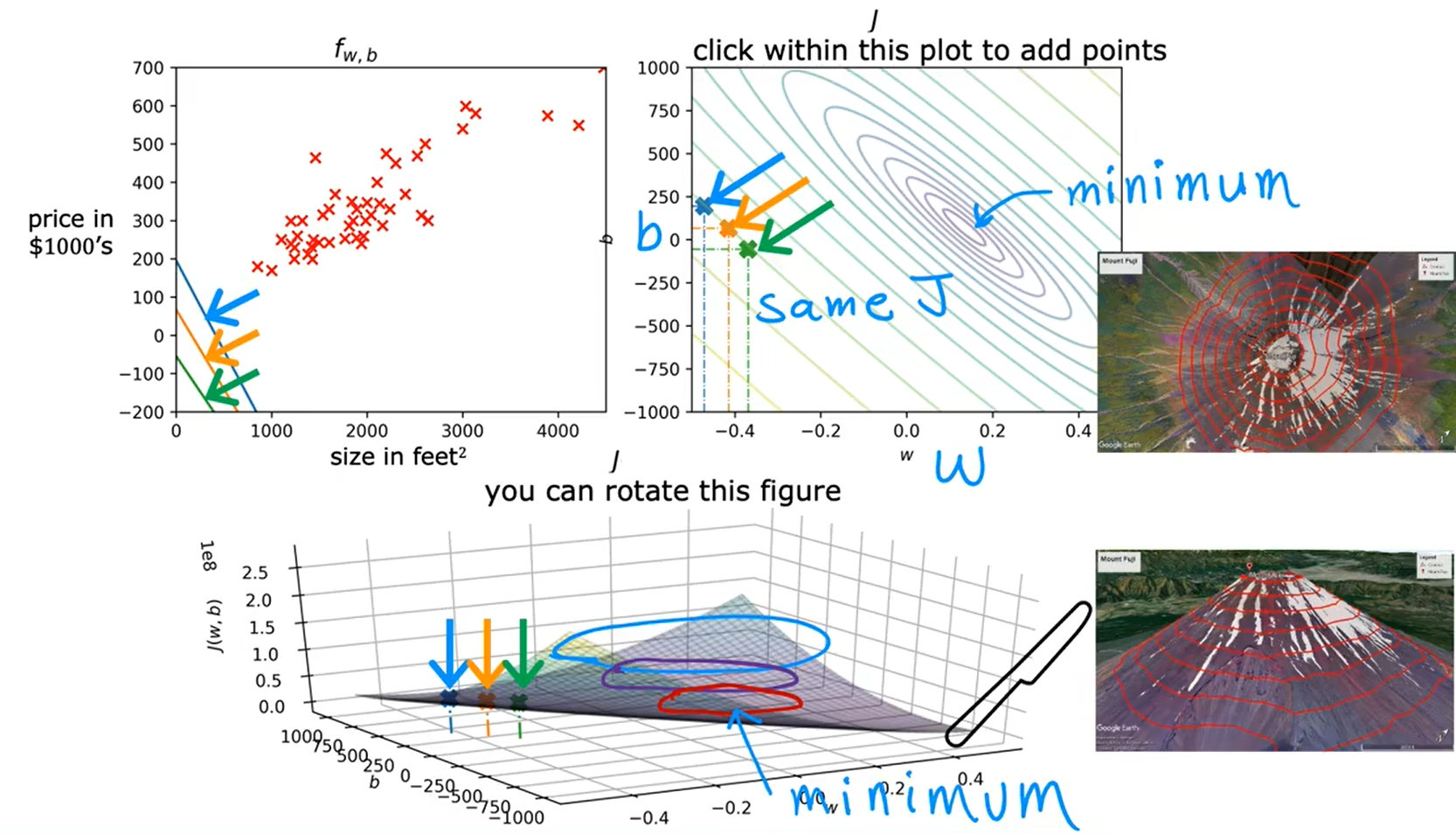• 一般先设置参数为0
• 然后逐步改变参数的值，来减少成本函数的值
• 直到我们达到或接近最小值（而且这个最低限度可能不止一个）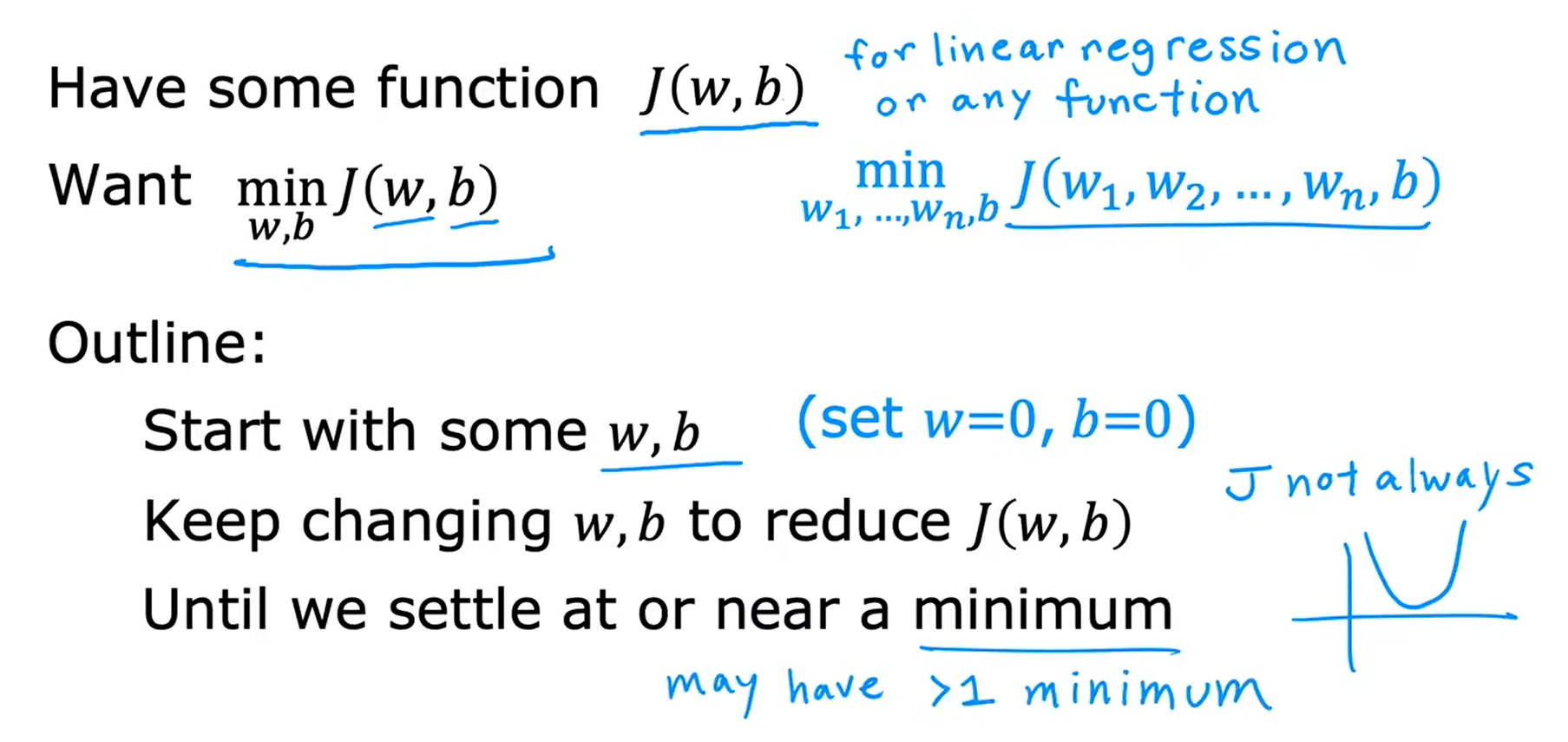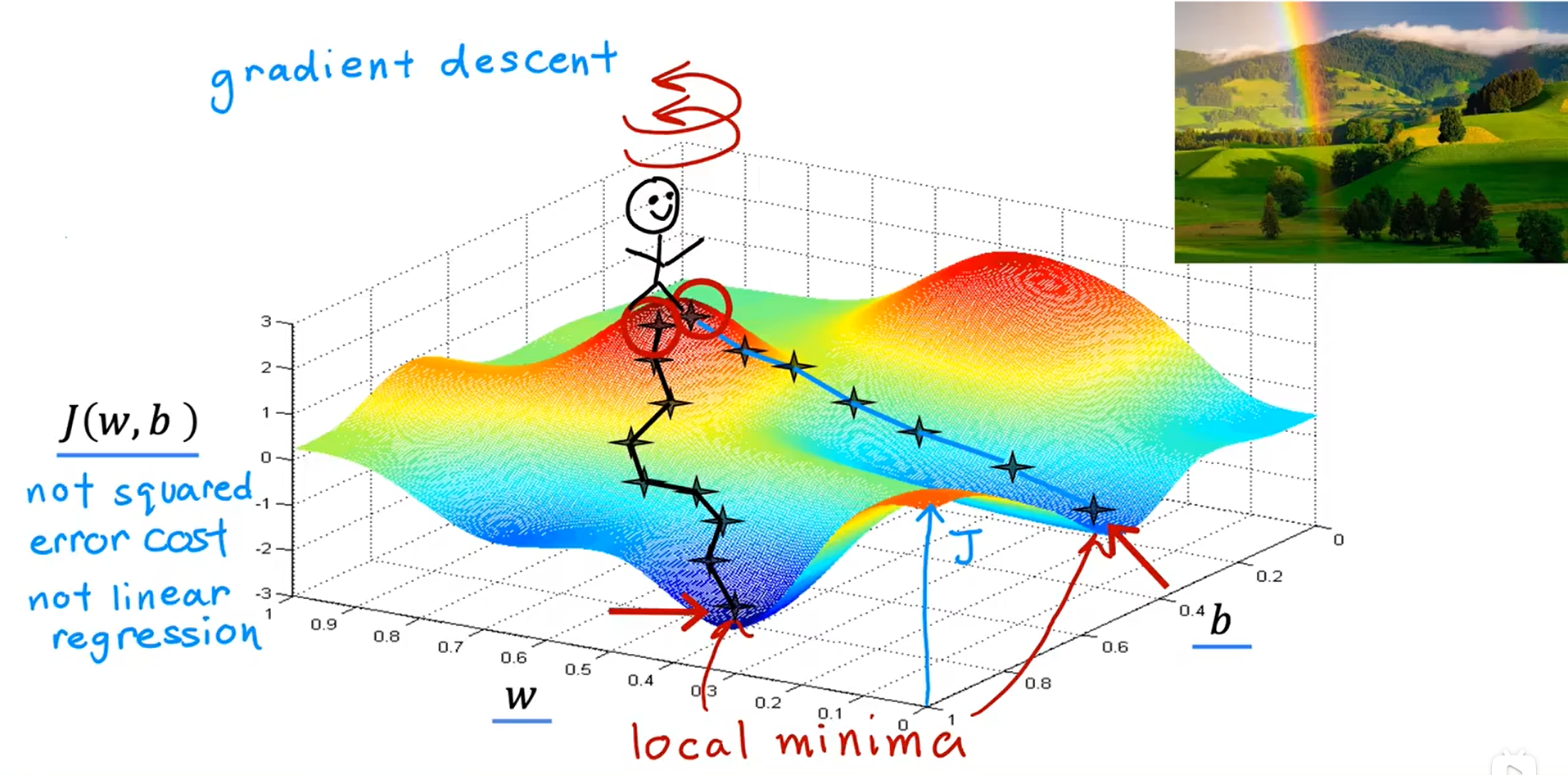## 实现梯度下降

α：学习率（Learning Rate），学习率通常是0到1之间的一个数，比如0.1 0.2；这个变量控制下坡时的步幅，在这个例子中即是更行模型参数 w 和 b 时迈出的步子的大小。
∂J(w, b) / ∂w：成本函数的（偏）导数项，下坡时的方向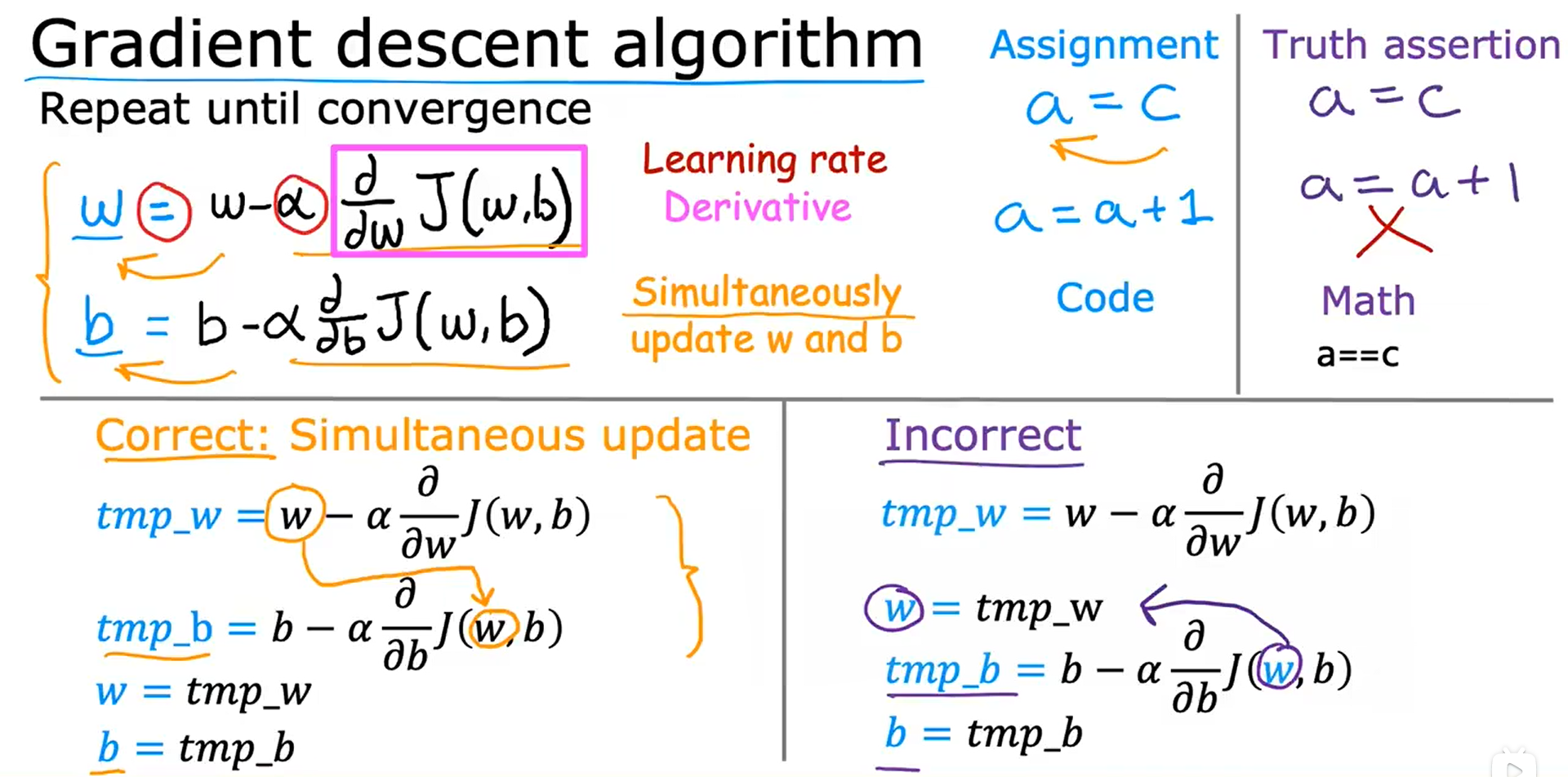## 梯度下降的直观理解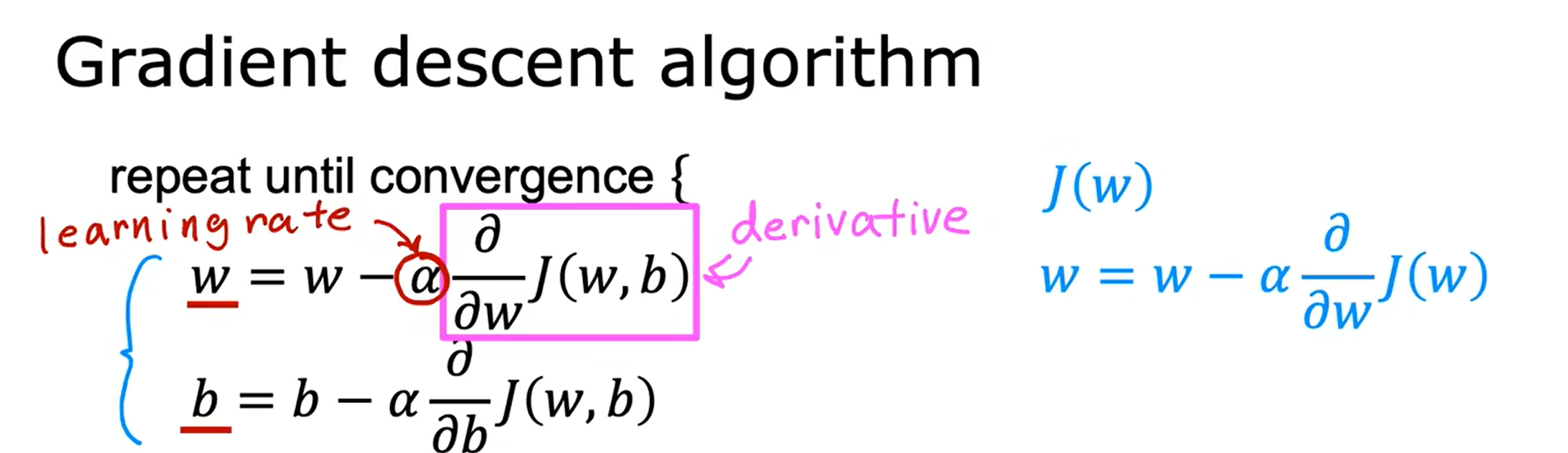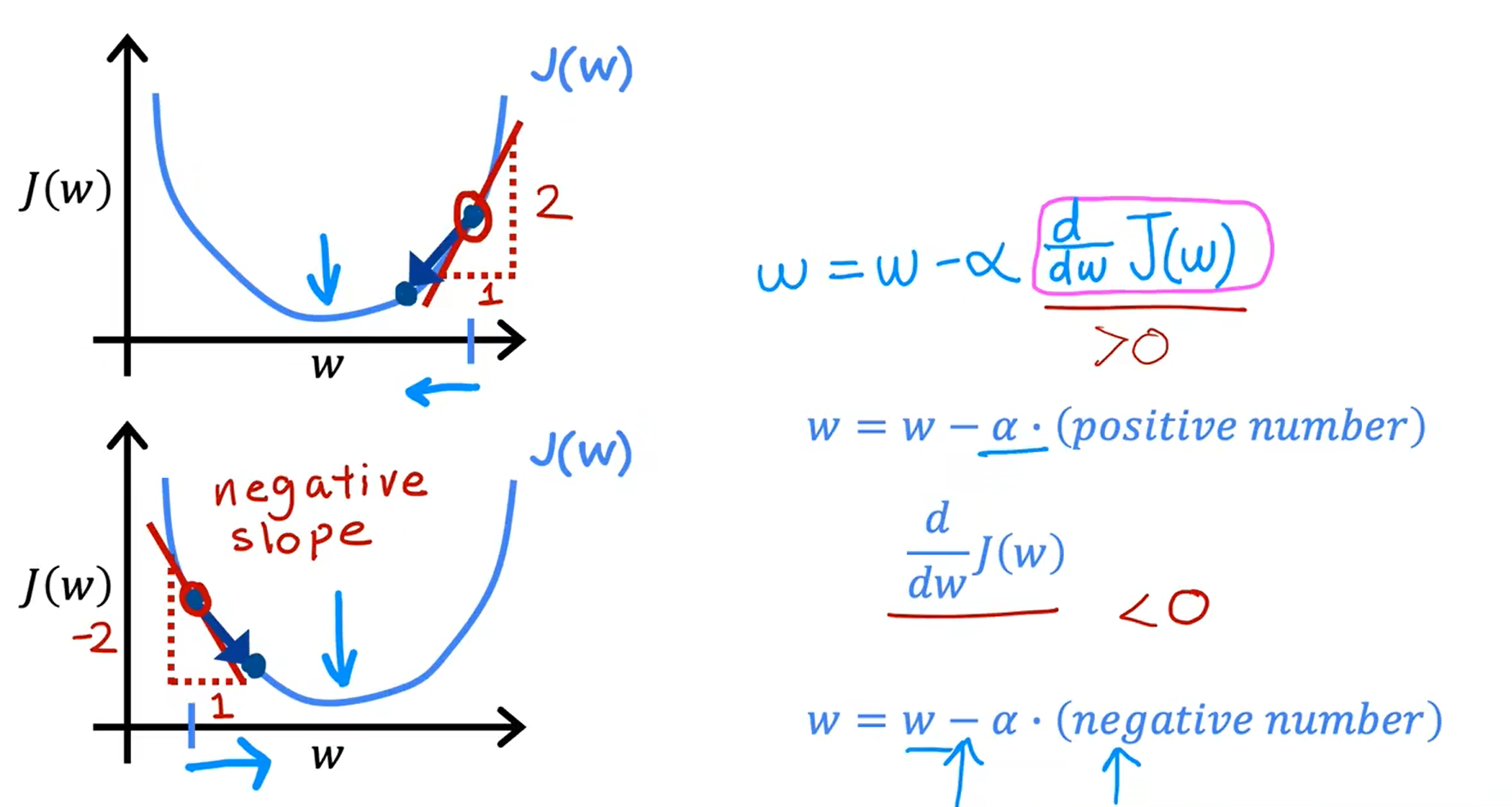## 学习率 Learning Rate, α

• 如果学习率很小，那么梯度下降的速度会很慢，这会消耗很多的时间，因为在它接近最小值之前，它需要很多步骤，而且每一步的步幅都很小。
• 如果学习率过大，那么梯度下降可能会超过预期目标，可能永远都达不到最小（梯度下降可能无法收敛，甚至可能发散）。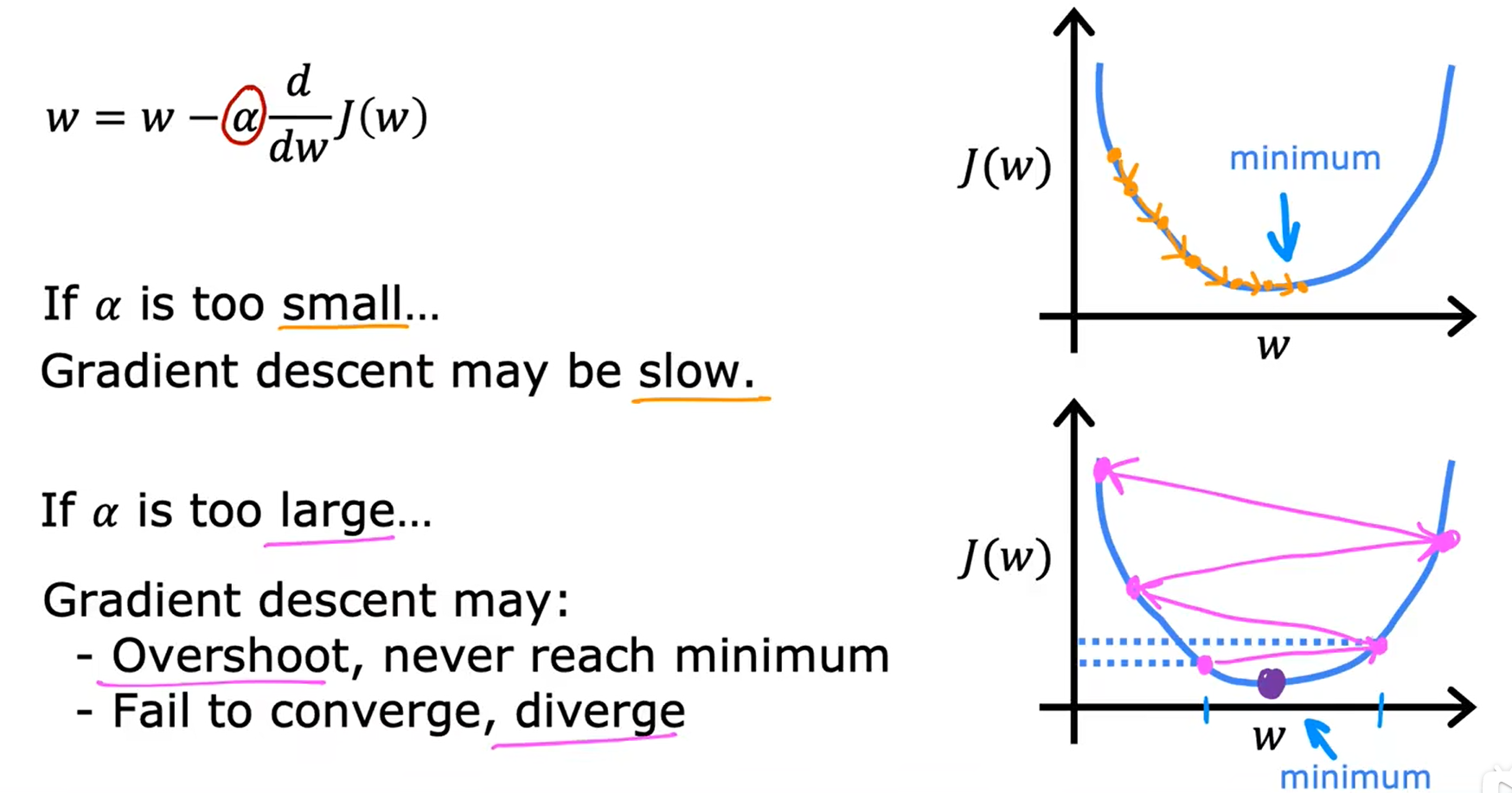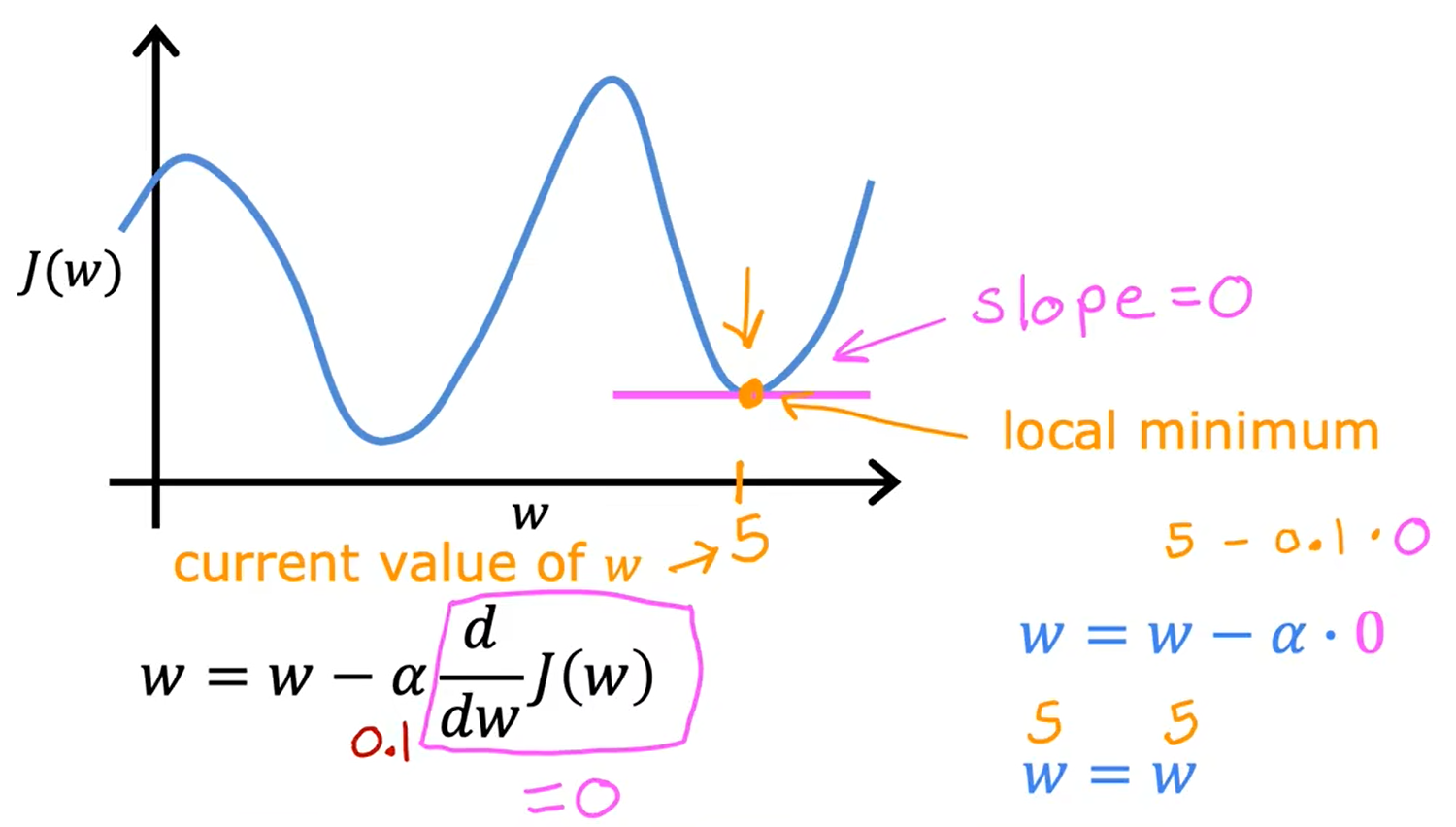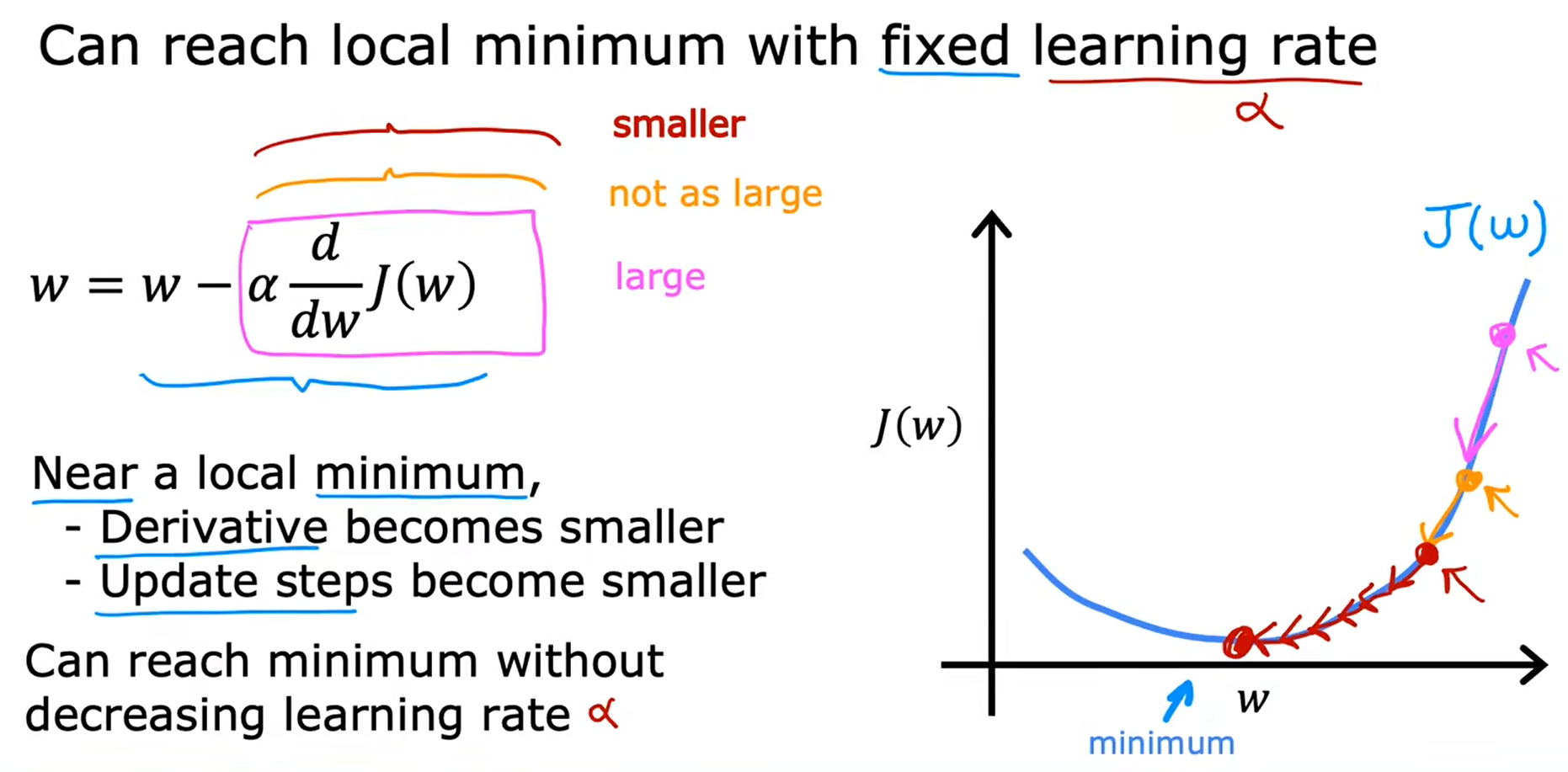## 线性回归中的梯度下降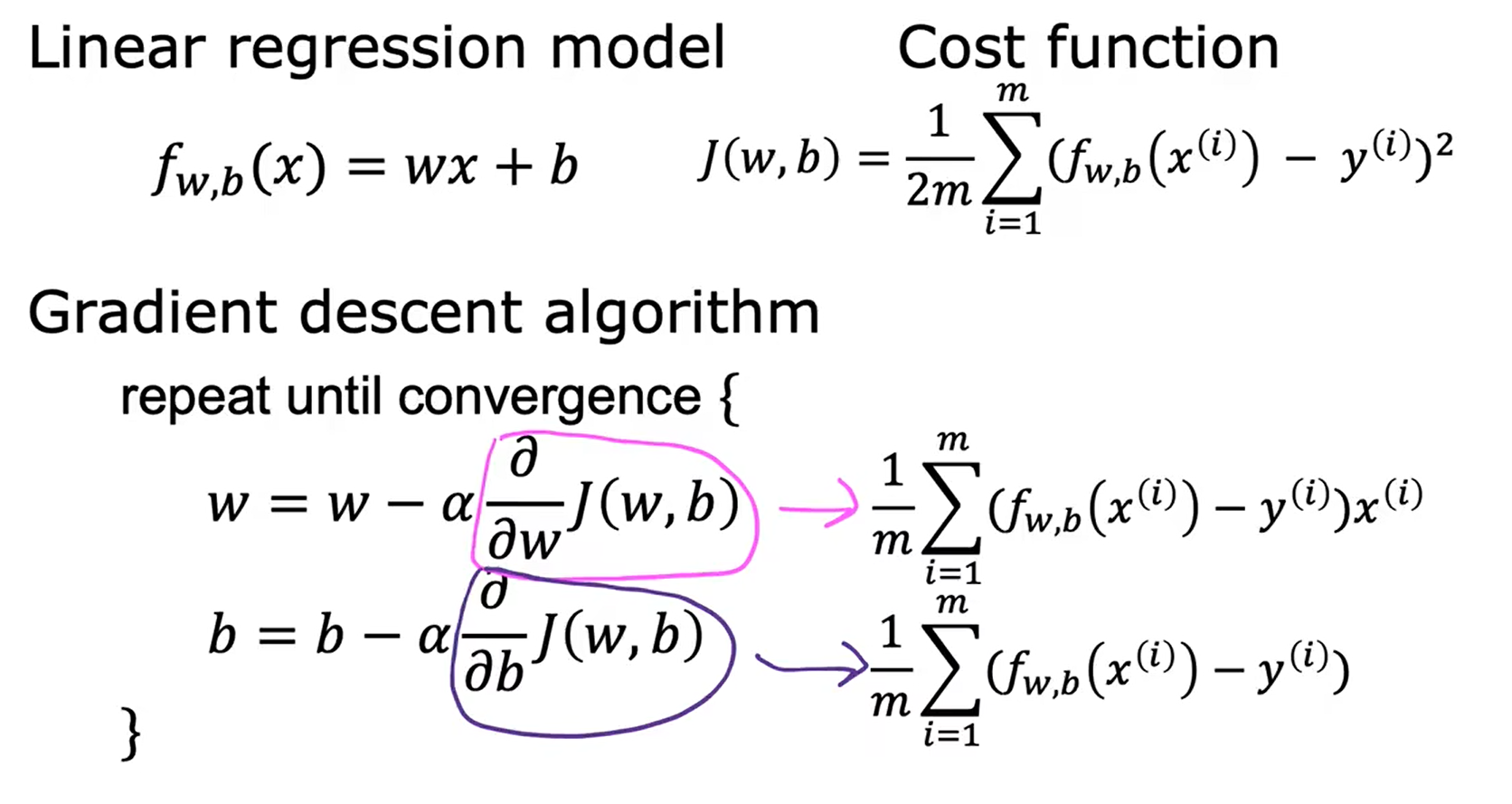∂

∂

w

J

(

w

,

b

)

=

∂

∂

w

1

2

m

∑

i

=

1

m

(

f

w

,

b

(

x

(

i

)

)

−

y

(

i

)

)

2

=

∂

∂

w

1

2

m

∑

i

=

1

m

(

w

x

(

i

)

+

b

−

y

(

i

)

)

2

=

1

2

m

∑

i

=

1

m

(

w

x

(

i

)

+

b

−

y

(

i

)

)

2

x

(

i

)

=

1

m

∑

i

=

1

m

(

f

w

,

b

(

x

(

i

)

)

−

y

(

i

)

)

x

(

i

)

\frac{∂}{∂w} J(w, b) = \frac{∂}{∂w} \frac{1}{2m} \sum_{i=1}^m (f_{w, b}(x^{(i)}) - y^{(i)}) ^2 = \\ \frac{∂}{∂w} \frac{1}{2m} \sum_{i=1}^m (wx^{(i)} + b - y^{(i)}) ^2 = \\ \frac{1}{2m} \sum_{i=1}^m (wx^{(i)} + b - y^{(i)}) 2x^{(i)} = \\ \frac{1}{m} \sum_{i=1}^m (f_{w, b}(x^{(i)}) - y^{(i)}) x^{(i)}

∂w∂​J(w,b)=∂w∂​2m1​i=1∑m​(fw,b​(x(i))−y(i))2=∂w∂​2m1​i=1∑m​(wx(i)+b−y(i))2=2m1​i=1∑m​(wx(i)+b−y(i))2x(i)=m1​i=1∑m​(fw,b​(x(i))−y(i))x(i)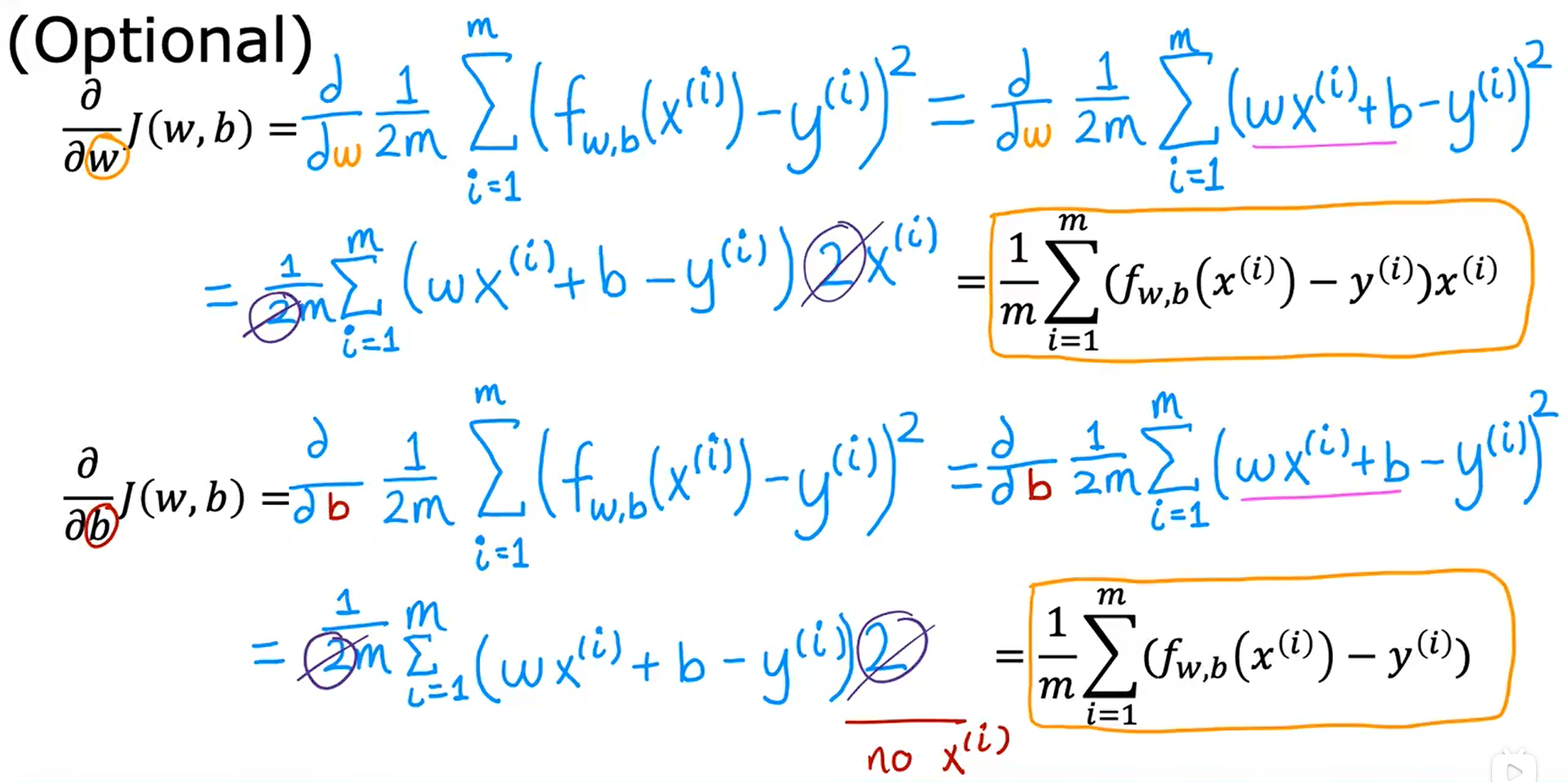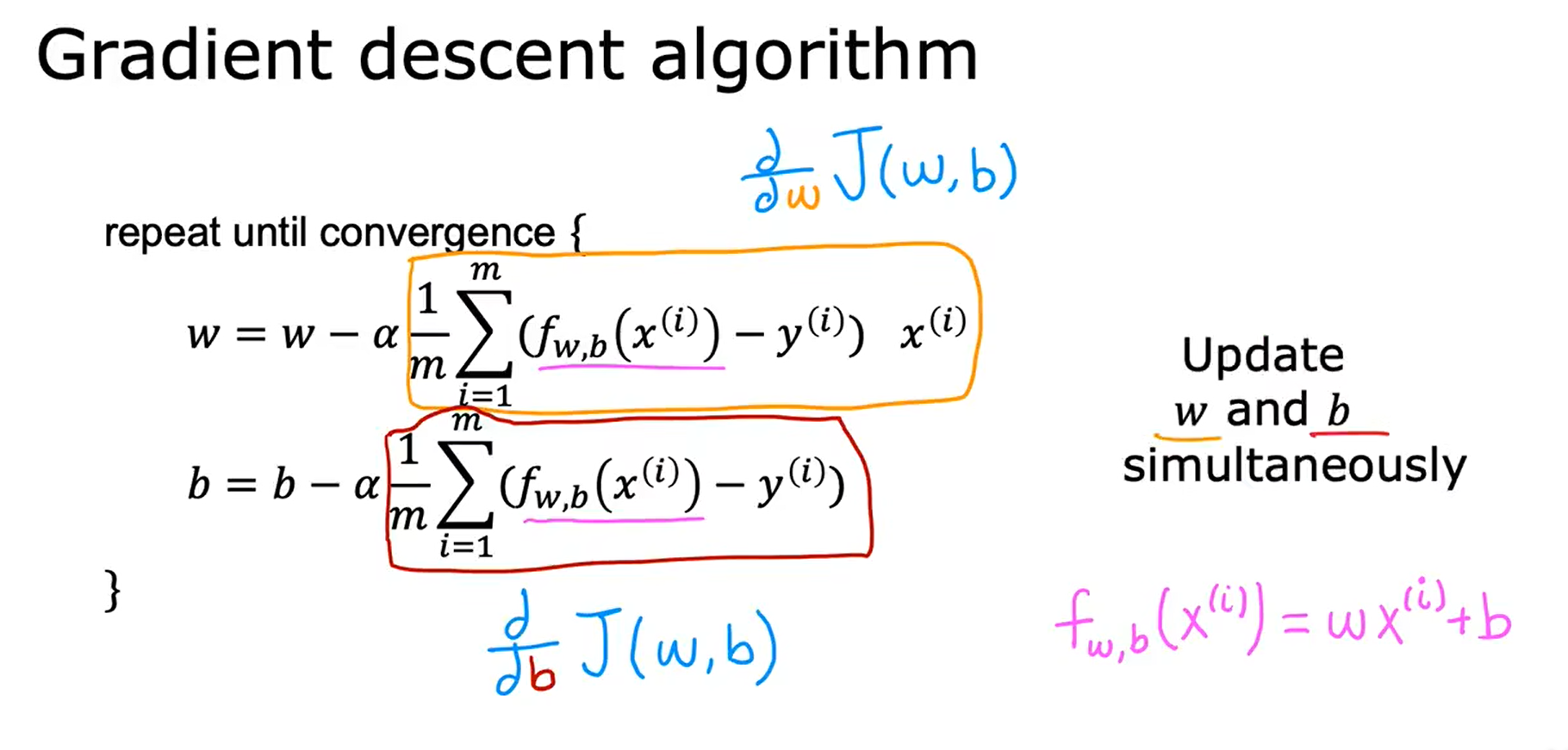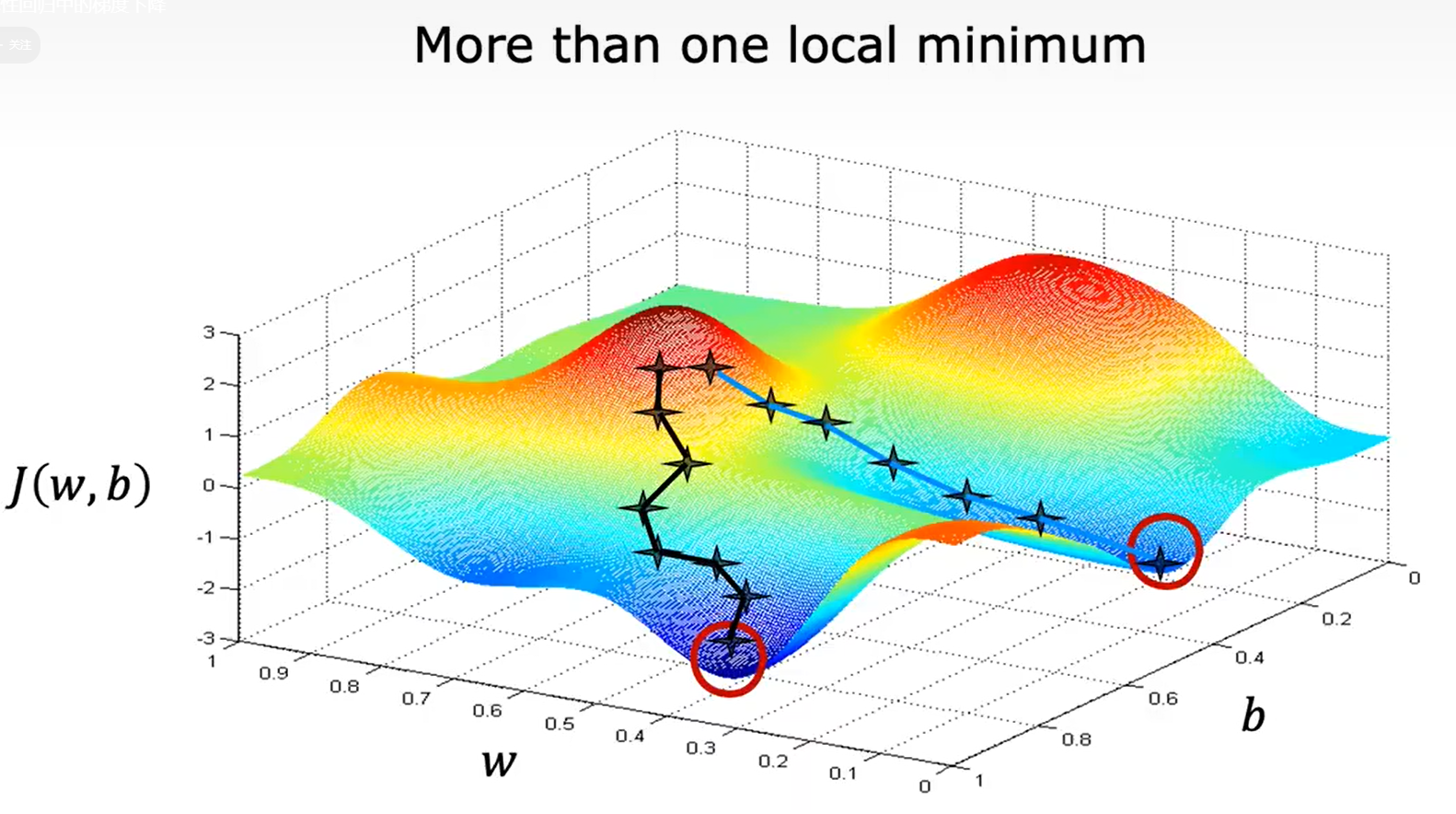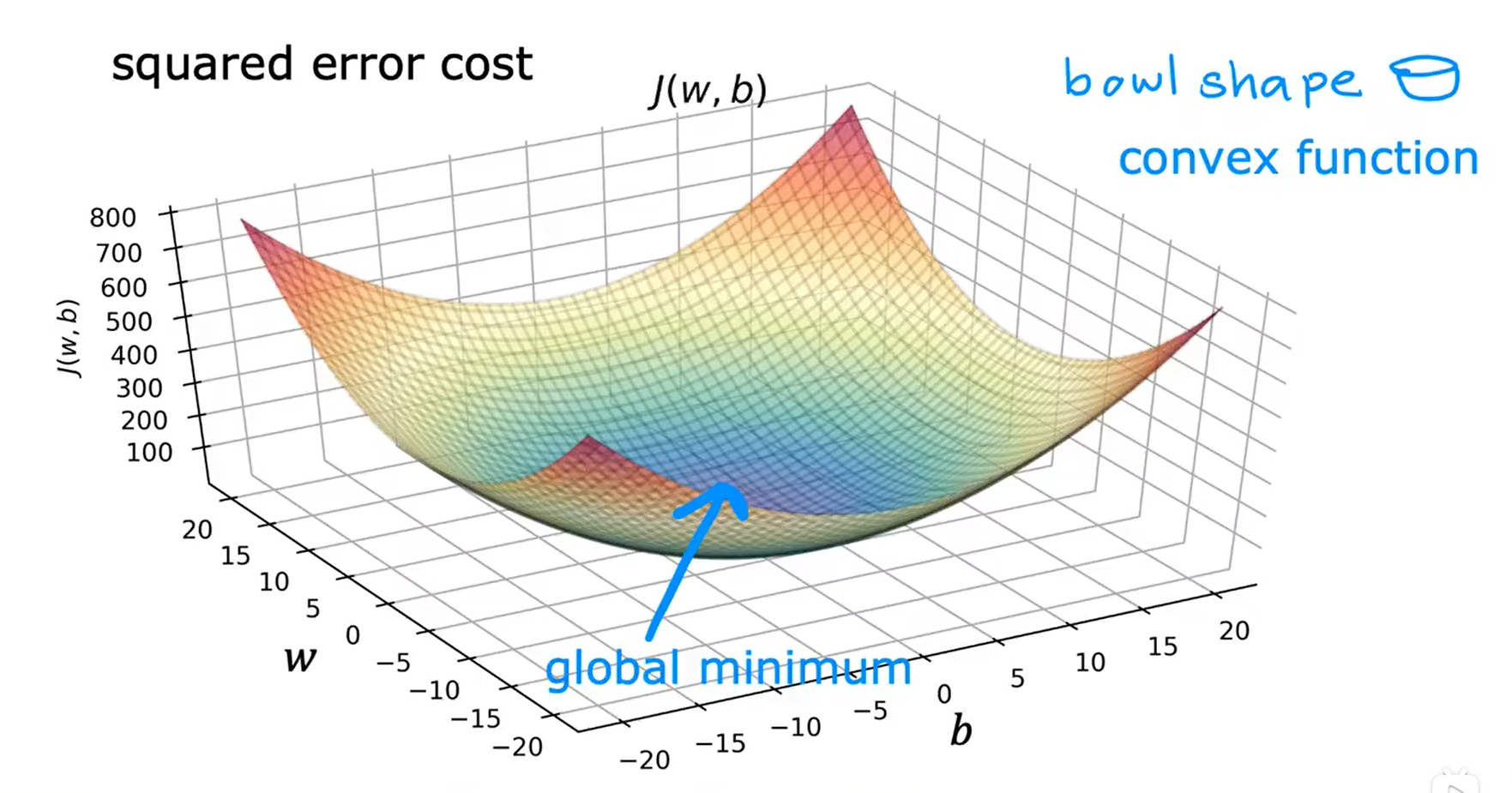## 运行梯度下降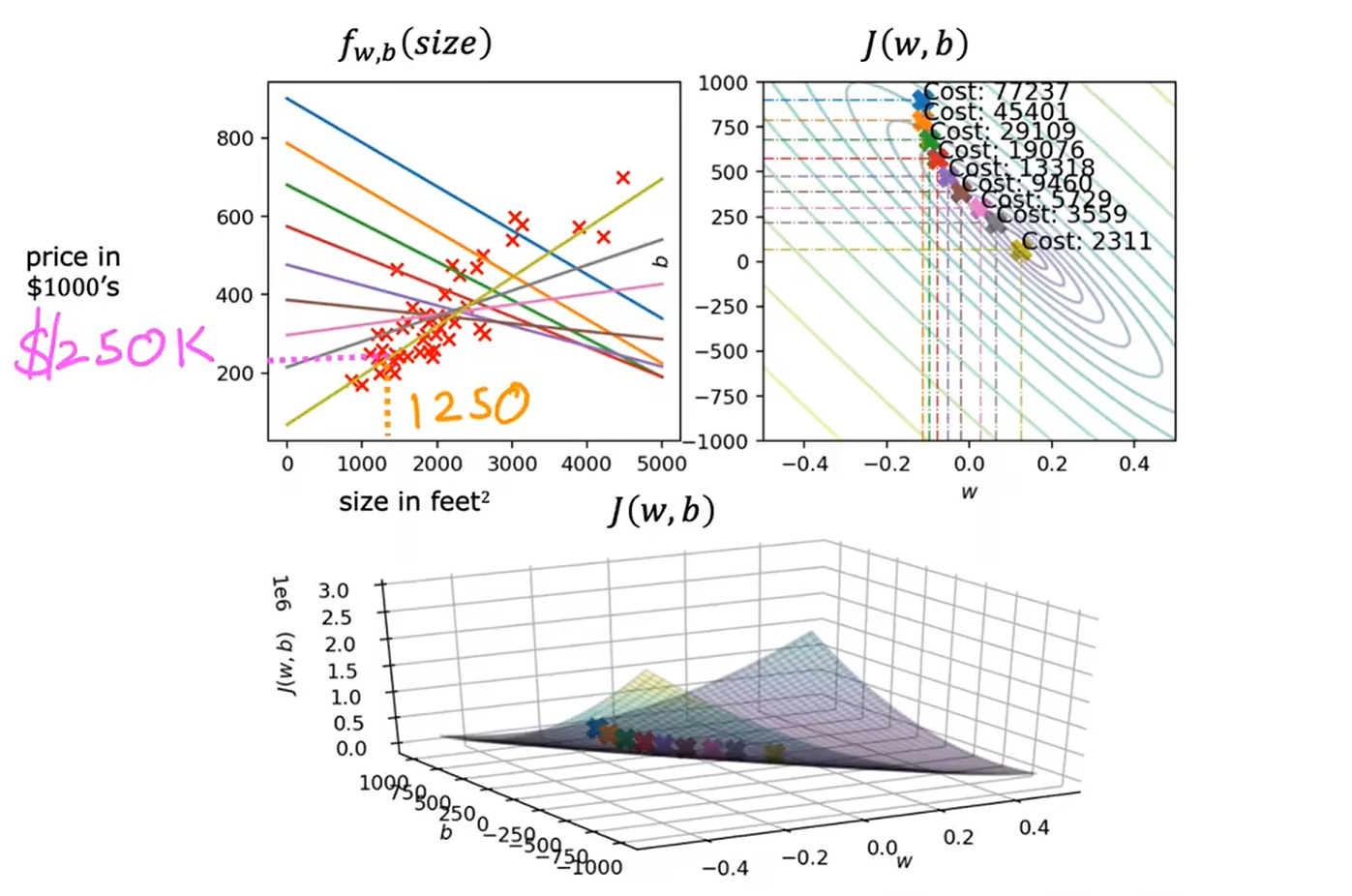## 多元线性回归

       x

j

:

第

j

列，即第

j

个特征

n

：特征的数量

x

⃗

(

i

)

:

第

i

个训练样本，即第

i

行数据

x

j

(

i

)

:

第

i

个训练样本的第

j

个特征的值

x_j : 第 j 列，即第 j 个特征 \\ n：特征的数量 \\ \vec{x}^{(i)} : 第 i 个训练样本，即第 i 行数据 \\ x^{(i)}_j : 第 i 个训练样本的 第 j 个特征的值 \\

xj​:第j列，即第j个特征n：特征的数量x(i):第i个训练样本，即第i行数据xj(i)​:第i个训练样本的第j个特征的值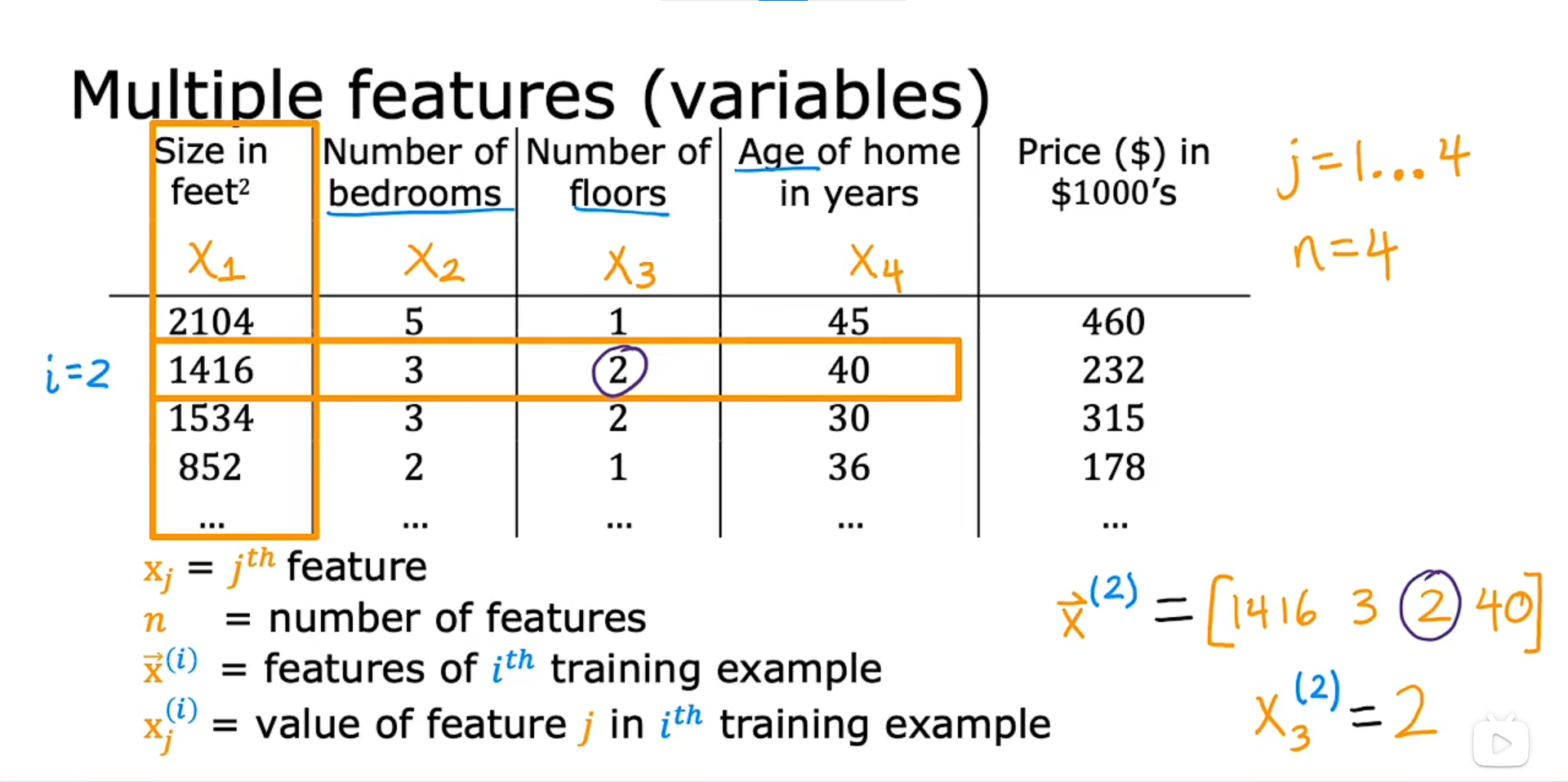M

o

d

e

l

:

Model:

Model:

P

r

e

v

i

o

u

s

l

y

:

f

w

,

b

(

x

)

=

w

x

+

b

Previously: f_{w, b}(x) = wx + b

Previously:fw,b​(x)=wx+b

f

w

,

b

(

x

)

=

w

1

x

1

+

w

2

x

2

+

w

3

x

3

+

w

4

x

4

+

b

f_{w, b}(x) = w_1x_1 + w_2x_2 + w_3x_3 + w_4x_4 + b

fw,b​(x)=w1​x1​+w2​x2​+w3​x3​+w4​x4​+b

e

x

a

m

p

l

e

:

f

w

,

b

(

x

)

=

0.1

x

1

+

4

x

2

+

10

x

3

+

(

−

2

)

x

4

+

80

example: f_{w,b}(x) = 0.1x_1 + 4x_2 + 10x_3 + (-2)x_4 + 80

example:fw,b​(x)=0.1x1​+4x2​+10x3​+(−2)x4​+80

x

1

:

s

i

z

e

,

x

2

:

b

e

d

r

o

o

m

s

,

x

3

:

f

l

o

o

r

s

,

x

4

:

y

e

a

r

s

,

80

:

b

a

s

e

p

r

i

c

e

x_1: size, x_2: bedrooms, x_3: floors, x_4: years, 80: base price

x1​:size,x2​:bedrooms,x3​:floors,x4​:years,80:baseprice

f

w

,

b

(

x

)

=

w

1

x

1

+

w

2

x

2

+

⋅

⋅

⋅

+

w

n

x

n

+

b

f_{w, b}(x) = w_1x_1 + w_2x_2 + ··· + w_nx_n + b

fw,b​(x)=w1​x1​+w2​x2​+⋅⋅⋅+wn​xn​+b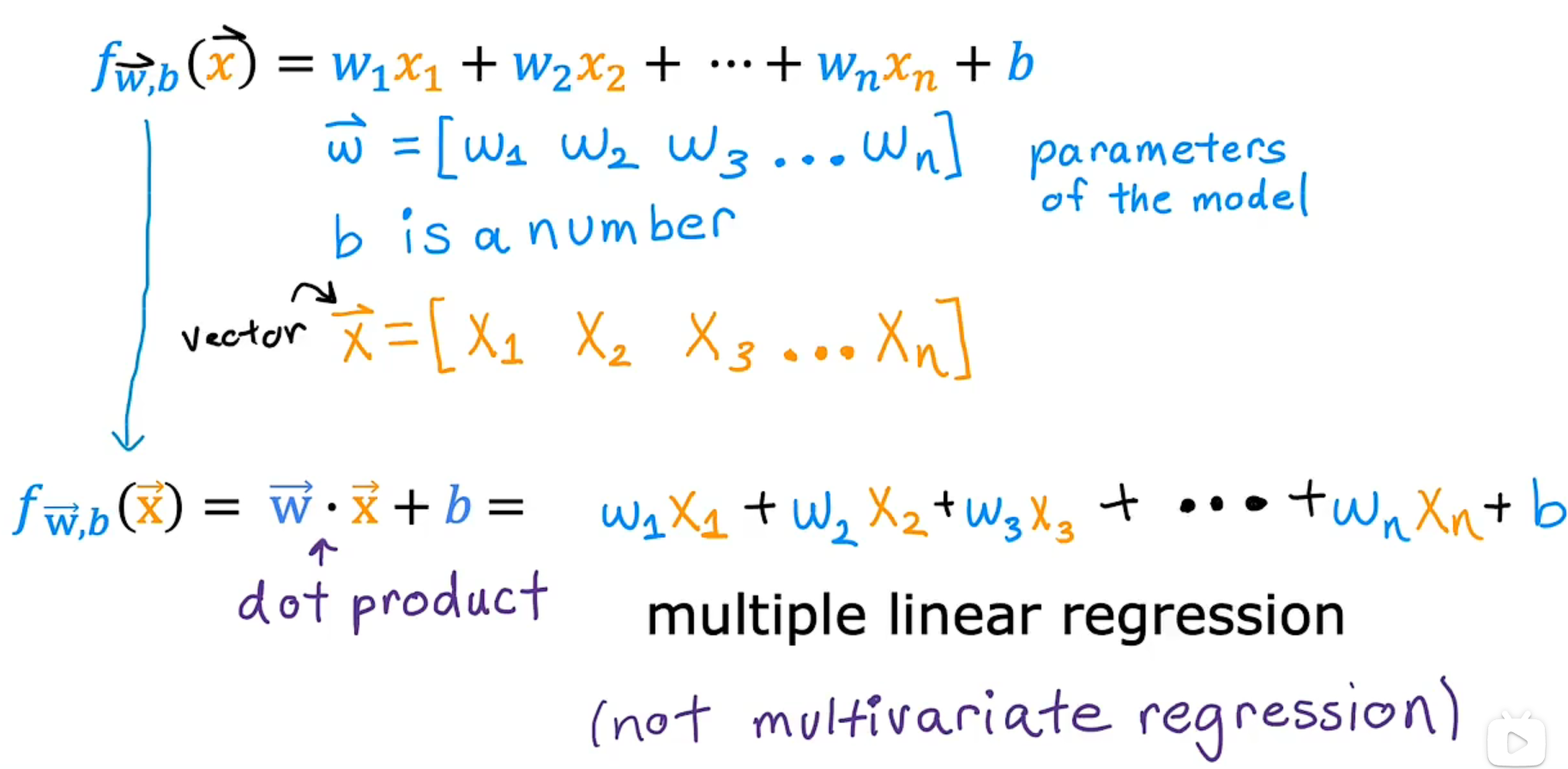## 向量化 Vectorization

Numpy：Python中的数值线性代数库

1. 代码简洁
2. 运行速度较快
np.dot(w, x)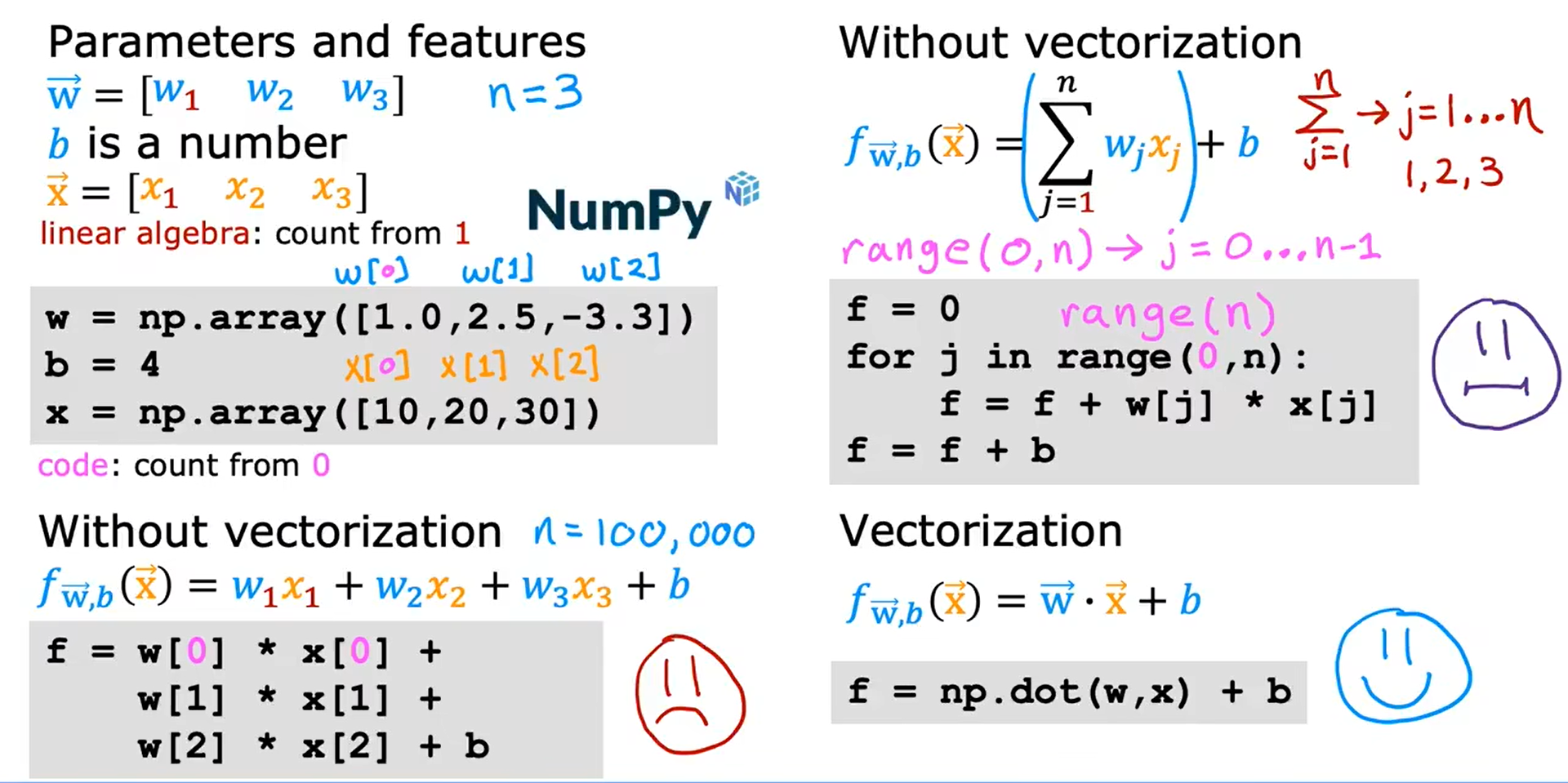★☆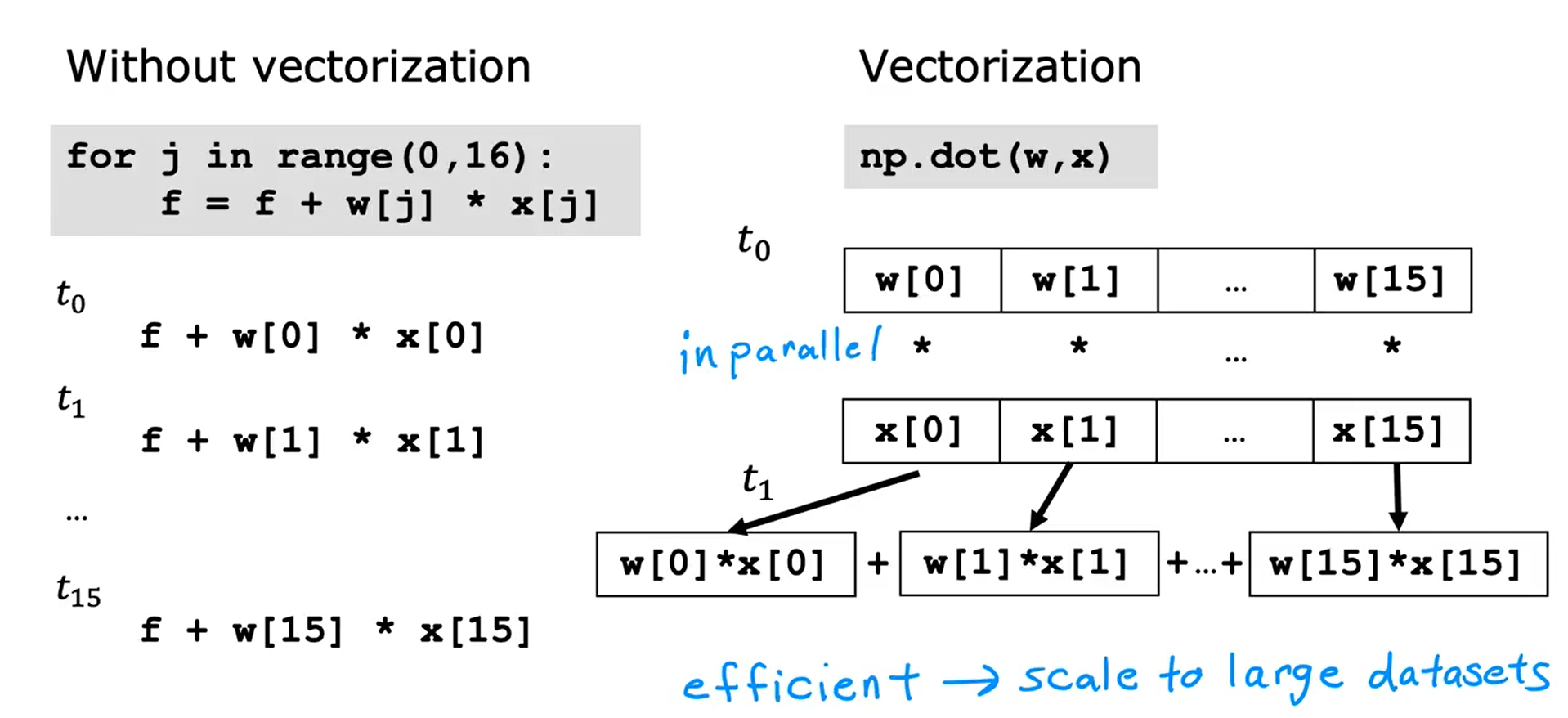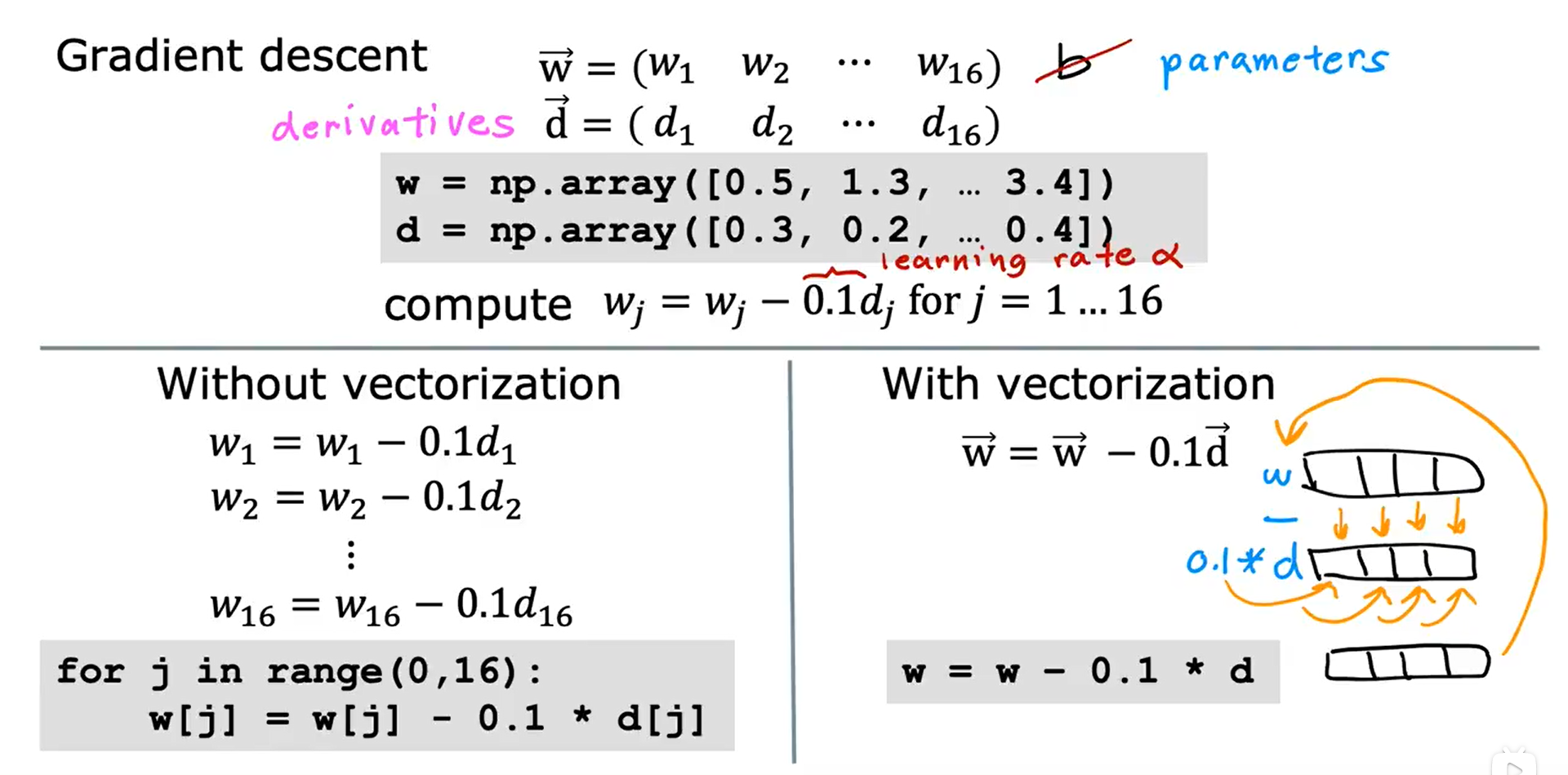## 多元线性回归的梯度下降法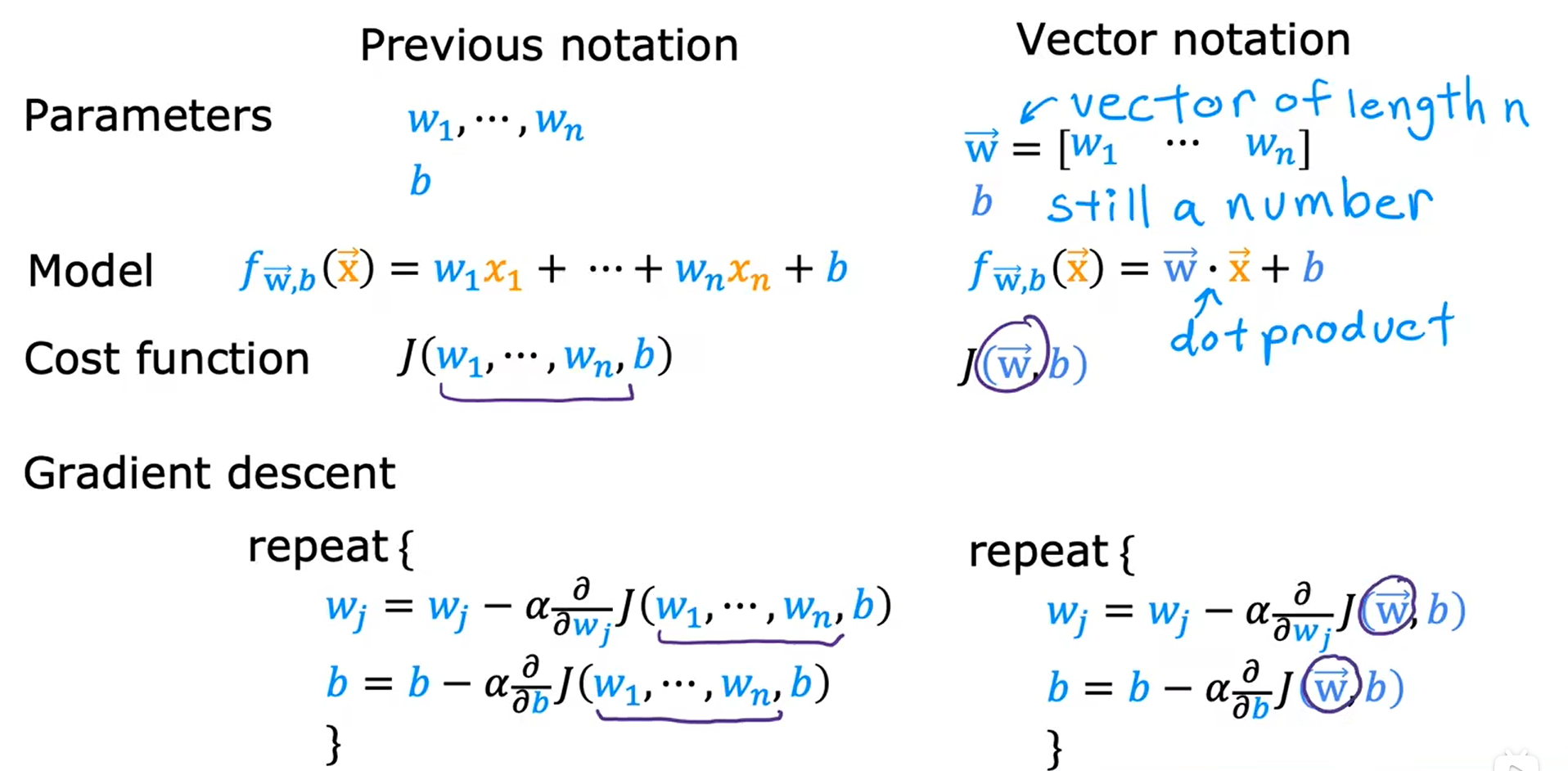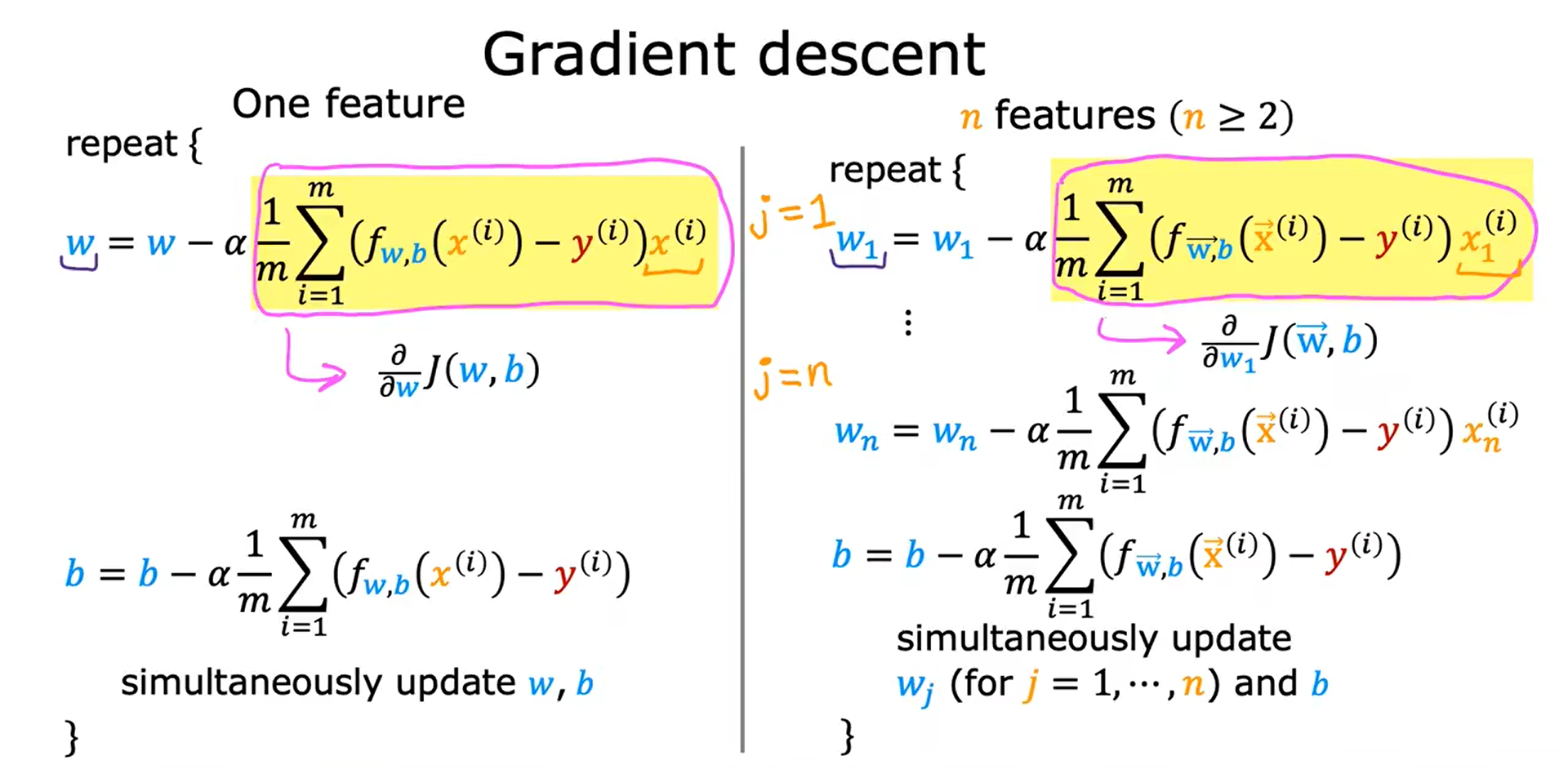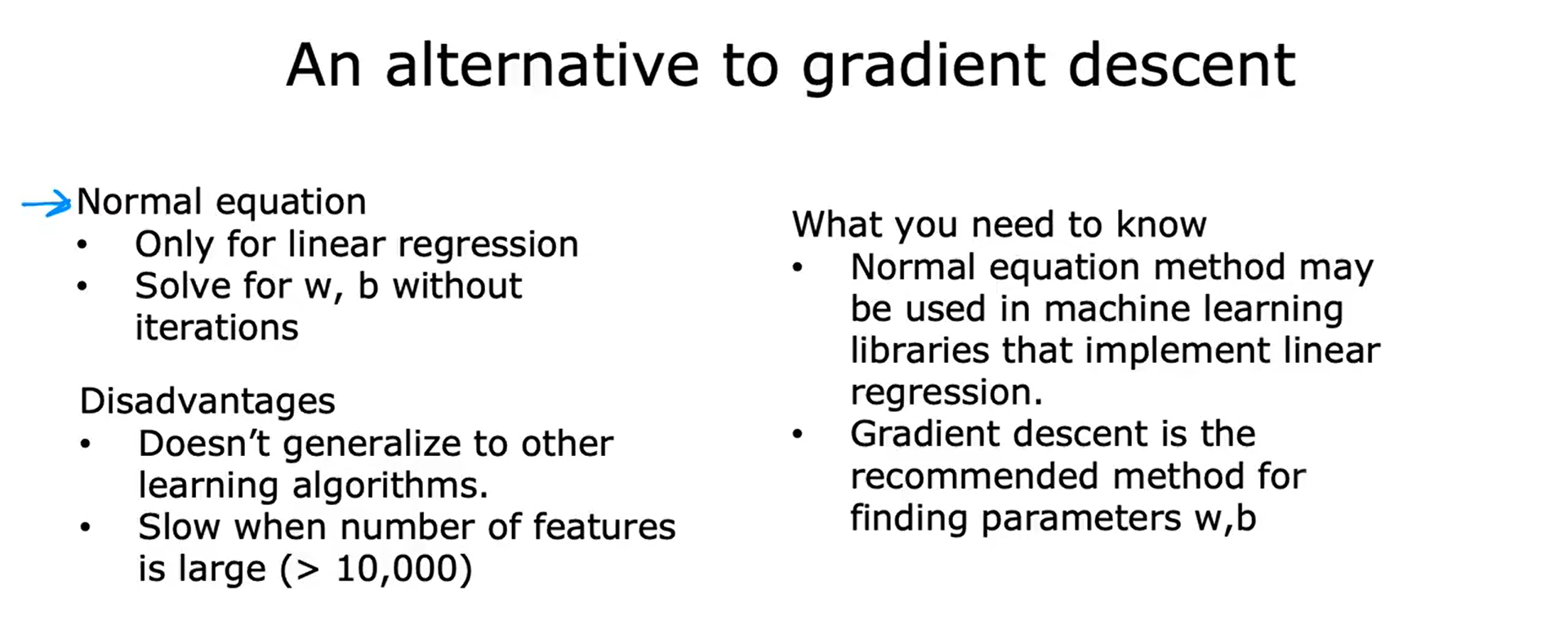## 特征放缩 Feature Scaling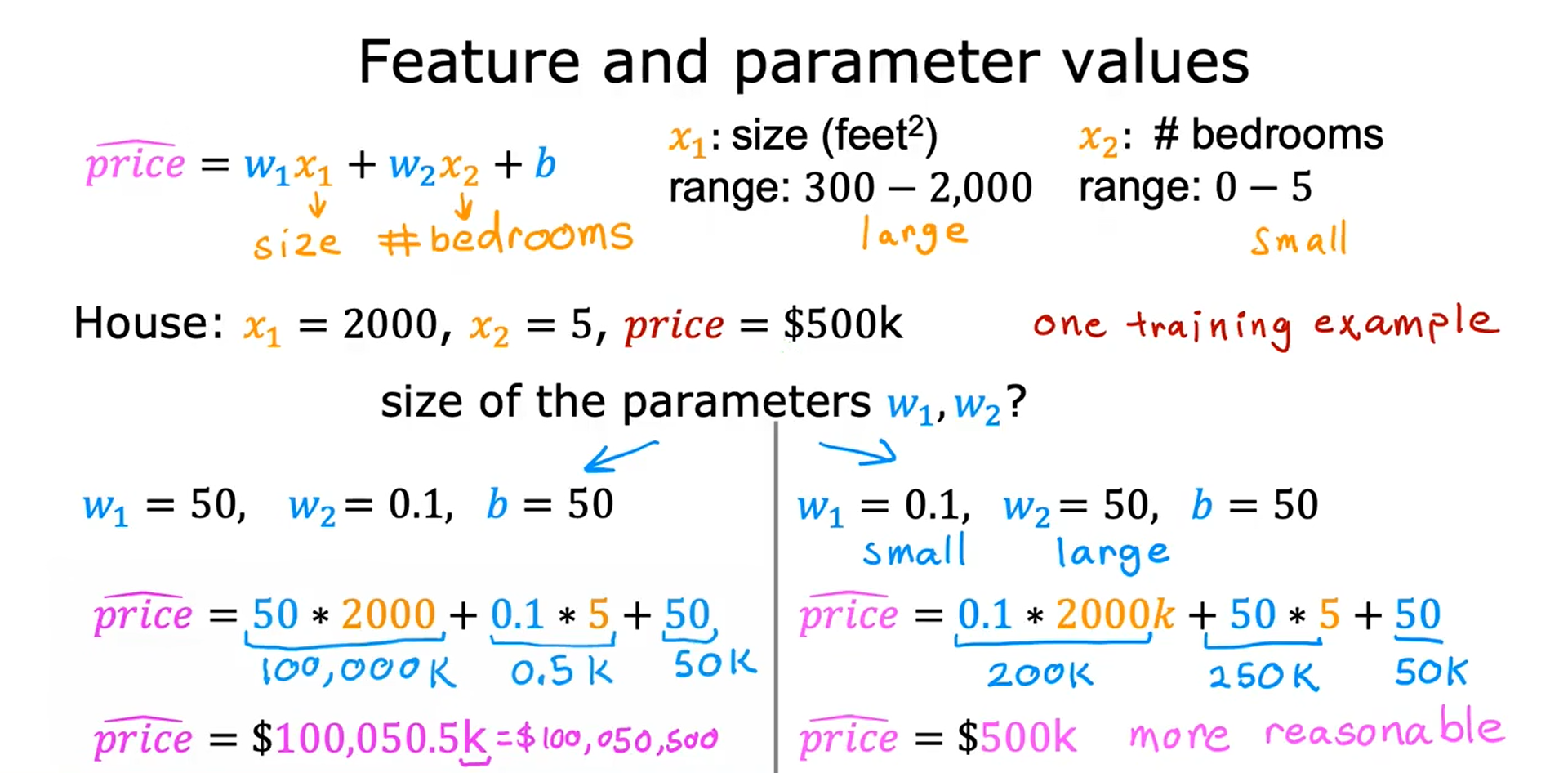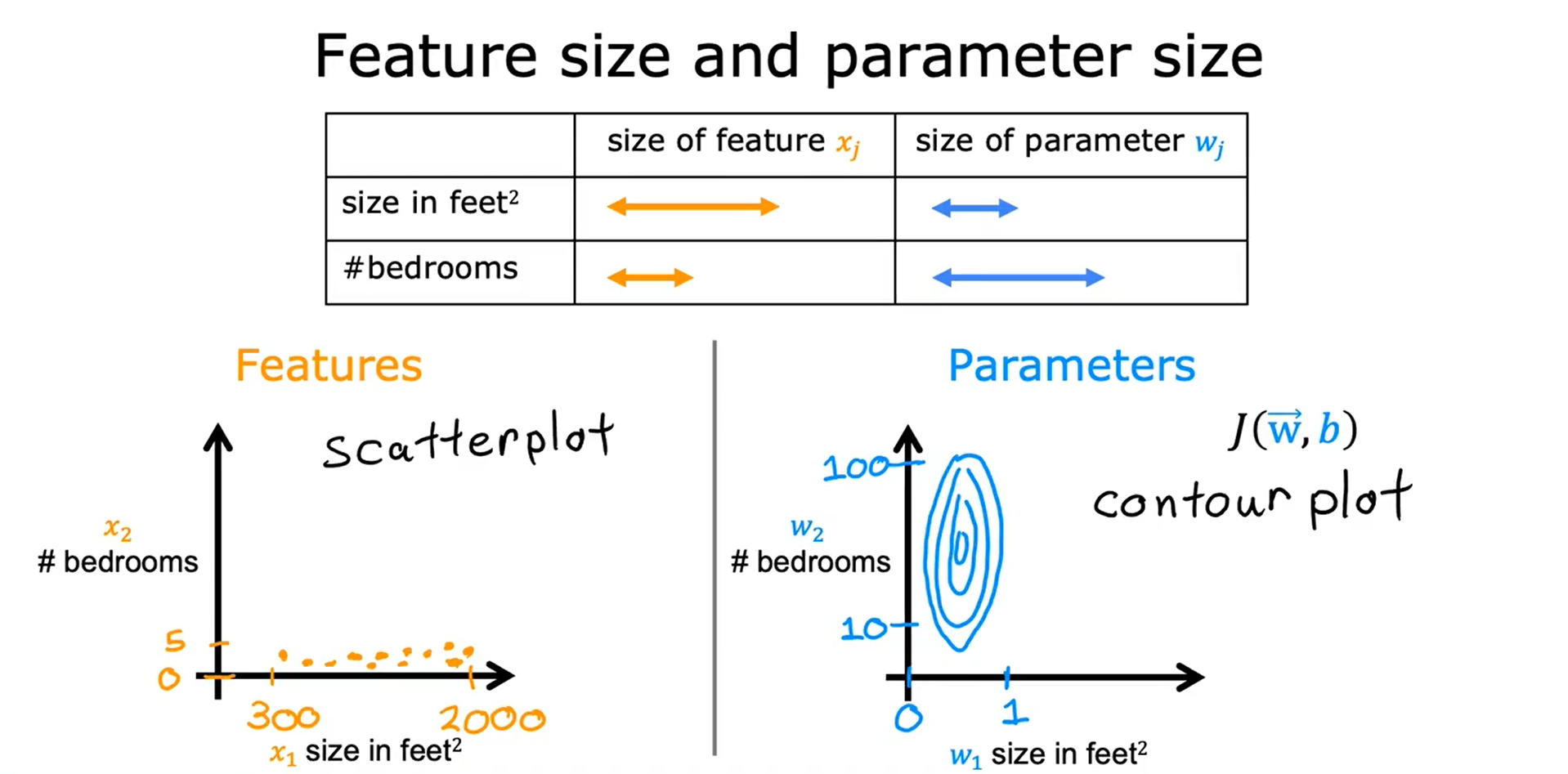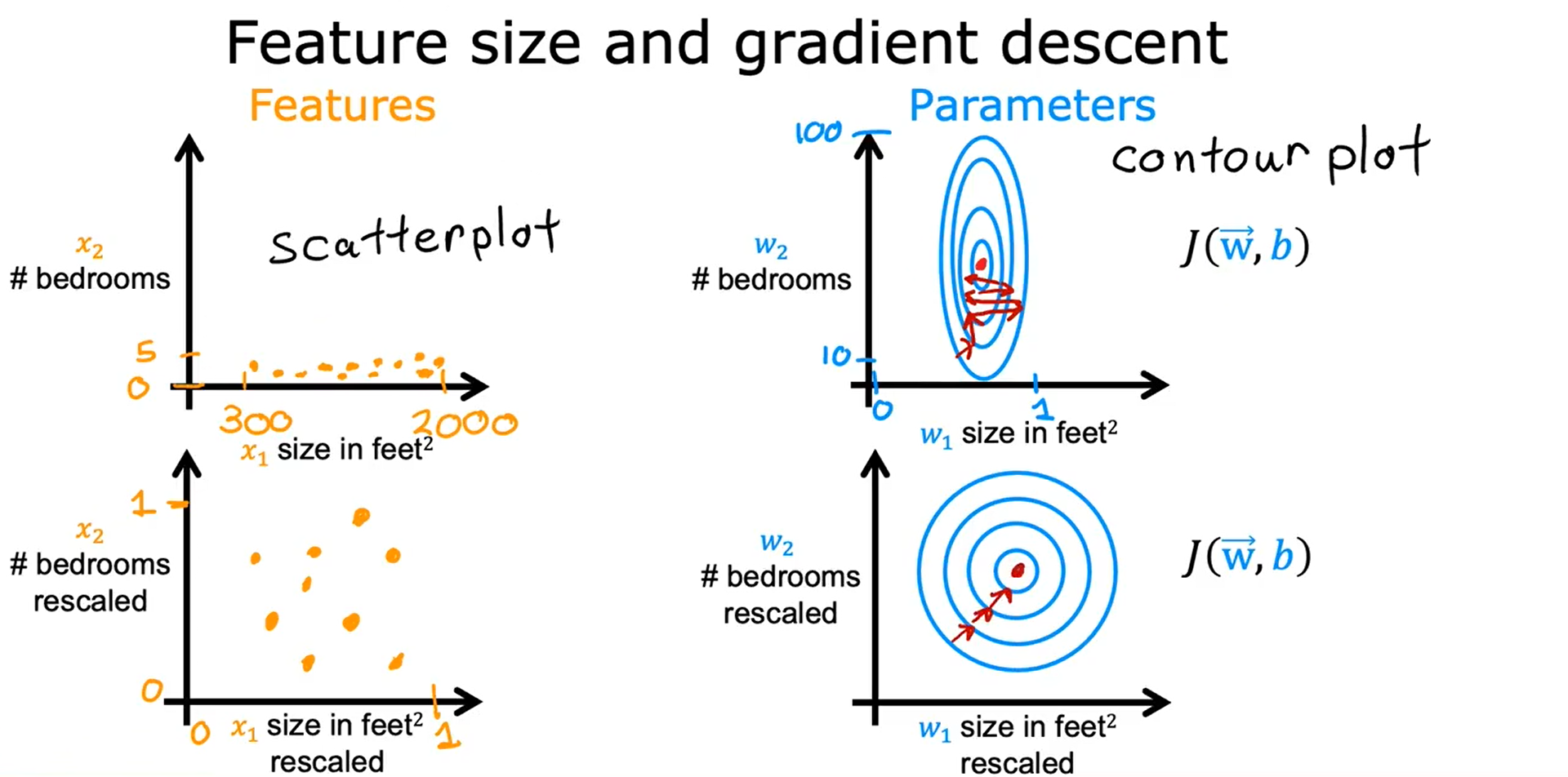## 1. 最大值标准化

x1的放缩范围：300/2000 ~ 2000/2000，即 0.15 ~ 1
x2的放缩范围：0/5 ~ 5/5，即 0 ~ 1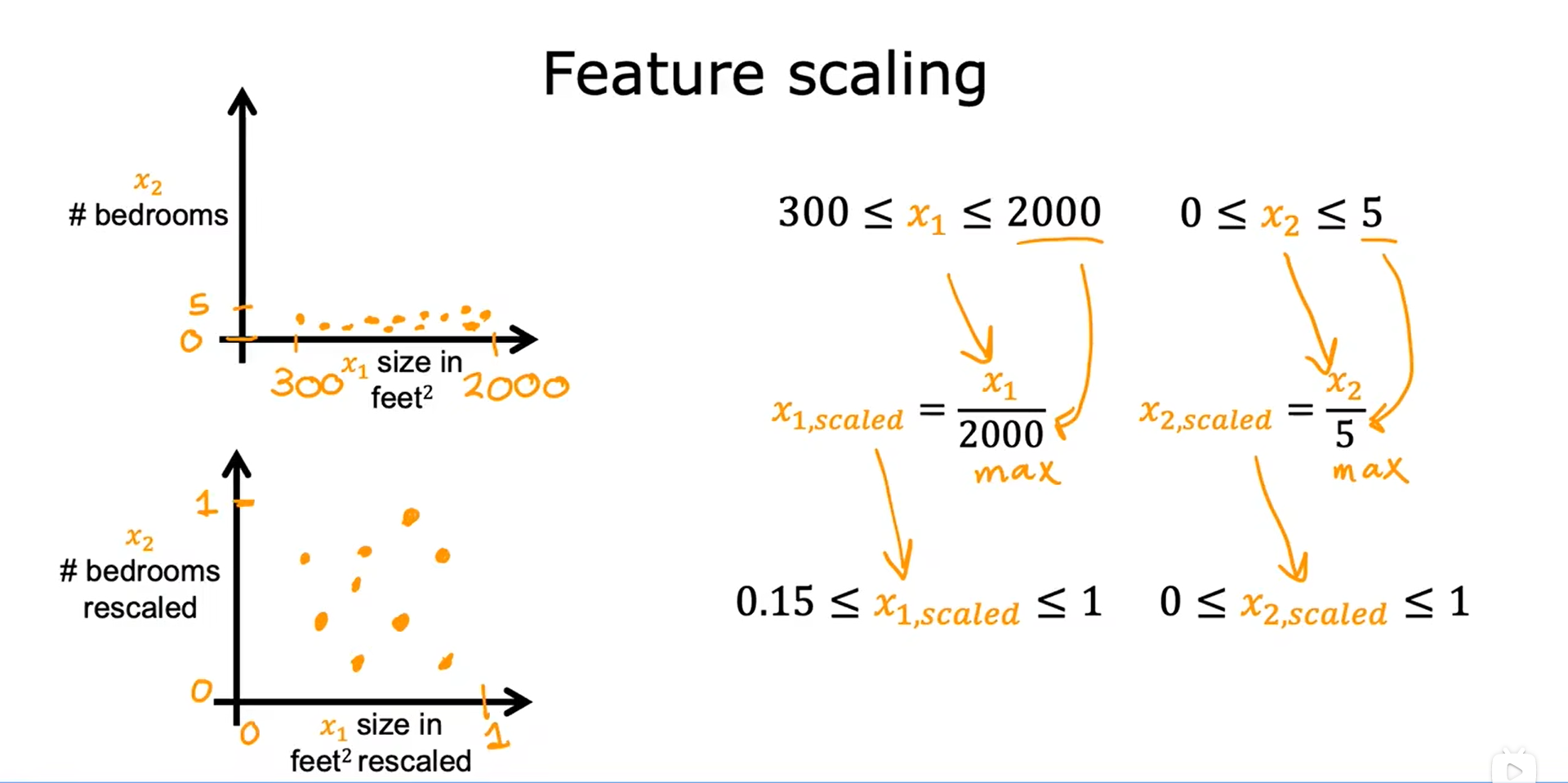## 2. 均值归一化 Mean Normalization

1. 找到训练集上的平均值 μ（此例中，μ1 = 600 作为 x1 的平均值）
2.                                                      x                                  1                                          =                                                                      x                                        1                                                  −                                                   μ                                        1                                                                        m                                     a                                     x                                     −                                     m                                     i                                     n                                                             x_1 = \frac{x_1 - \mu_1}{max - min}                        x1​=max−minx1​−μ1​​， 即                                                    x                               1                                      m                            i                            n                            =                                                   300                                  −                                  600                                                      2000                                  −                                  300                                                 =                            −                            0.18                                  x_1 min = \frac{300 - 600}{2000 - 300} = -0.18                     x1​min=2000−300300−600​=−0.18，                                                   x                               1                                      m                            a                            x                            =                                                   2000                                  −                                  600                                                      2000                                  −                                  300                                                 =                            0.82                                  x_1 max = \frac{2000 - 600}{2000 - 300} = 0.82                     x1​max=2000−3002000−600​=0.82，得到                                              −                               0.18                               ≤                                           x                                  1                                          ≤                               0.82                                      -0.18 \leq x_1 \leq 0.82                        −0.18≤x1​≤0.82，此时，x1 已均值归一化。

3. 找到训练集上的平均值 μ（此例中，μ2 = 2.3 作为 x2 的平均值）
4.                                                      x                                  2                                          =                                                                      x                                        2                                                  −                                                   μ                                        2                                                                        m                                     a                                     x                                     −                                     m                                     i                                     n                                                             x_2 = \frac{x_2 - \mu_2}{max - min}                        x2​=max−minx2​−μ2​​，即                                                    x                               2                                      m                            i                            n                            =                                                   0                                  −                                  2.3                                                      5                                  −                                  0                                                 =                            −                            0.46                                  x_2min = \frac{0 - 2.3}{5 - 0} = -0.46                     x2​min=5−00−2.3​=−0.46，                                                   x                               2                                      m                            a                            x                            =                                                   5                                  −                                  2.3                                                      5                                  −                                  0                                                 =                            0.54                                  x_2max = \frac{5 - 2.3}{5 - 0} = 0.54                     x2​max=5−05−2.3​=0.54，得到                                              −                               0.46                               ≤                                           x                                  2                                          ≤                               0.54                                      -0.46 \leq x_2 \leq 0.54                        −0.46≤x2​≤0.54，此时，x2 已均值归一化。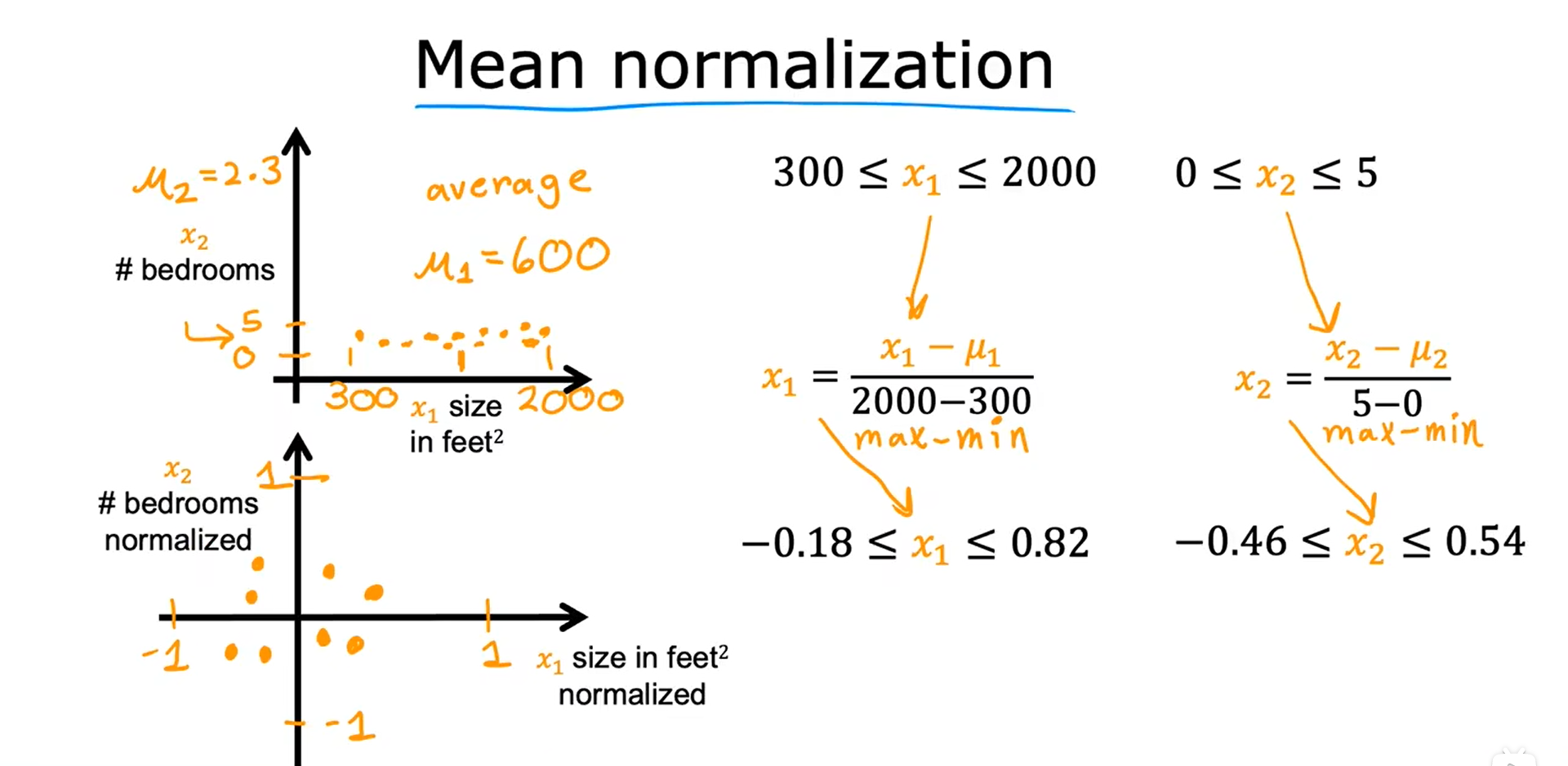## 3. Z分数归一化 Z-score normalization

1. 若 x1 的标准差为 σ1 = 450，平均值 μ1 = 600
2.                                                      x                                  1                                          =                                                                      x                                        1                                                  −                                                   μ                                        1                                                                        σ                                     1                                                             x_1 = \frac{x_1 - \mu_1}{\sigma_1}                        x1​=σ1​x1​−μ1​​,                                                    x                               1                                      m                            i                            n                            =                                                   300                                  −                                  600                                          450                                      =                            0.67                            ,                                       x                               2                                      m                            a                            x                            =                                                   2000                                  −                                  600                                          450                                      =                            3.1                                  x_1min = \frac{300 - 600}{450} = 0.67, x_2max = \frac{2000 - 600}{450} = 3.1                     x1​min=450300−600​=0.67,x2​max=4502000−600​=3.1，即                                              −                               0.67                               ≤                                           x                                  1                                          ≤                               3.1                                      -0.67 \leq x_1 \leq 3.1                        −0.67≤x1​≤3.1

3. 若 x2 的标准差为 σ2 = 1.4，平均值 μ2 = 2.3
4.                                                      x                                  2                                          =                                                                      x                                        2                                                  −                                                   μ                                        2                                                                        σ                                     2                                                             x_2 = \frac{x_2 - \mu_2}{\sigma_2}                        x2​=σ2​x2​−μ2​​ ,                                                    x                               2                                      m                            i                            n                            =                                                   0                                  −                                  2.3                                          1.4                                      =                            −                            1.6                            ,                                       x                               2                                      m                            a                            x                            =                                                   5                                  −                                  2.3                                          1.4                                      =                            1.9                                  x_2min = \frac{0 - 2.3}{1.4} = -1.6, x_2max = \frac{5 - 2.3}{1.4} = 1.9                     x2​min=1.40−2.3​=−1.6,x2​max=1.45−2.3​=1.9，即                                              −                               1.6                               ≤                                           x                                  2                                          ≤                               1.9                                      -1.6 \leq x_2 \leq 1.9                        −1.6≤x2​≤1.9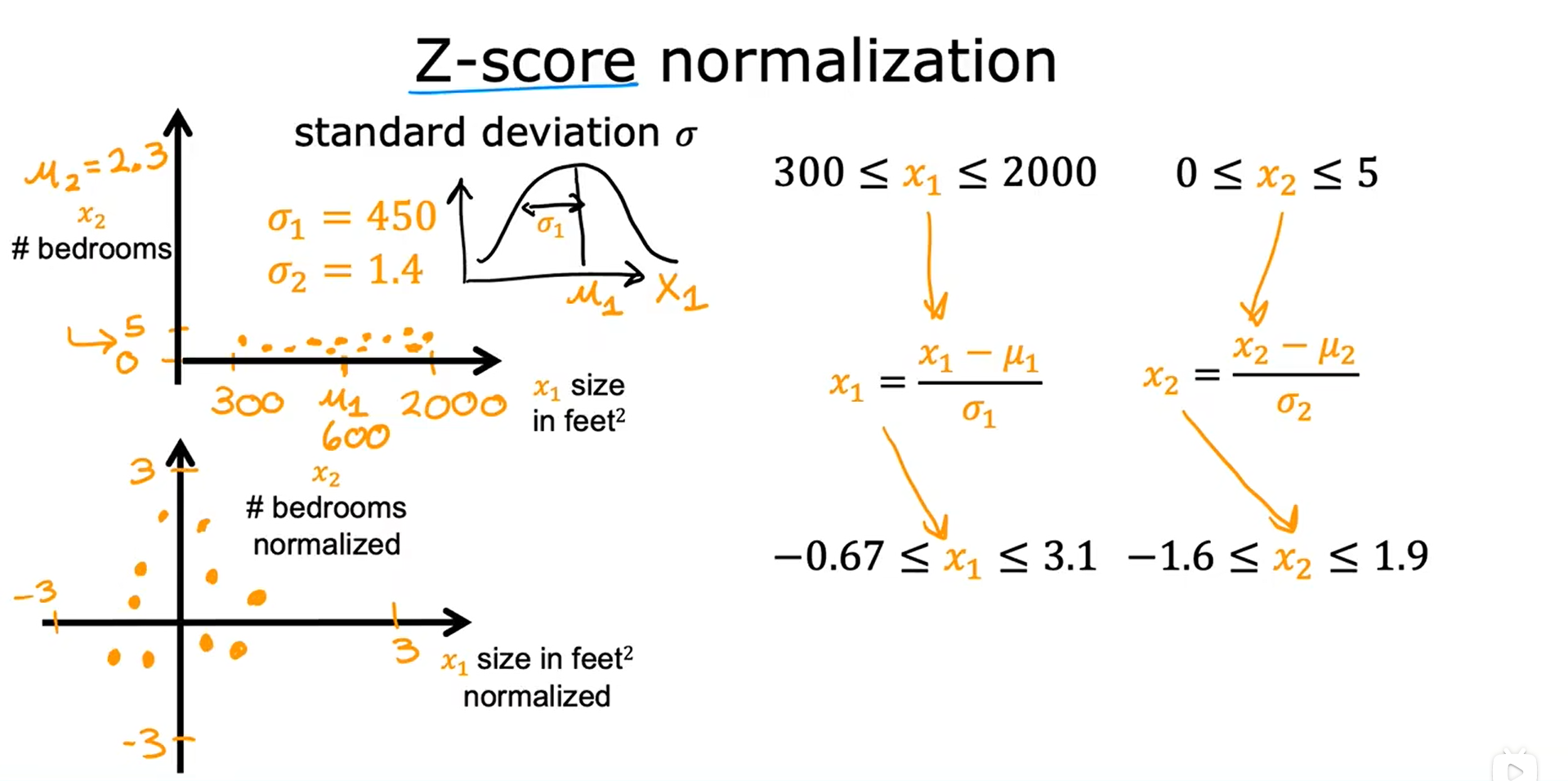## 检查梯度下降是否收敛

### 1. 创建一个 学习曲线 Learning Curve，试图推测出何时可以停止自己的特定的训练模型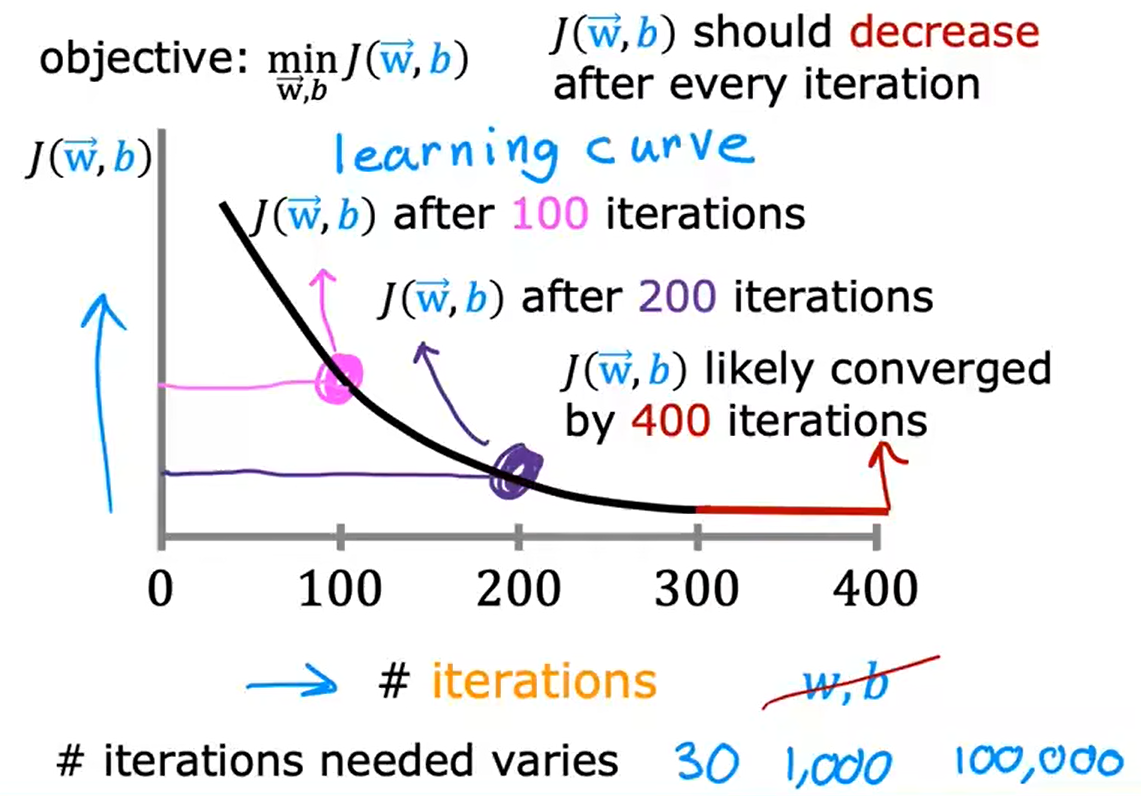### 2. 自动收敛测试 Automatic convergence test

1. 用 ϵ \epsilon ϵ 来代表一个很小的数字，比如 0.001
2. 如果在一次迭代中，成本函数的值下降的幅度小于 ϵ \epsilon ϵ ，那么现在的曲线图像就很可能处于上图中的平坦部分，此时即可宣布收敛，收敛表明此时找到的参数 向量w 和 常数b 很接近成本函数的最小值
3. 但通常情况下，选择正确的阈值 ϵ \epsilon ϵ 是相当困难的，所以实际上倾向于使用上面的方法，而不是使用自动收敛检测

## 学习率的选择

• 如果学习率过小，则梯度下降需要花费很多次的迭代才能收敛
• 如果学习率过大，则迭代的时候可能超过最小值，导致成本上升

## 特征工程 Feature Engineering

Using intuition to design new features, by transforming or combining original features.

       f

w

⃗

,

b

(

x

⃗

)

=

w

1

∗

x

1

+

w

2

∗

x

2

+

b

i

f

:

a

r

e

a

=

f

r

o

n

t

a

g

e

∗

d

e

p

t

h

s

e

t

:

x

3

=

x

1

∗

x

2

,

(

n

e

w

f

e

a

t

u

r

e

:

x

3

)

f

w

⃗

,

b

(

x

⃗

)

=

w

1

∗

x

1

+

w

2

∗

x

2

+

w

3

∗

x

3

+

b

f_{\vec{w}, b}(\vec{x}) = w_1 * x_1 + w_2 * x_2 + b \\ if: area = frontage * depth \\ set: x_3 = x_1 * x_2, (new feature: x_3)\\ f_{\vec{w},b}(\vec{x}) = w_1 * x_1 + w_2 * x_2 + w_3 * x_3 + b

fw,b​(x)=w1​∗x1​+w2​∗x2​+bif:area=frontage∗depthset:x3​=x1​∗x2​,(newfeature:x3​)fw,b​(x)=w1​∗x1​+w2​∗x2​+w3​∗x3​+b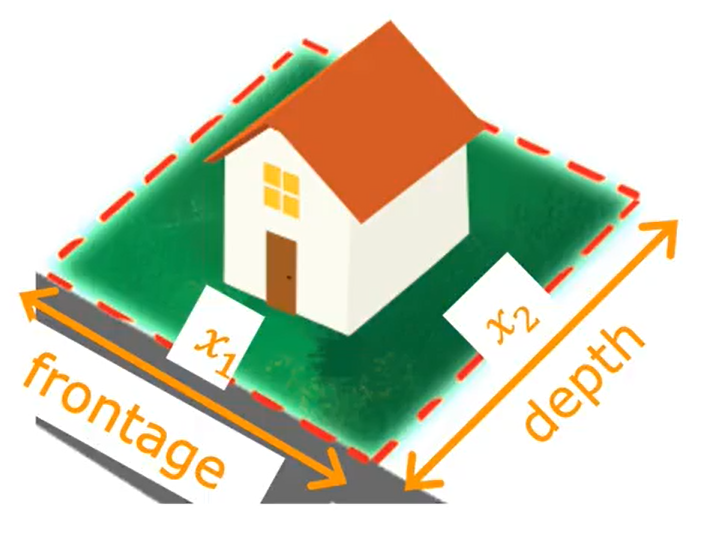## 多项式回归 Polynomial Regression

• 研究一个因变量与一个或多个自变量间多项式的回归分析方法，称为多项式回归（Polynomial Regression）。
• 如果自变量只有一个时，称为一元多项式回归；如果自变量有多个时，称为多元多项式回归。
• 在一元回归分析中，如果变量y与自变量x的关系为非线性的，但是又找不到适当的函数曲线来拟合，则可以采用一元多项式回归。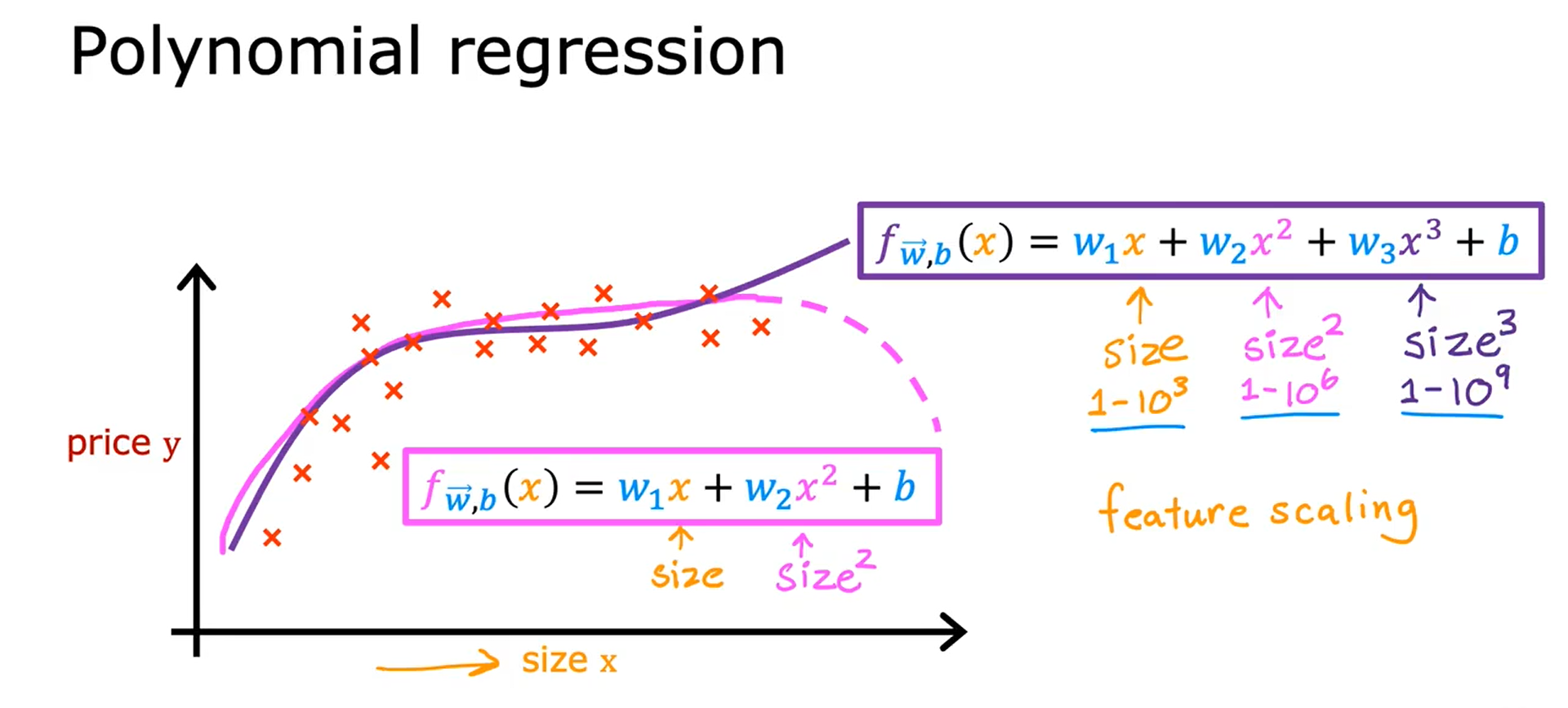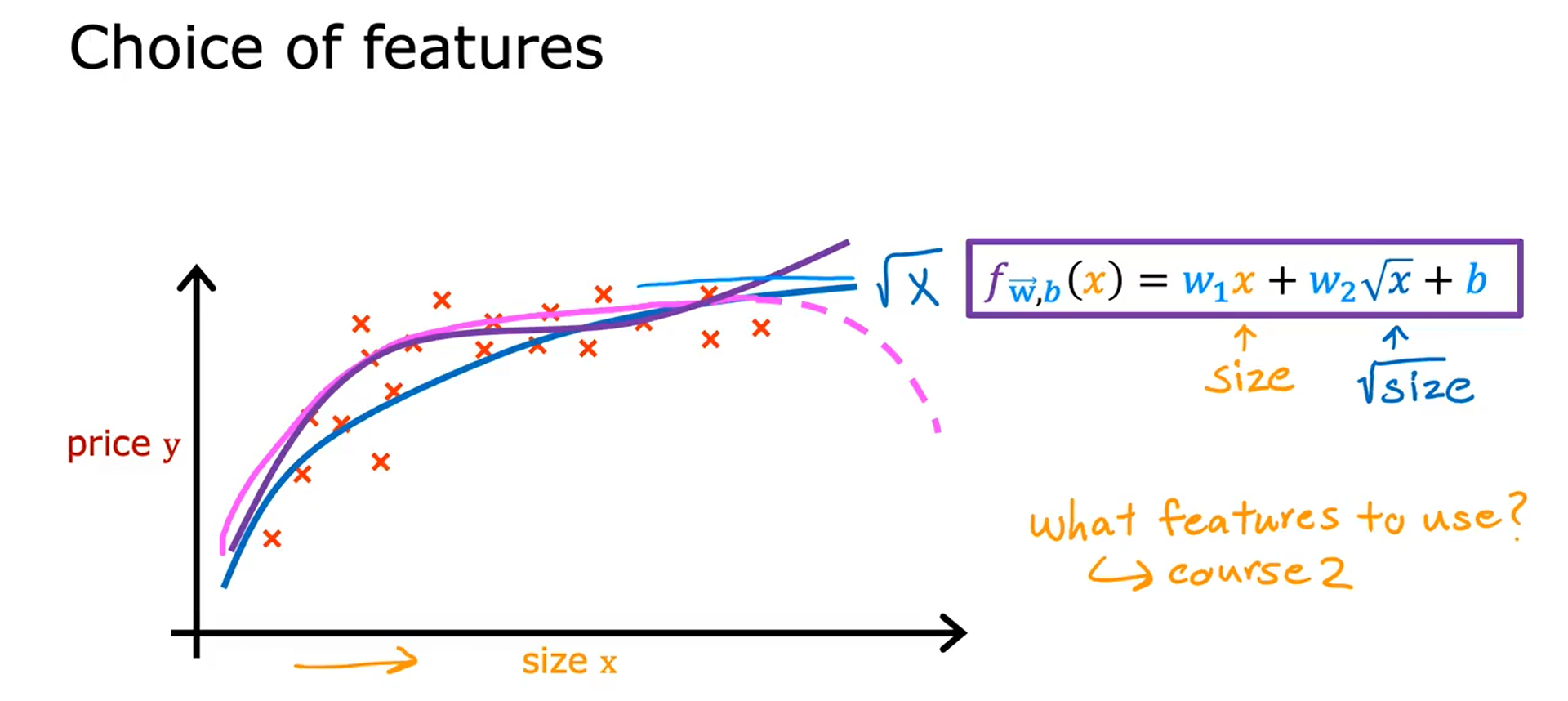## 动机 Motivation

QuestionAnswer “y”Is this email spam?no yesIs the transaction fraudulent?no yesIs the tumor malignant?no yes
Answer “y” can only be one of two values, no or yes, false or true, 0 or 1

class = category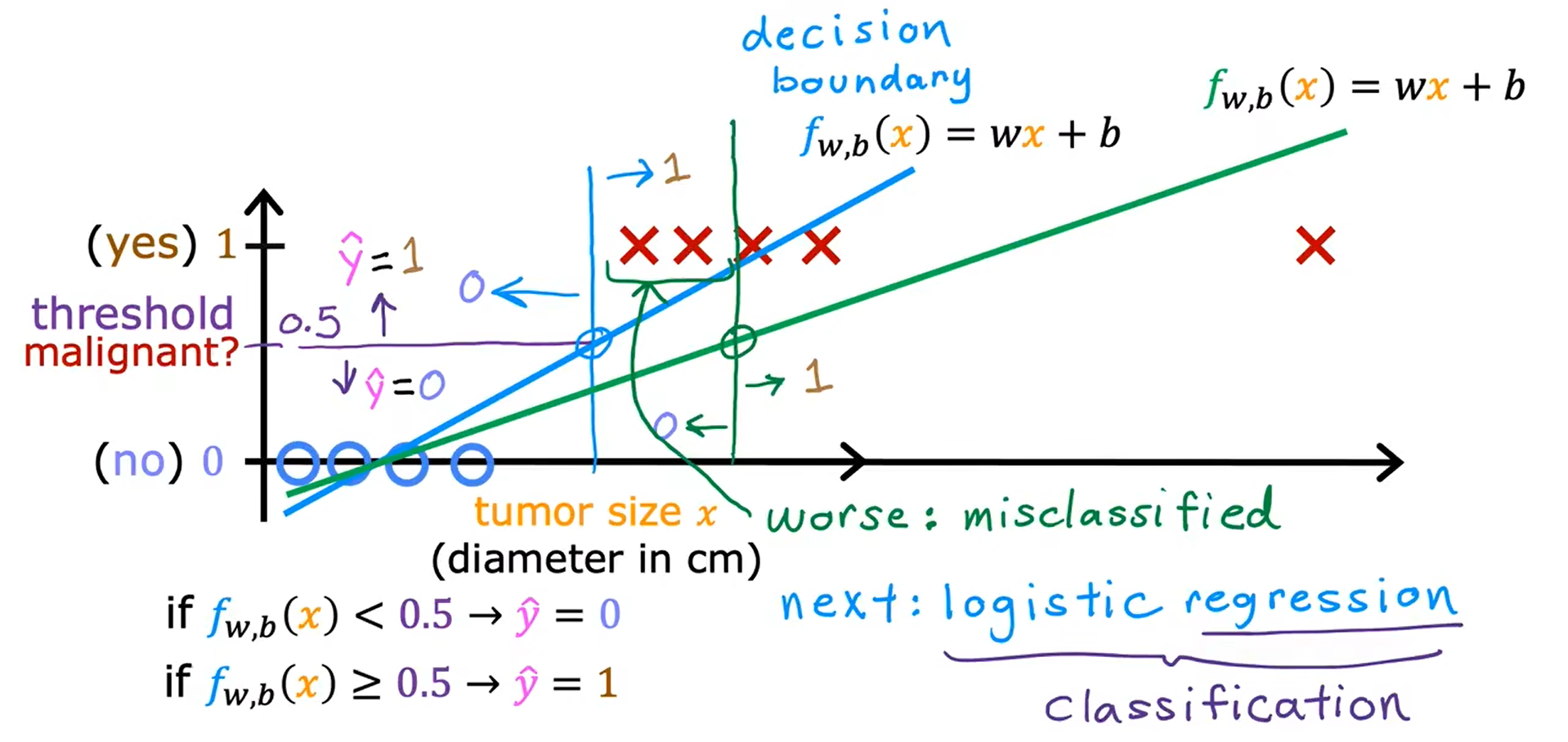## 逻辑回归 Logistic Regression

Sigmoid Function（Logistic Function）（乙状结肠函数，后勤函数，S型函数

     g

(

z

)

=

1

1

+

e

−

z

,

0

<

g

(

z

)

<

1

g(z) = \frac{1}{1 + e^{-z}}, 0 < g(z) < 1

g(z)=1+e−z1​,0<g(z)<1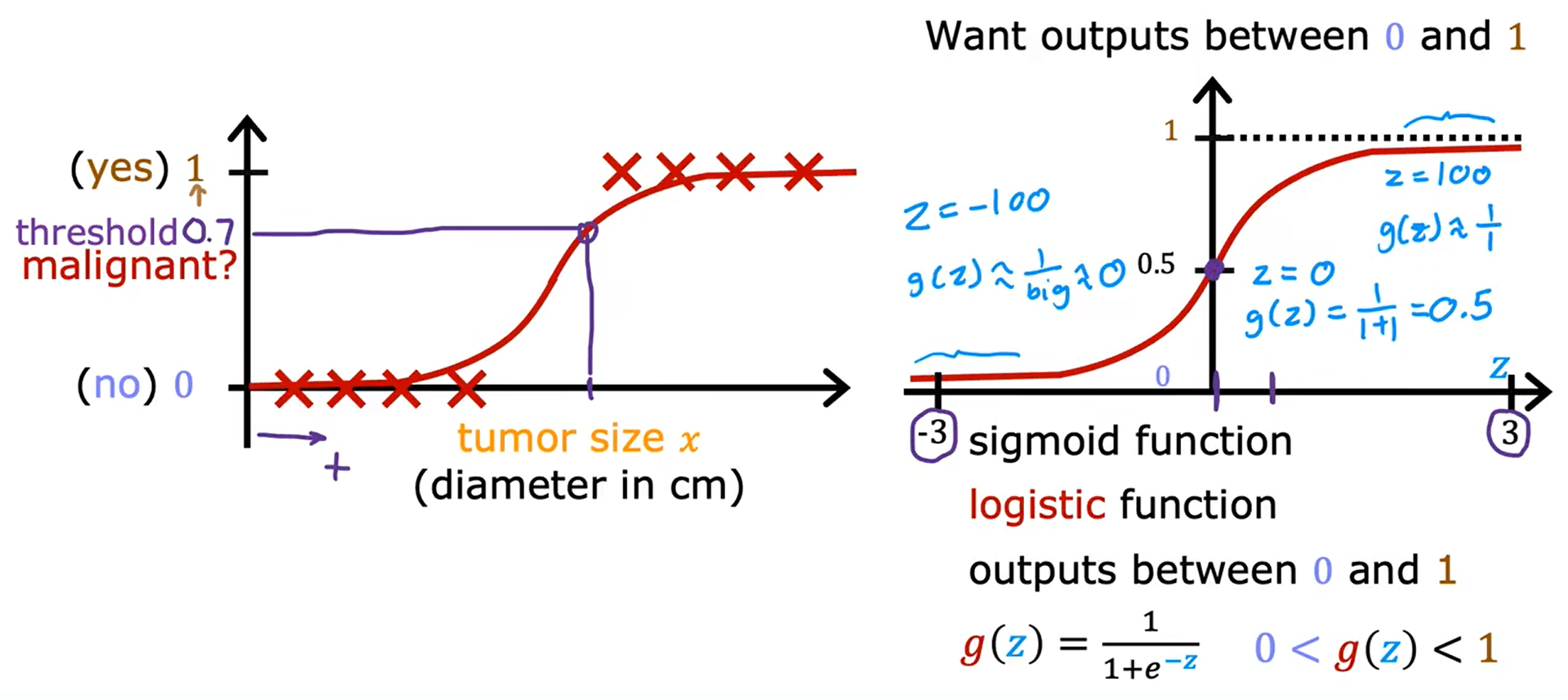f

w

⃗

,

b

(

x

⃗

)

=

g

(

w

⃗

⋅

x

⃗

+

b

)

=

1

1

+

e

−

(

w

⃗

⋅

x

⃗

+

b

)

f_{\vec{w},b}(\vec{x}) = g(\vec{w} · \vec{x} + b) = \frac{1}{1 + e^{-(\vec{w} · \vec{x} + b)}}

fw,b​(x)=g(w⋅x+b)=1+e−(w⋅x+b)1​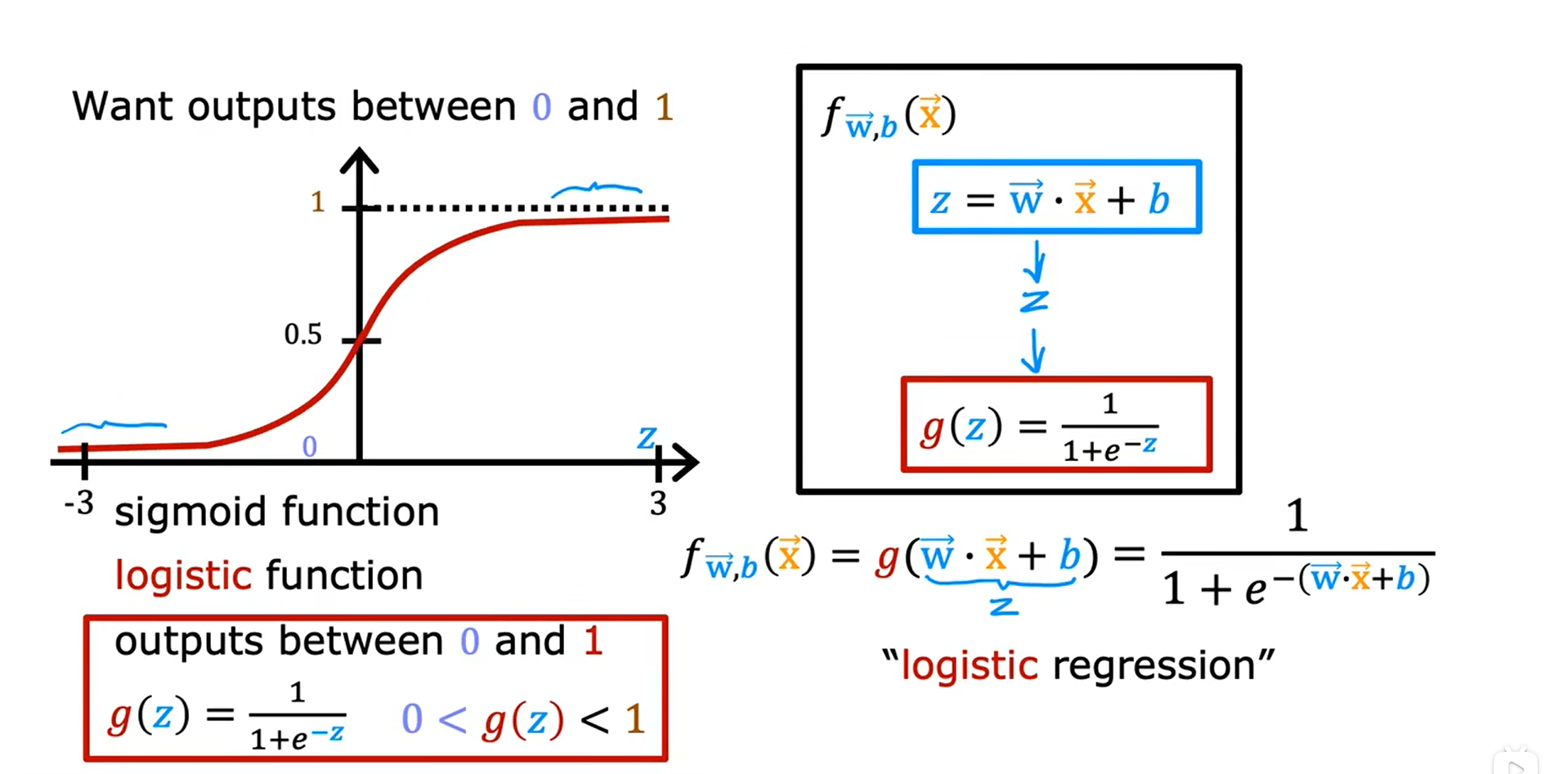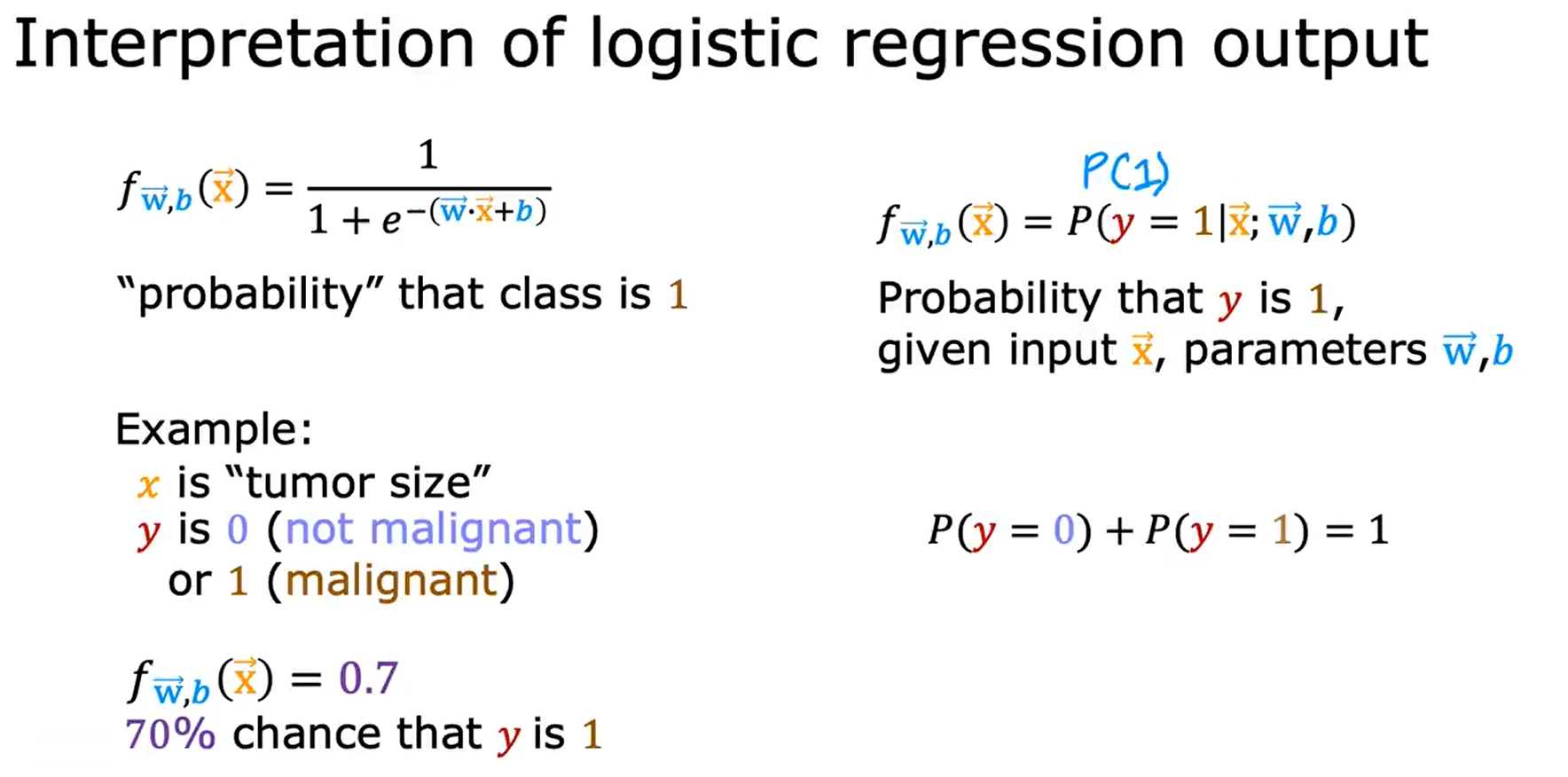## 决策边界 Decision Boundary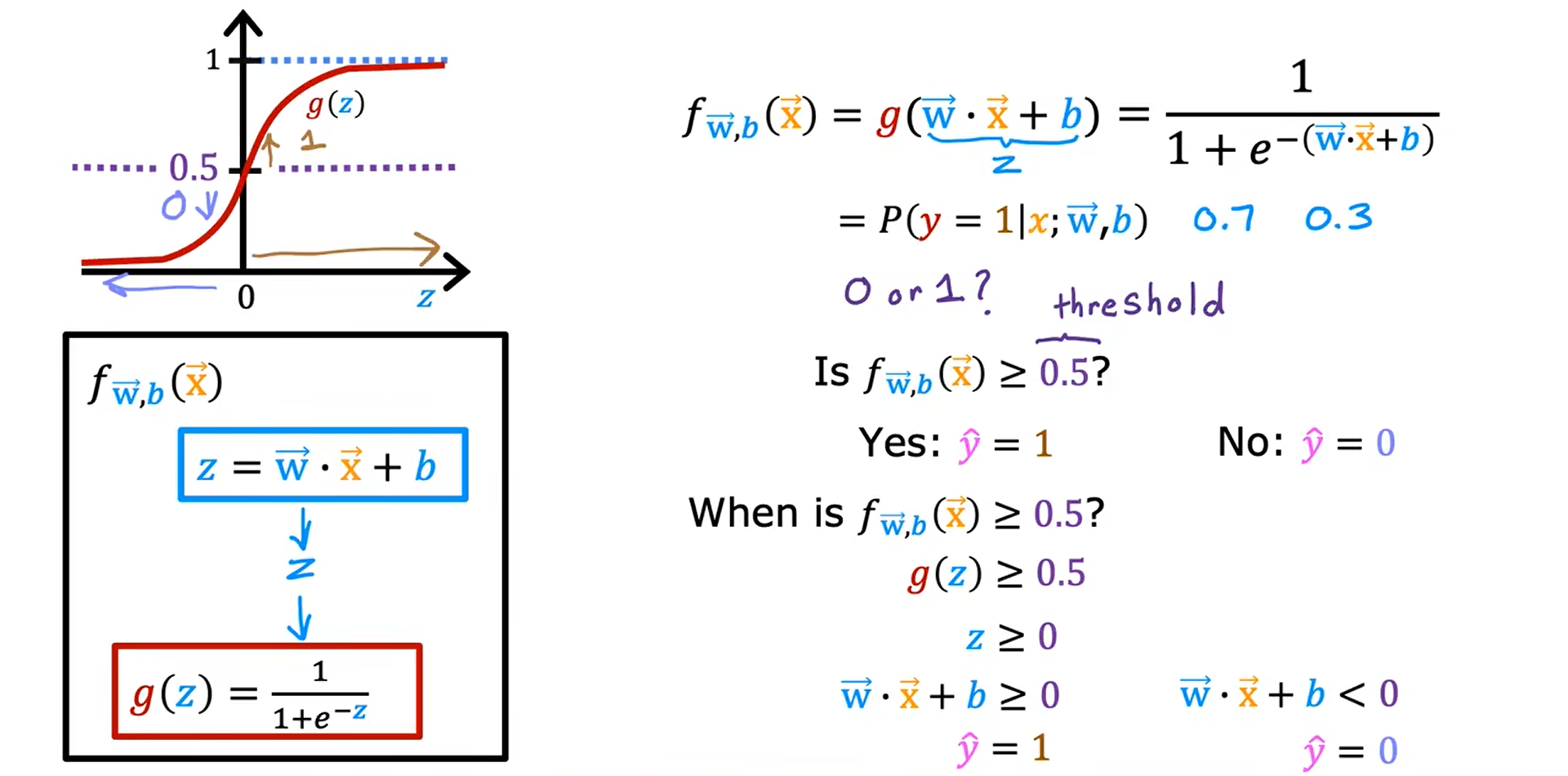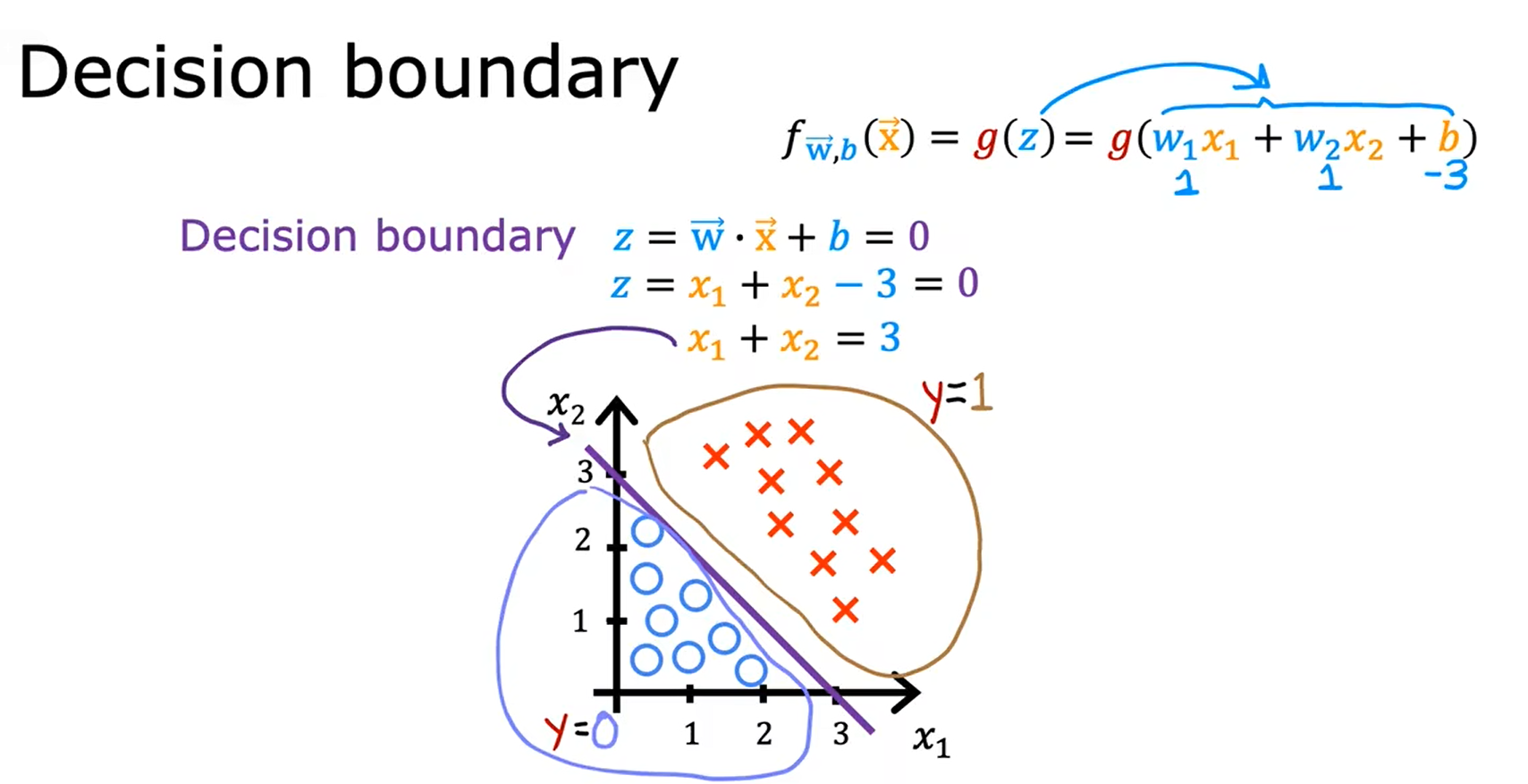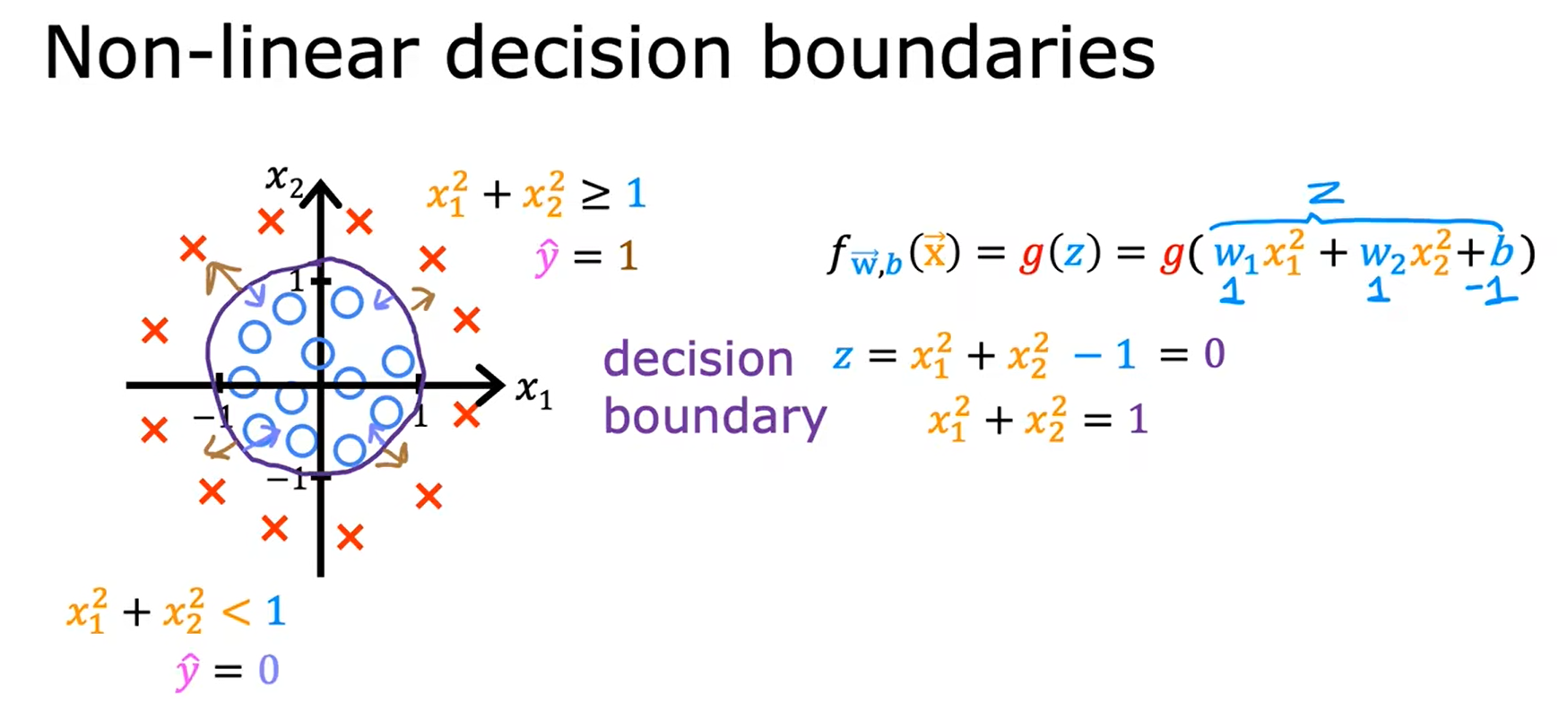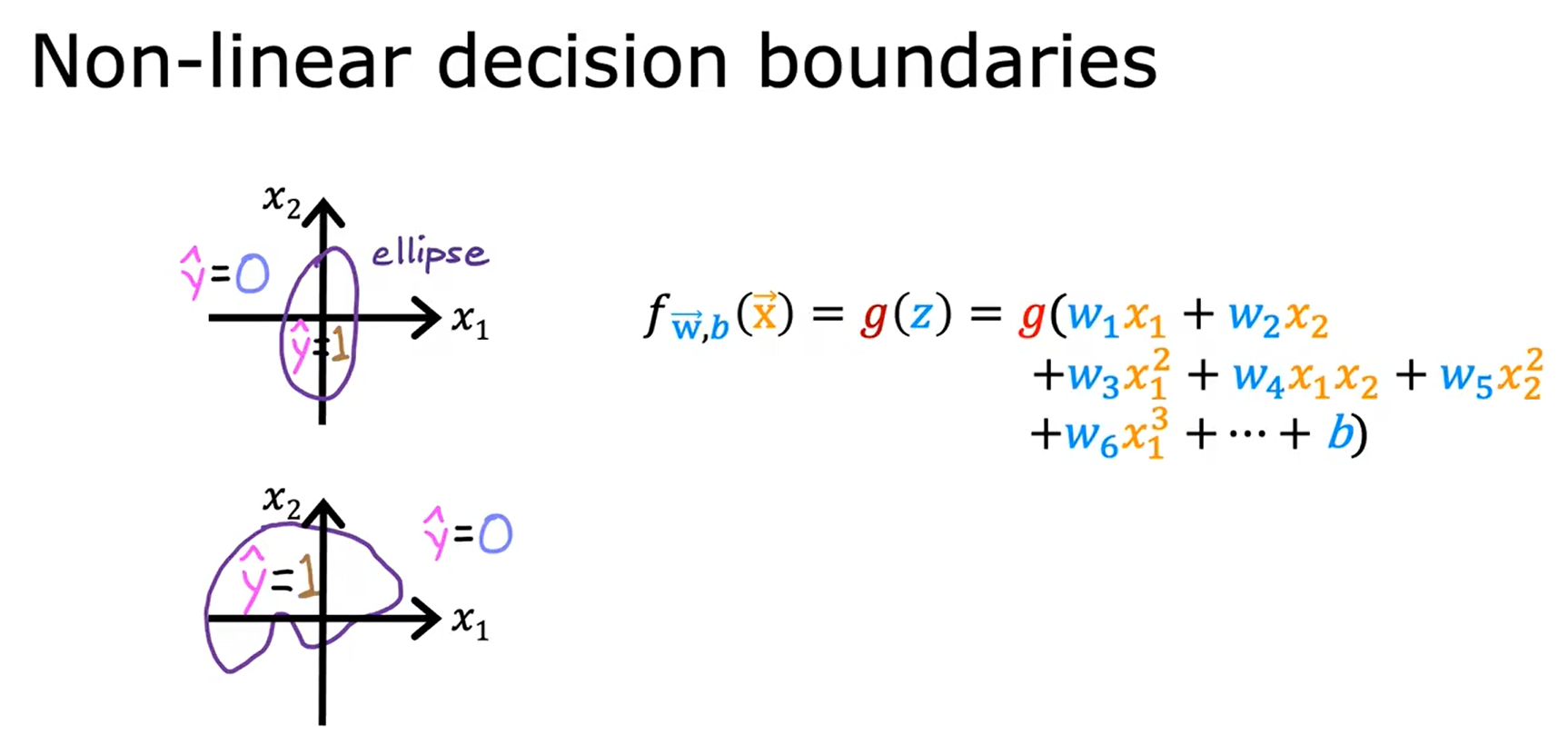## 逻辑回归的成本函数函数 Cost Function For Logistic Regression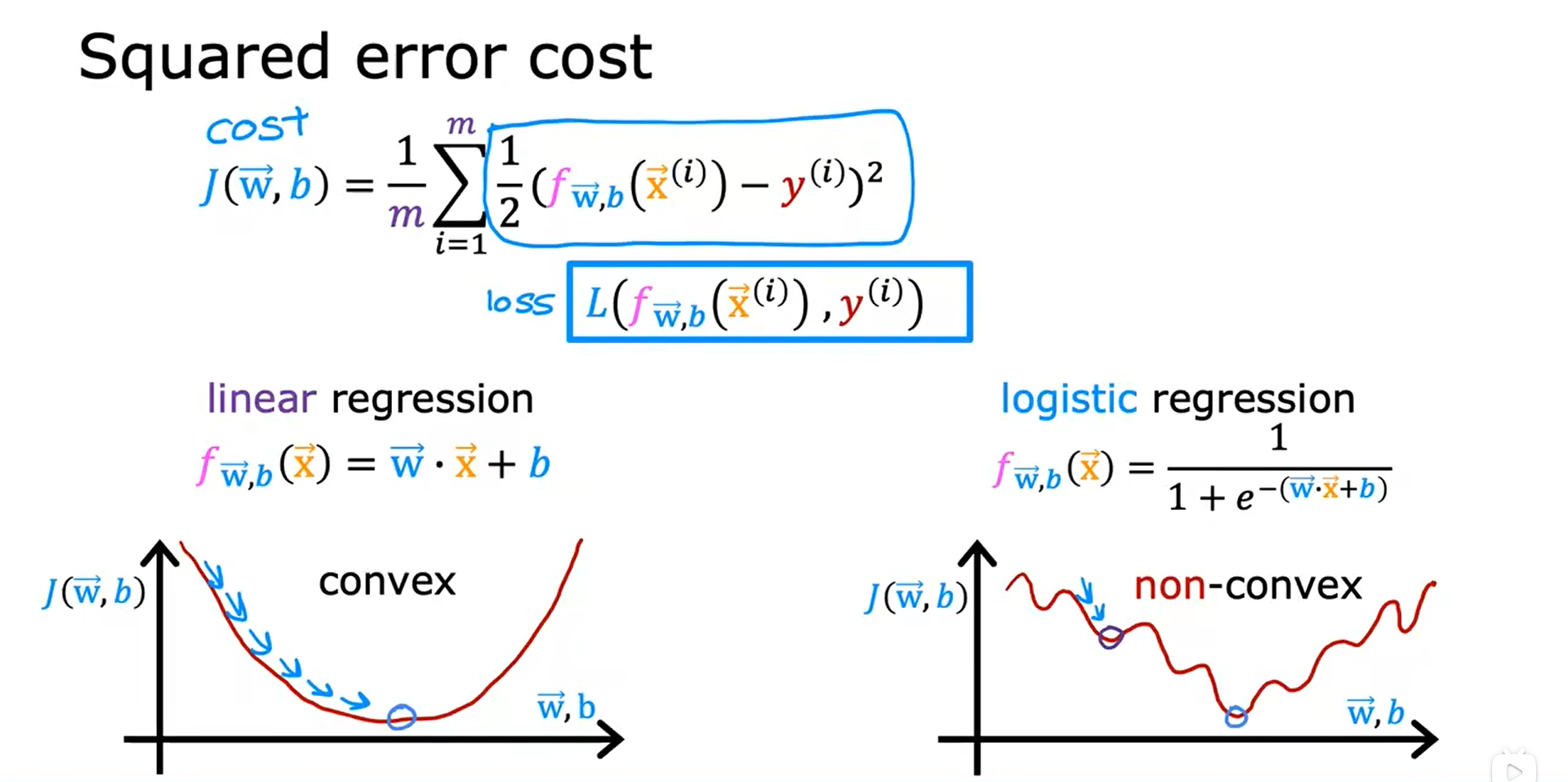## 逻辑回归的损失函数

      y

(

i

)

y^{(i)}

y(i) 等于1时，损失函数会帮助算法做出更准确的预测，因为当它预测的值接近1时，损失是最低的。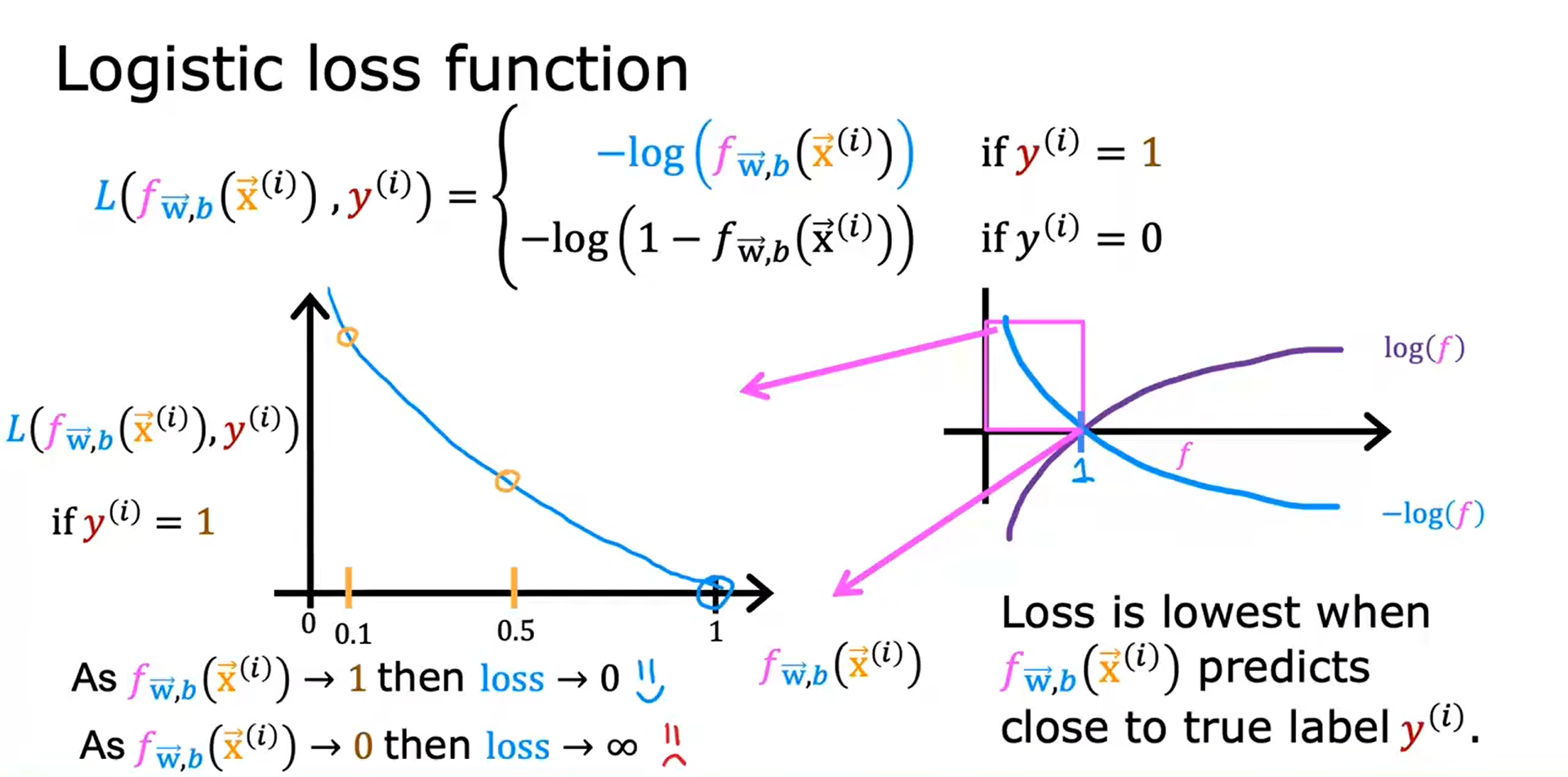y

(

i

)

y^{(i)}

y(i) 等于0时，

f

w

⃗

,

b

(

x

(

i

)

⃗

)

f_{\vec{w},b}(\vec{x^{(i)}})

fw,b​(x(i)) 越远离

y

(

i

)

y^{(i)}

y(i) ，损失越大。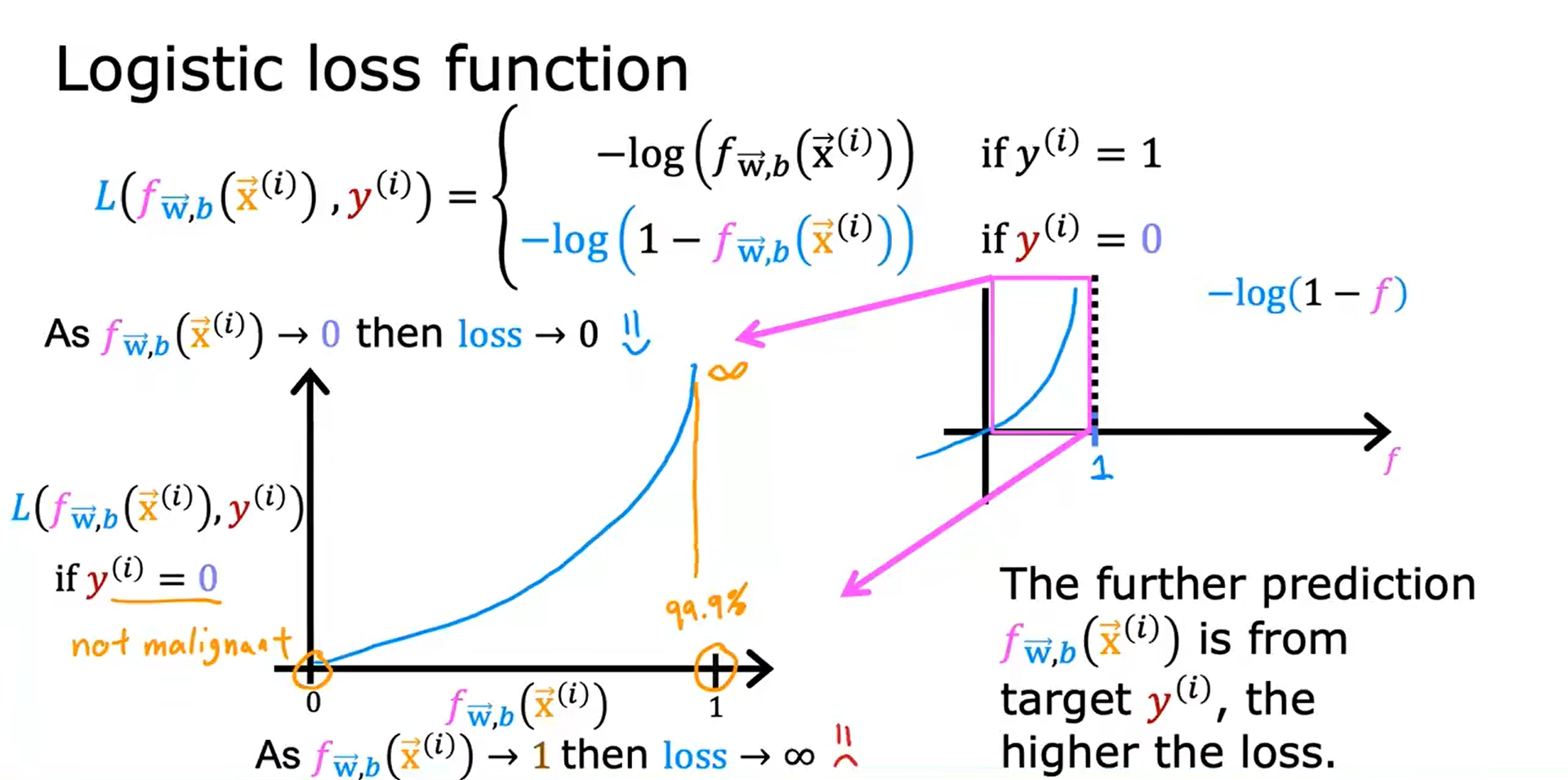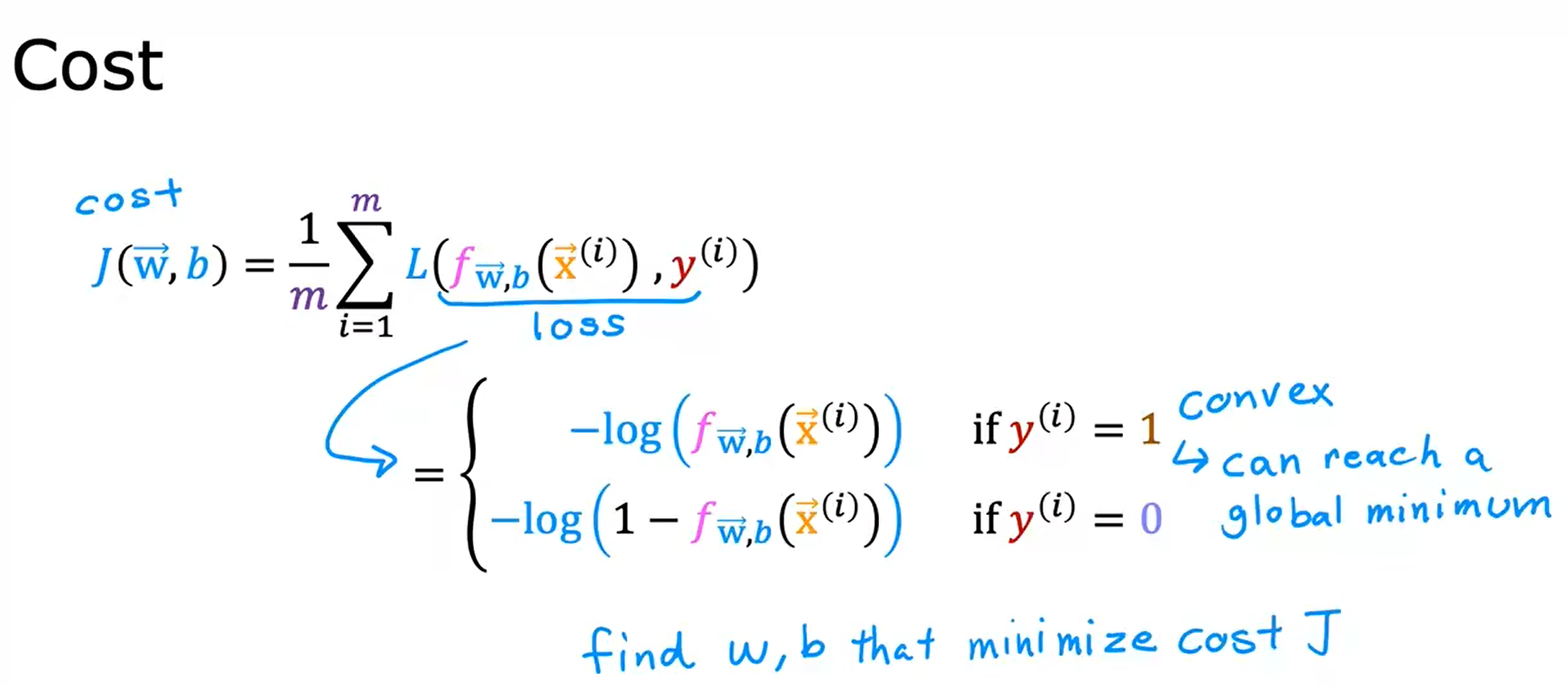## 逻辑回归的简化版成本函数

      y

(

i

)

y^{(i)}

y(i) 为1还是0，都会得到原来的表达式：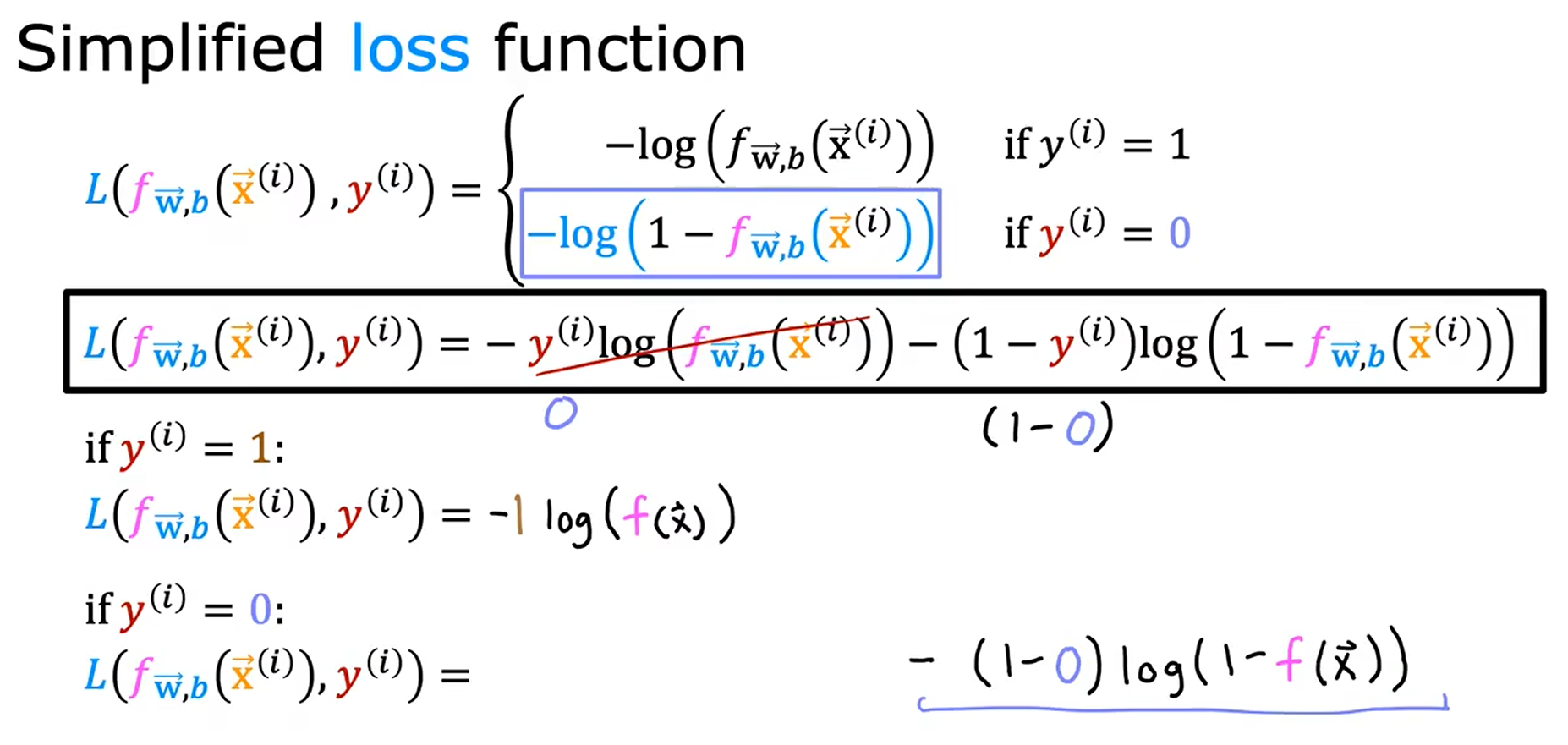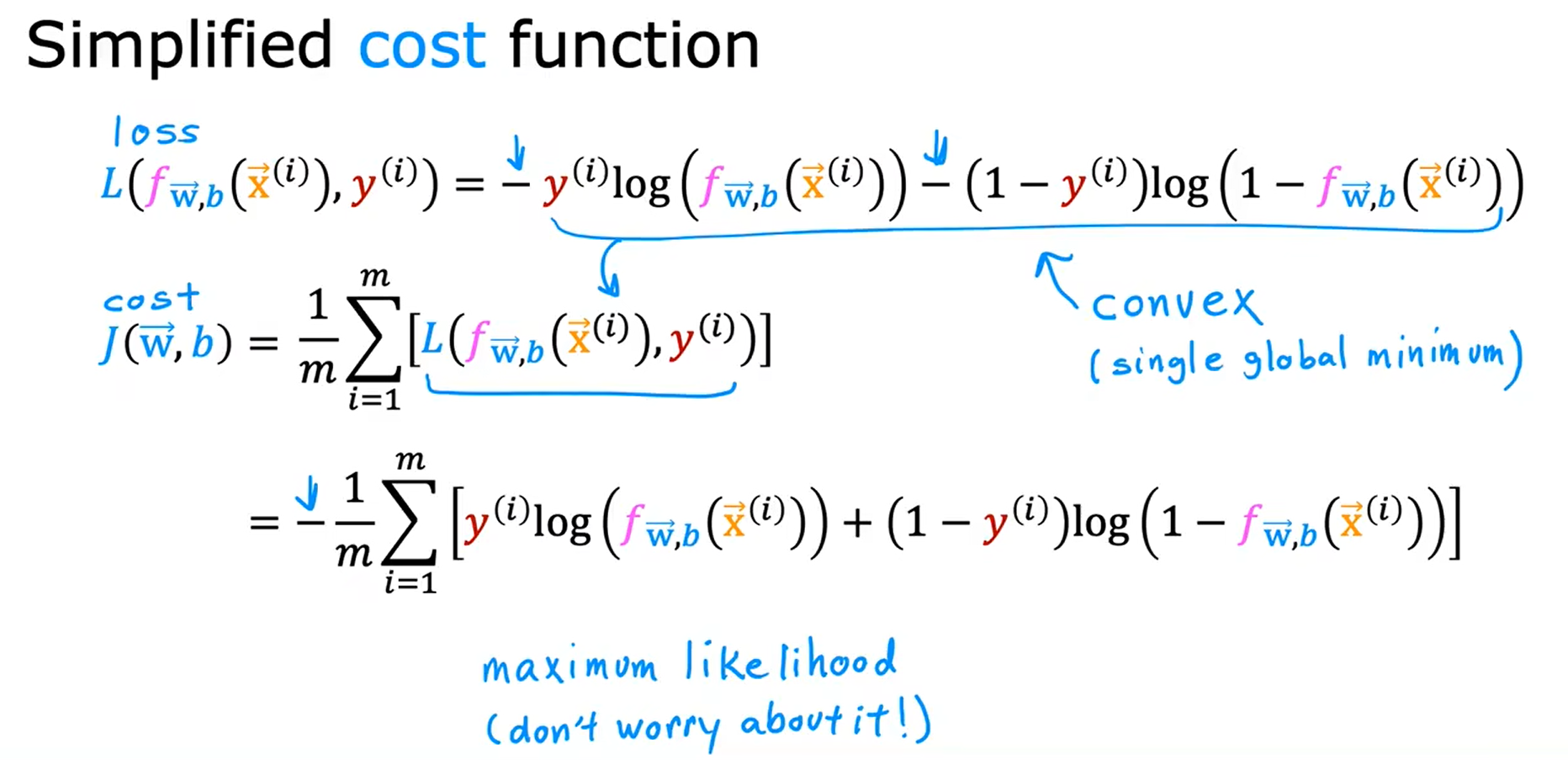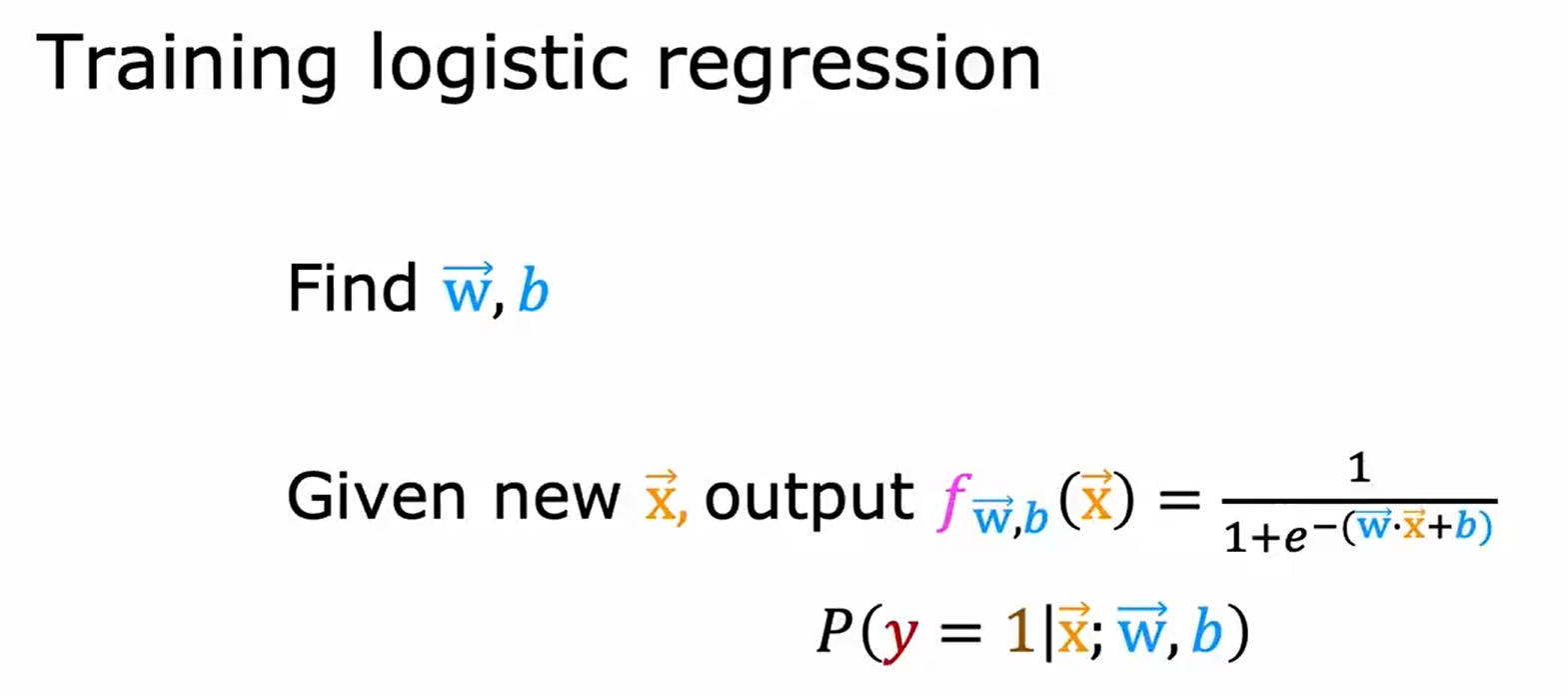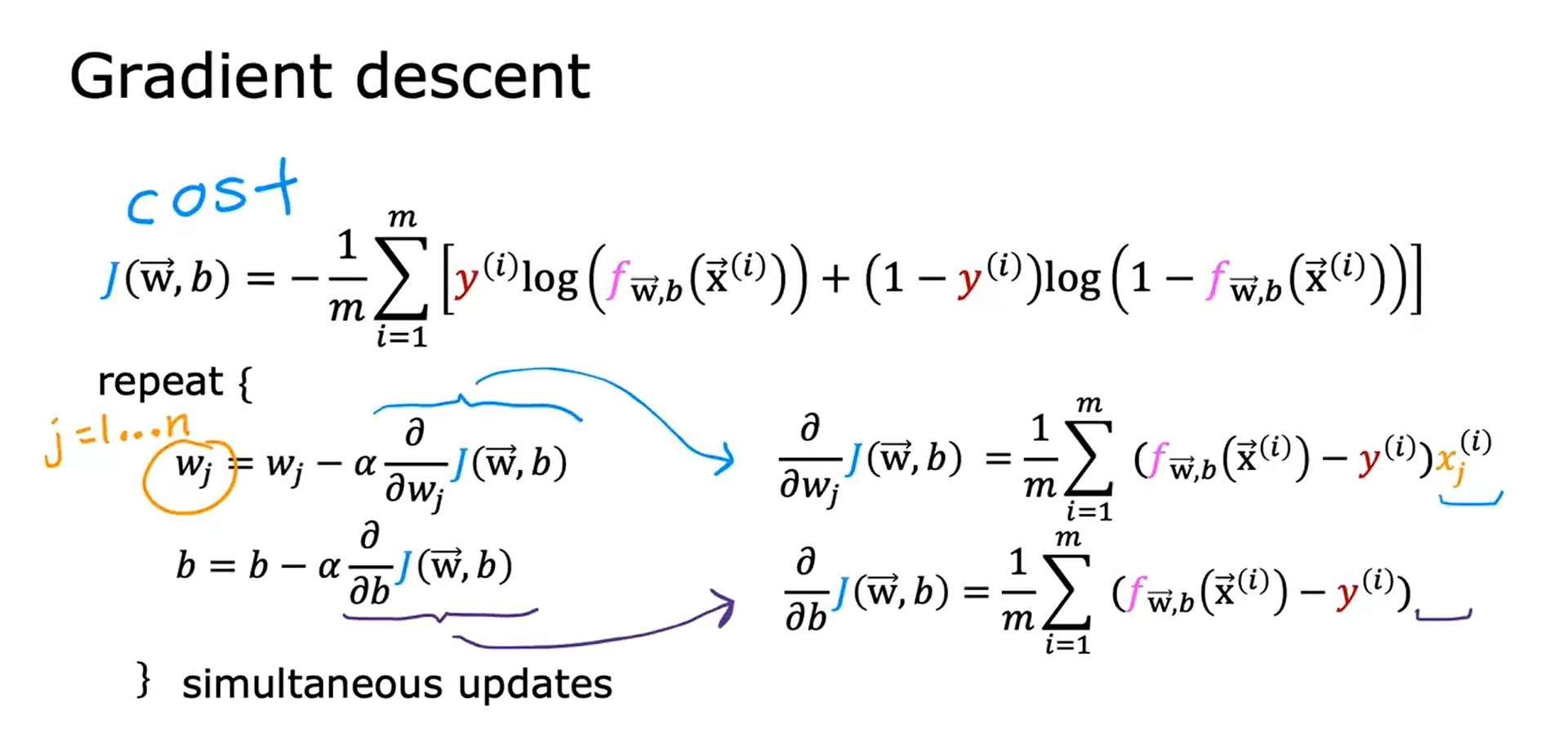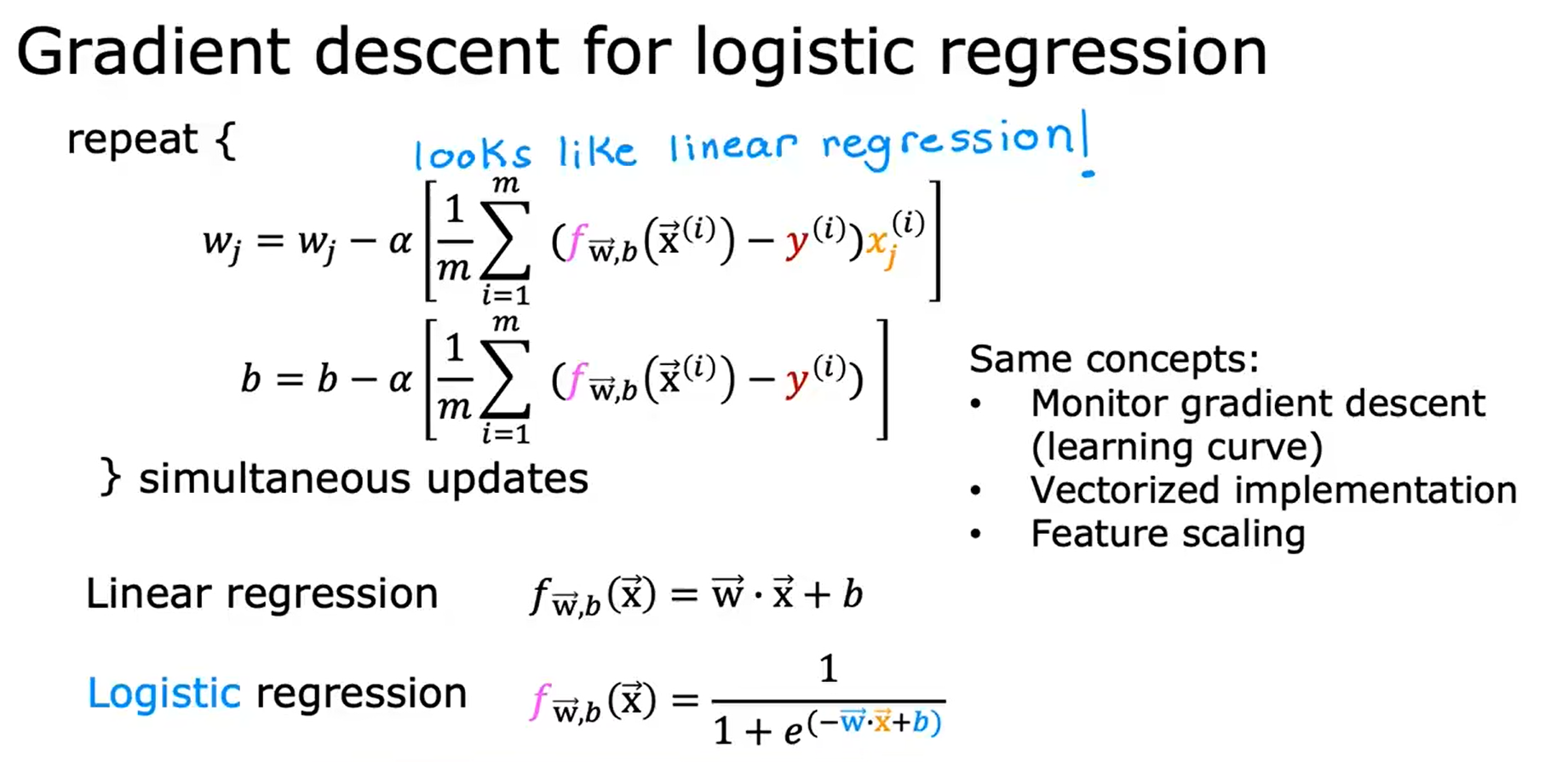## 过拟合的问题 The Proble Of Overfitting

Regression example：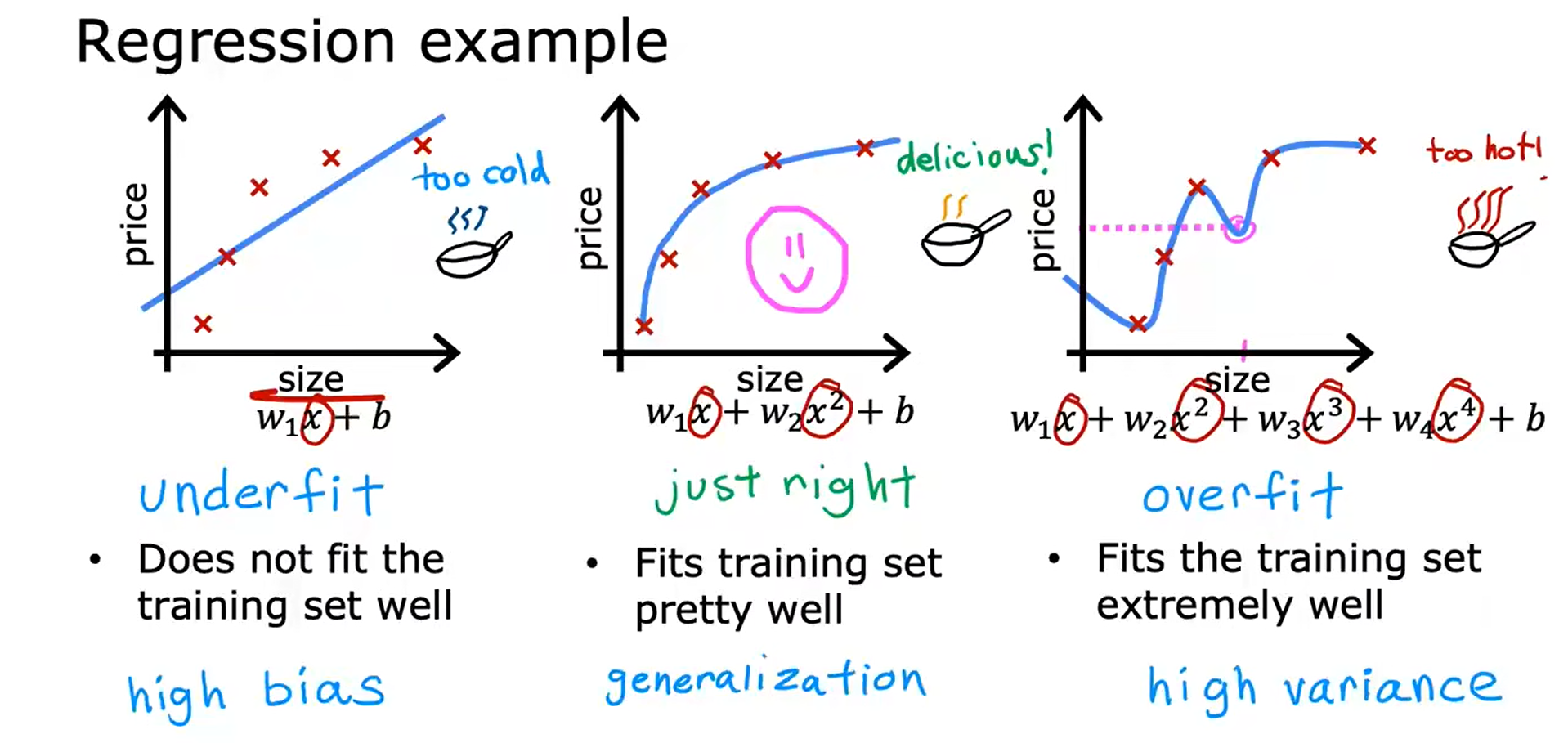Classification example：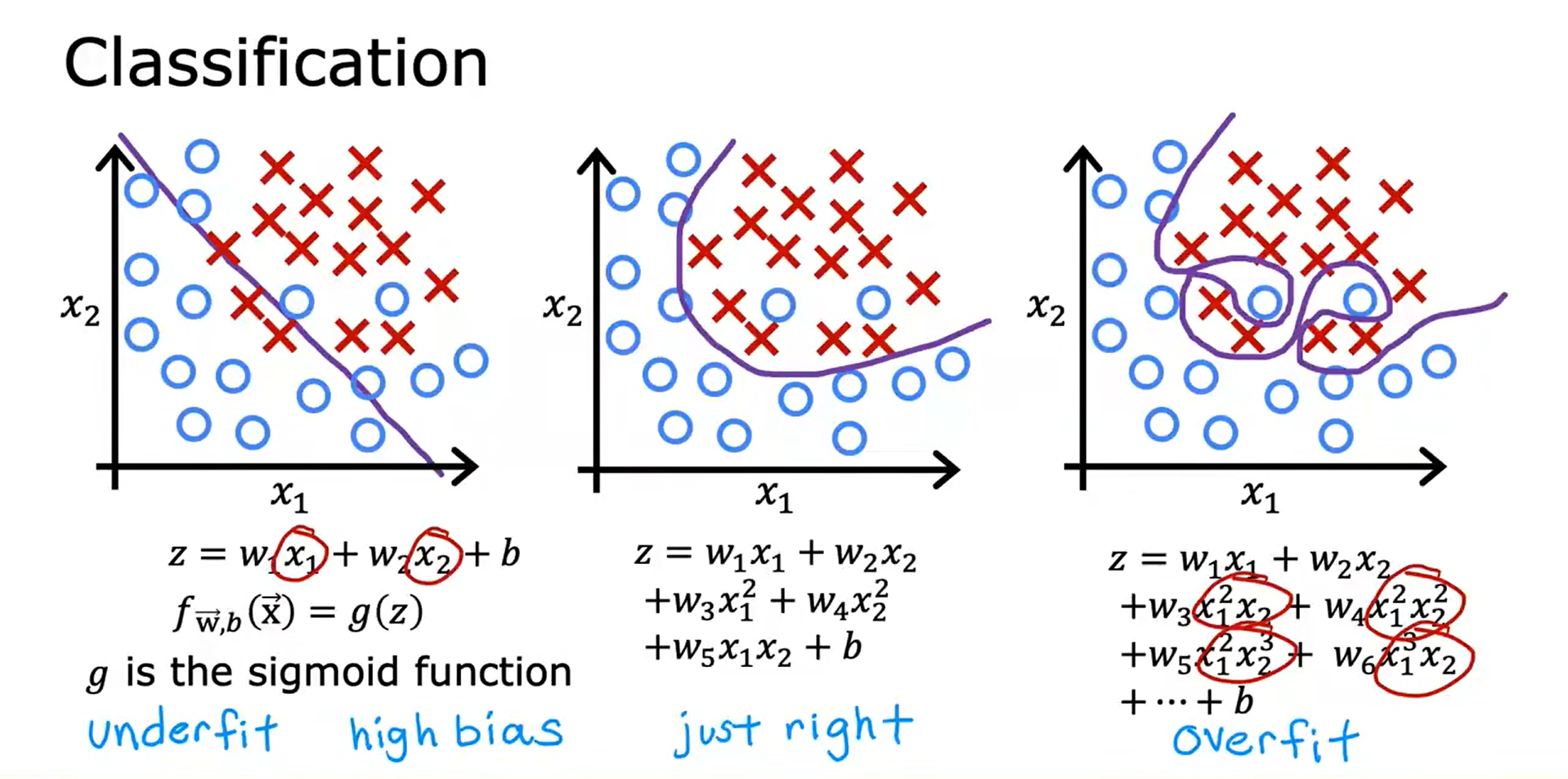1. 收集更多的训练数据（Collect more training examples, Collect more data）
2. 选择要包含/排除的特性（Select features to include/exclude, Select features）
3. 正规化（Regularization, Reduce size of parameters） - 减少参数的大小，正规化是一种更温和地减少一些特征影响的方法。- 正规化鼓励学习算法缩小参数的值，而不一定要求参数被设置为零。- 正规化所做的是保持所有的特征，但只是防止某个特征产生过大的影响。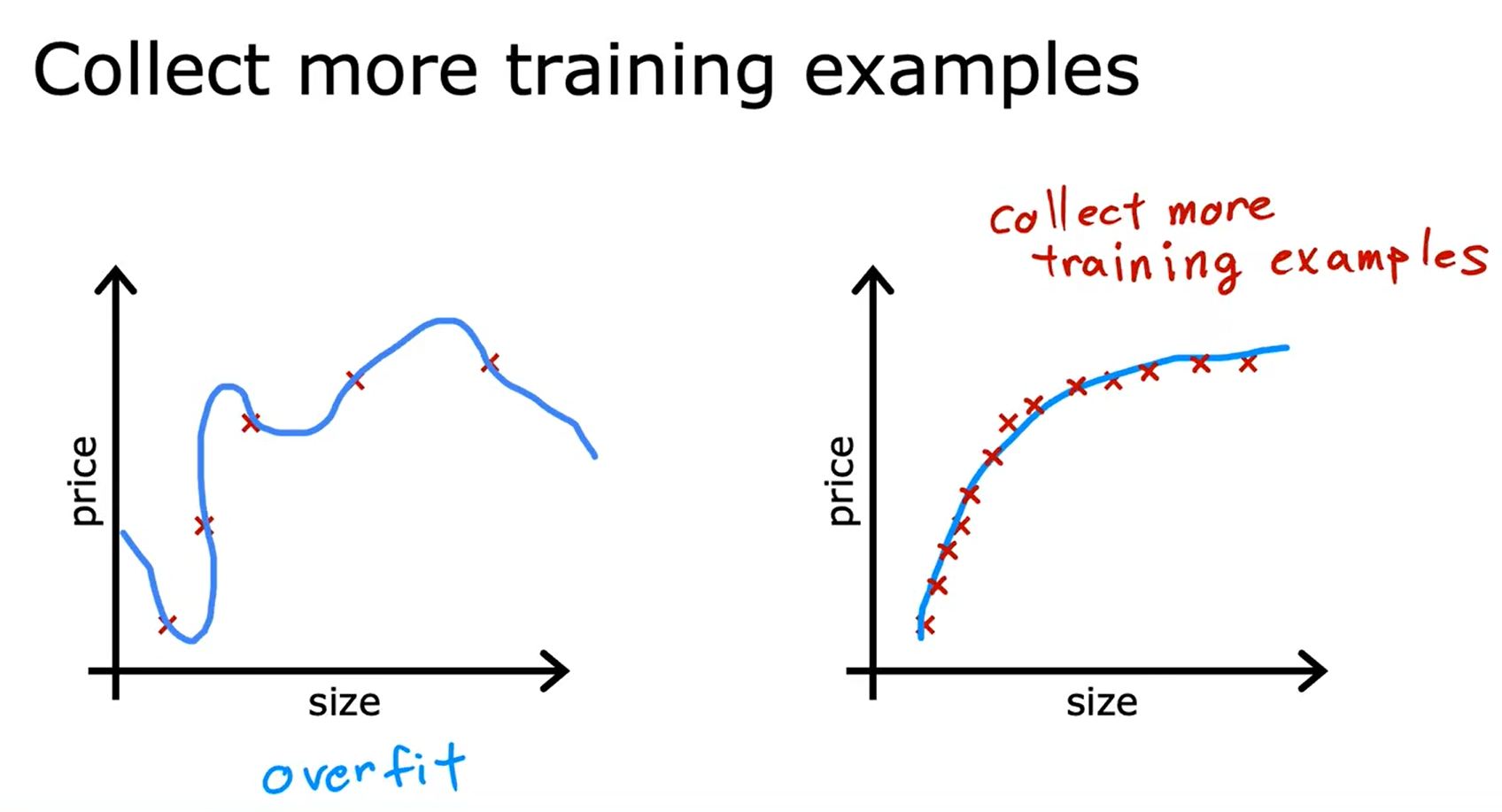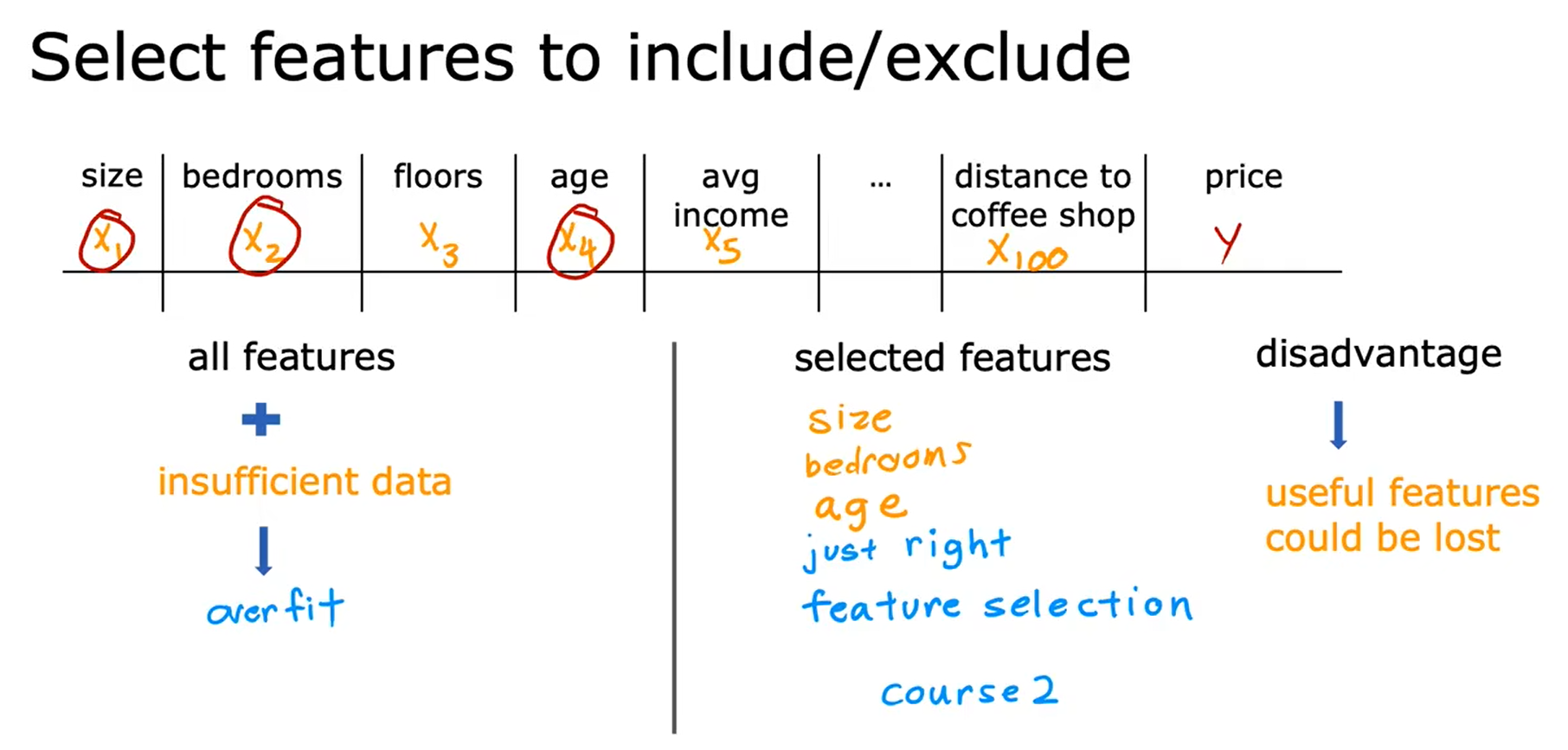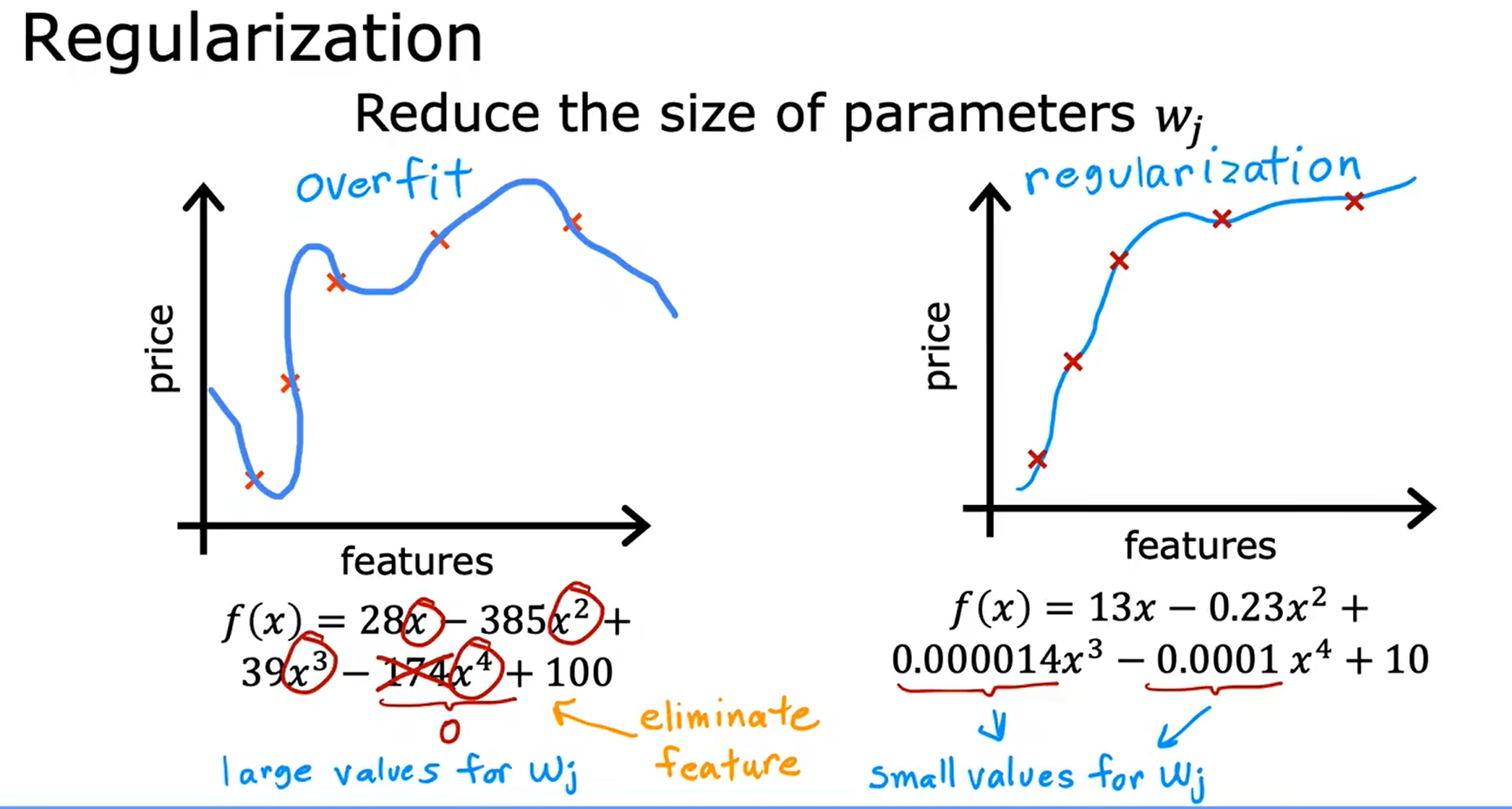## 正则化代价函数 Cost Function With Regularization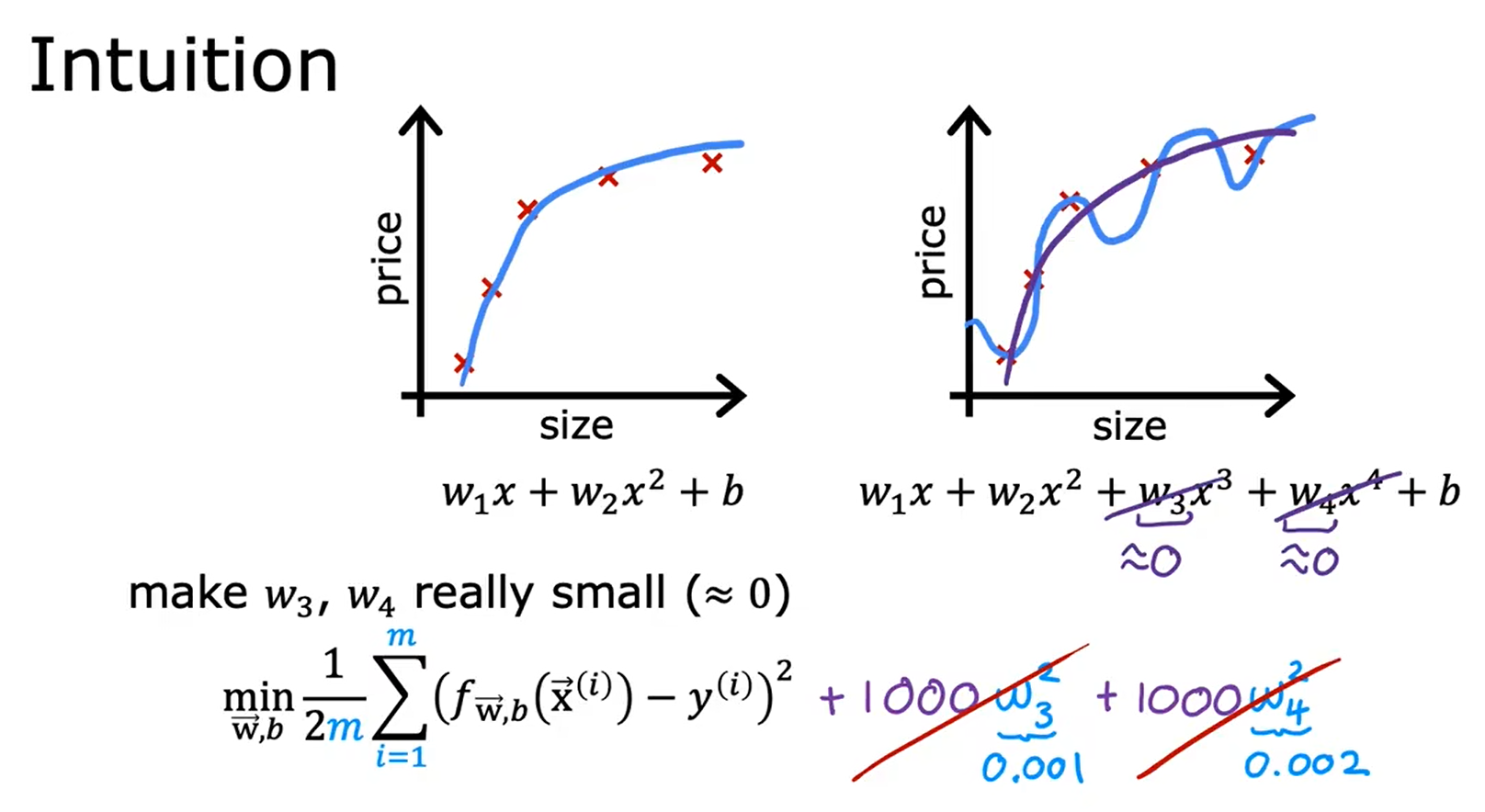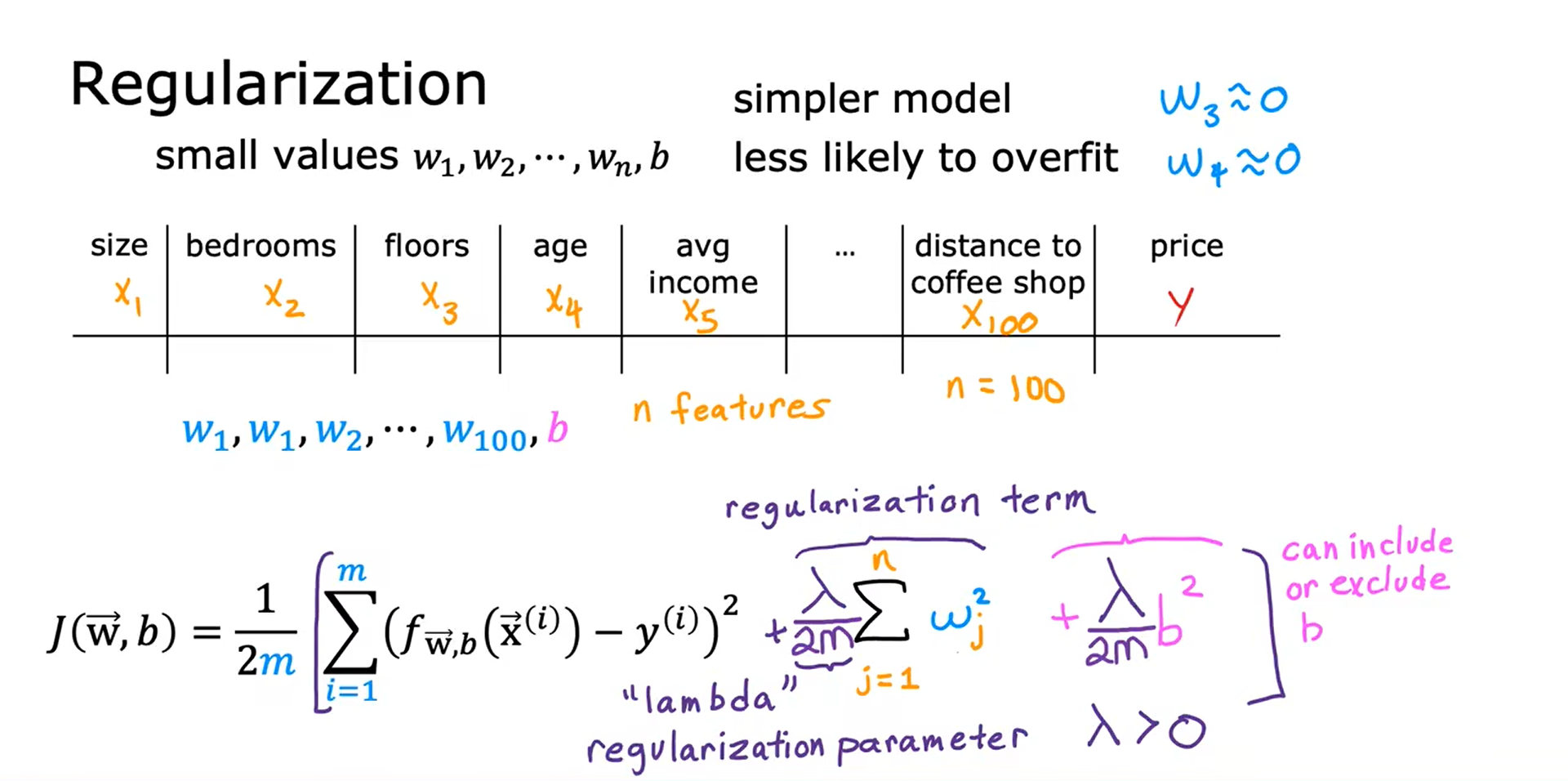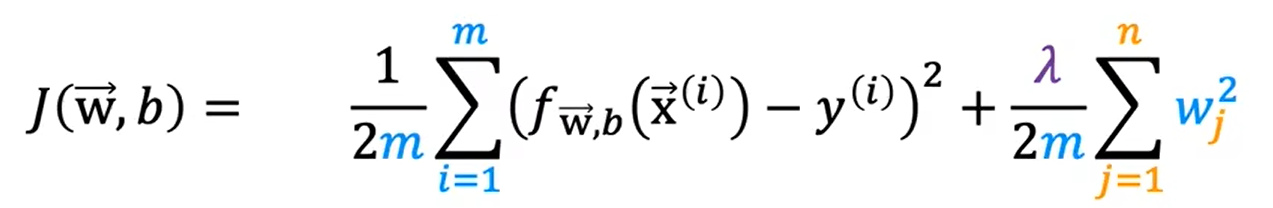1. 若 λ = 0，即没有使用正则化项，则最终会过拟合。
2. 若 λ = 1 0 10 10^{10} 1010，则使其最小化的唯一方法是让w的值非常接近于0，则 f(x) = b 会成立，会导致欠拟合。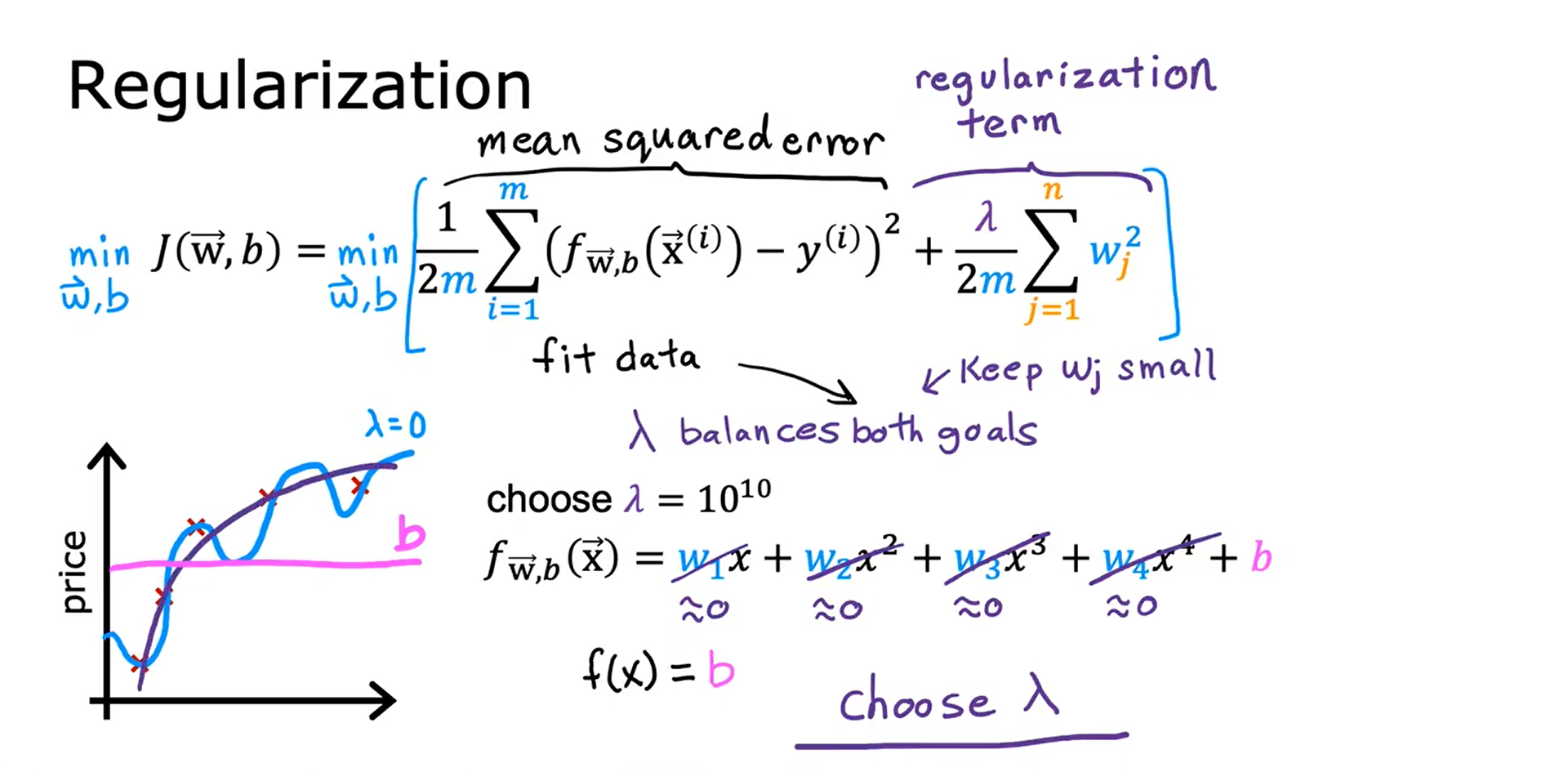## 正则化线性回归 Regularized Linear Regression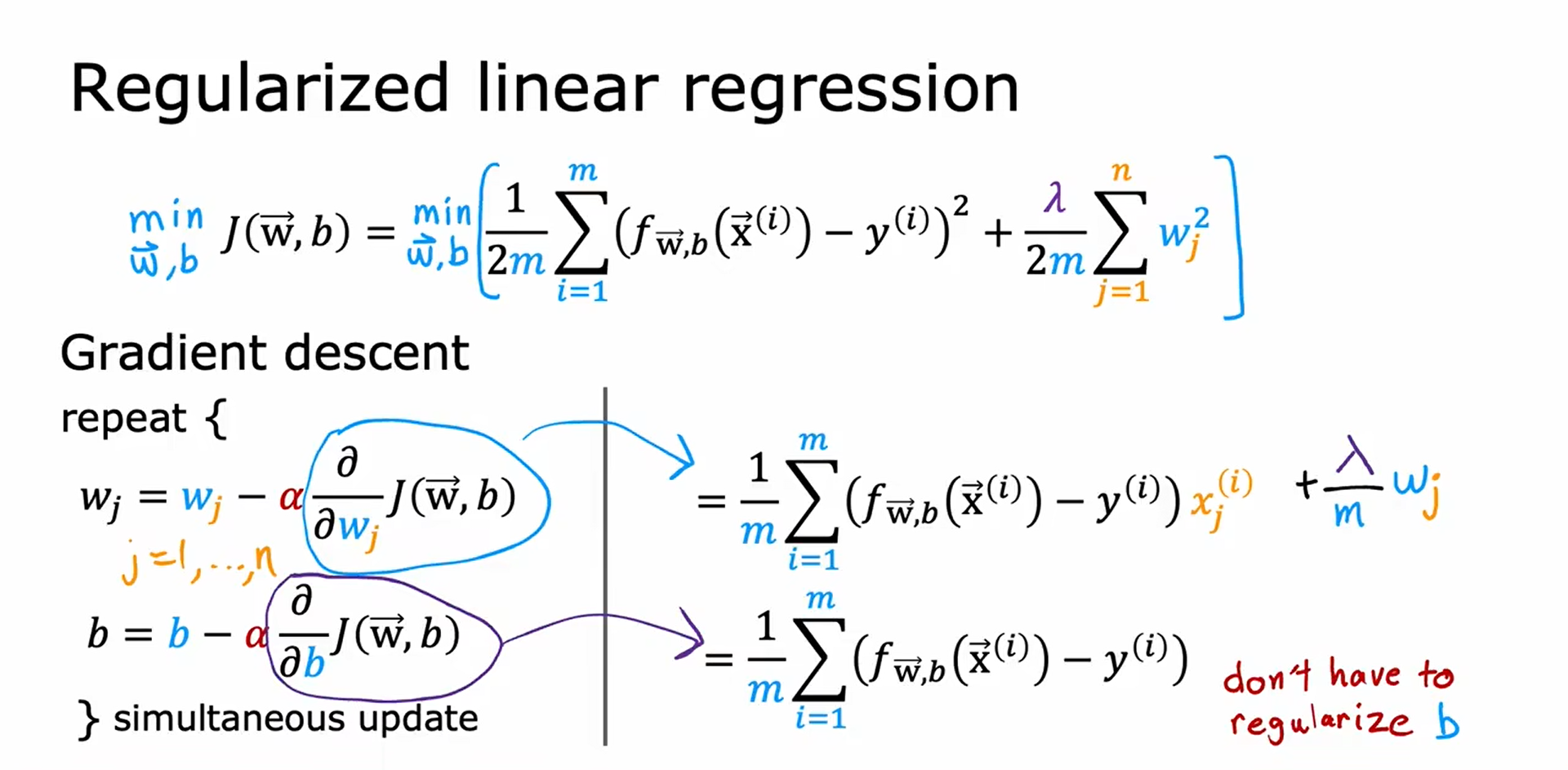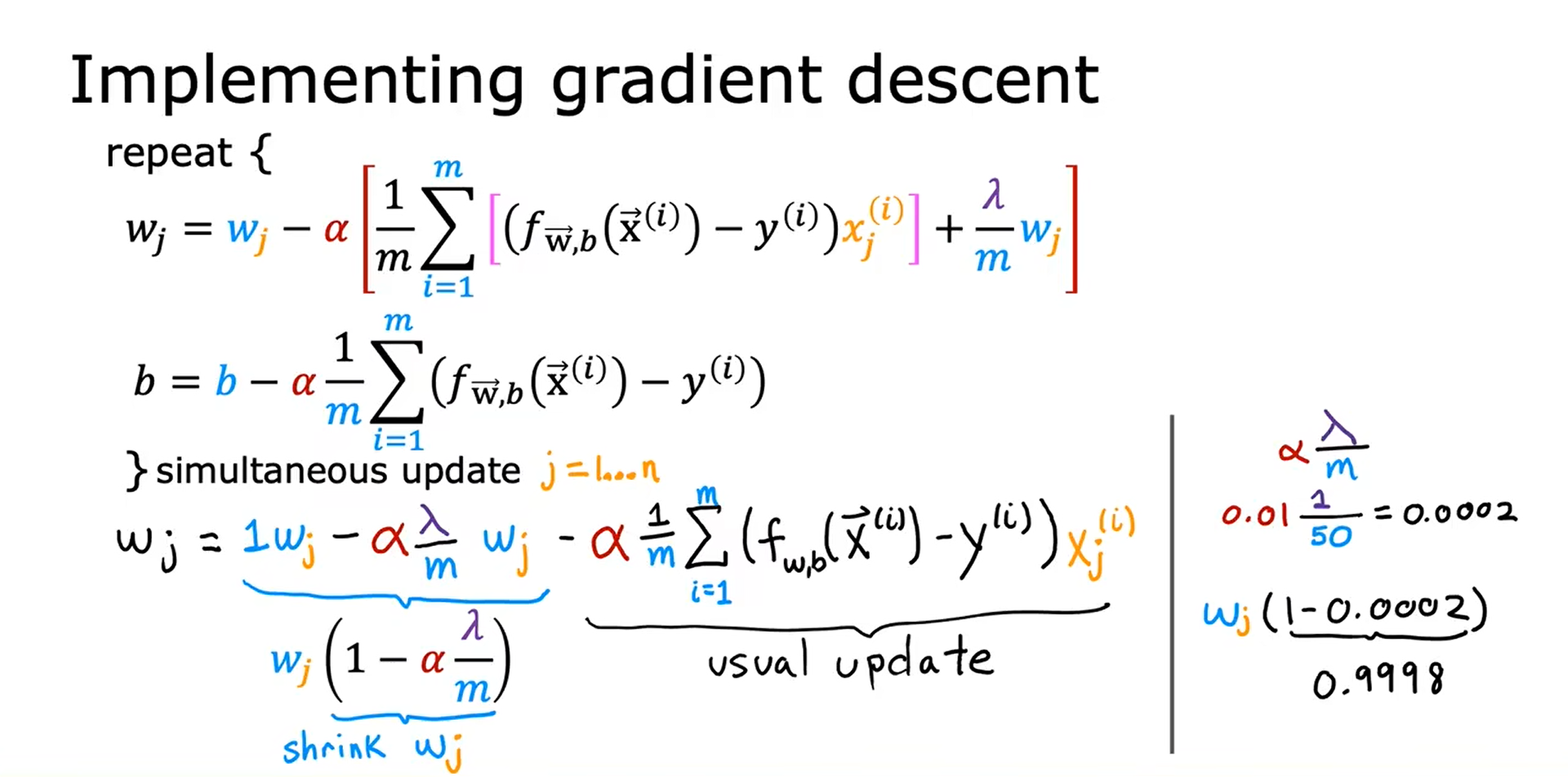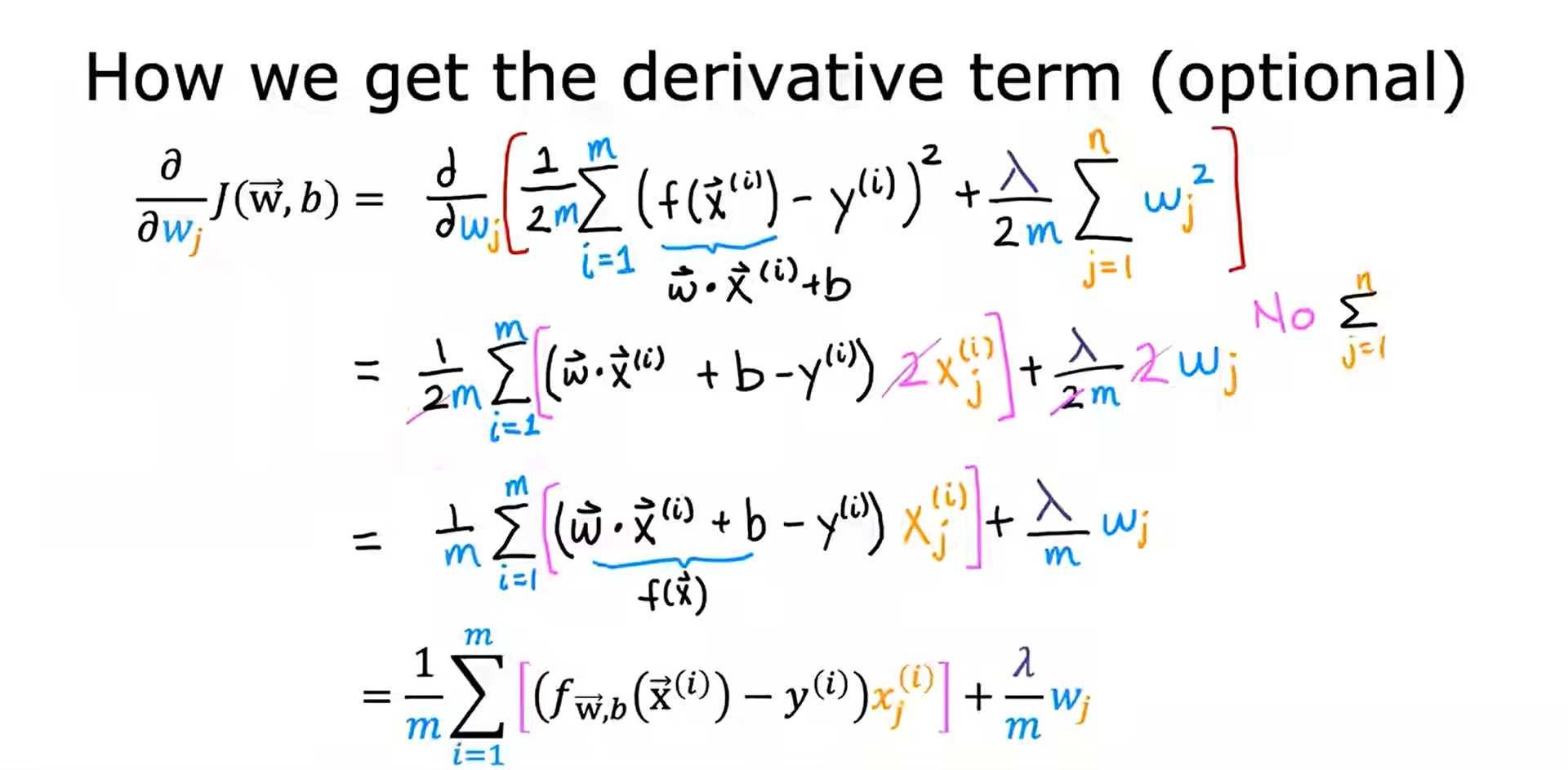## 正则化逻辑回归 Regularized Logistic Regression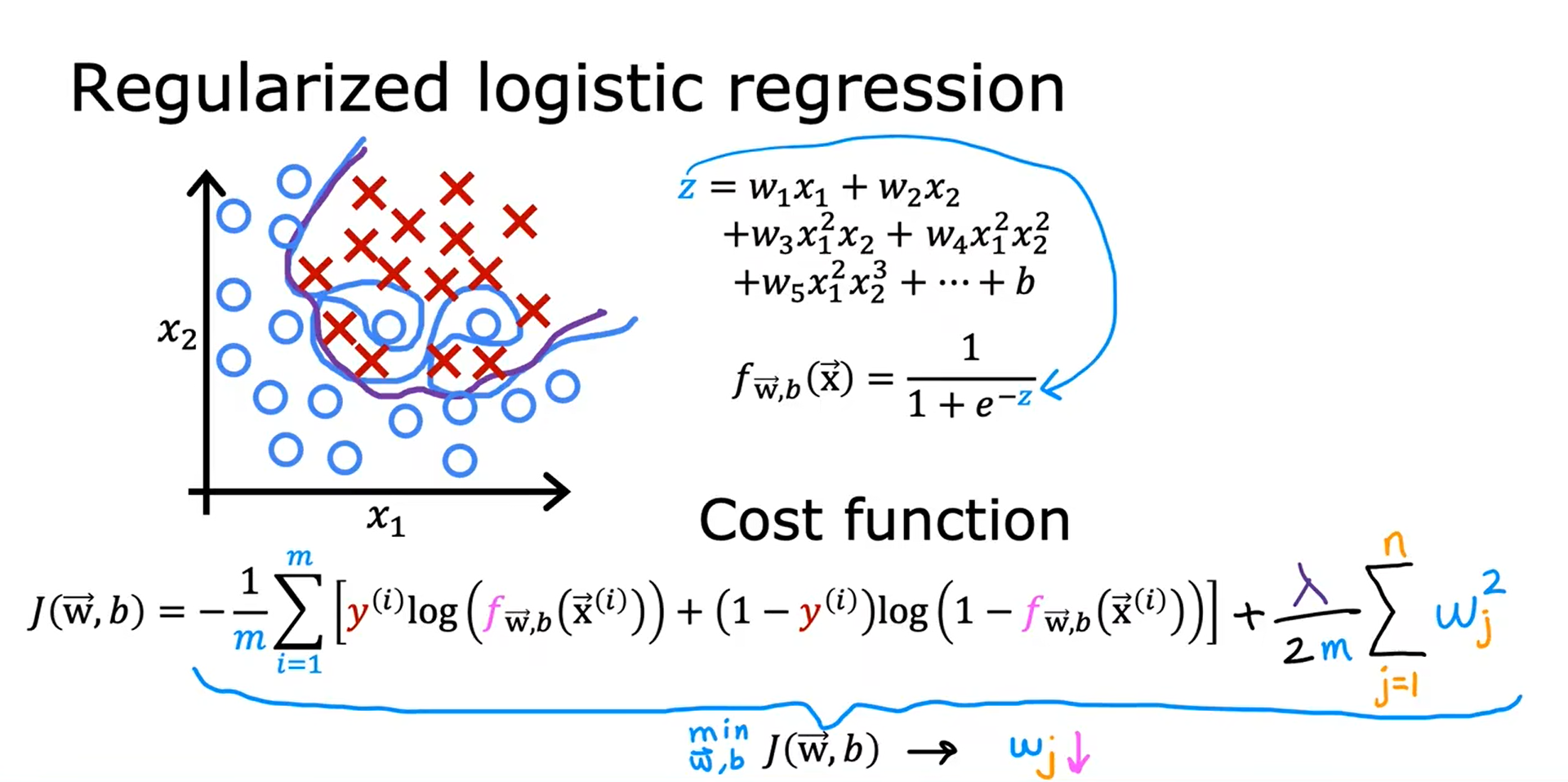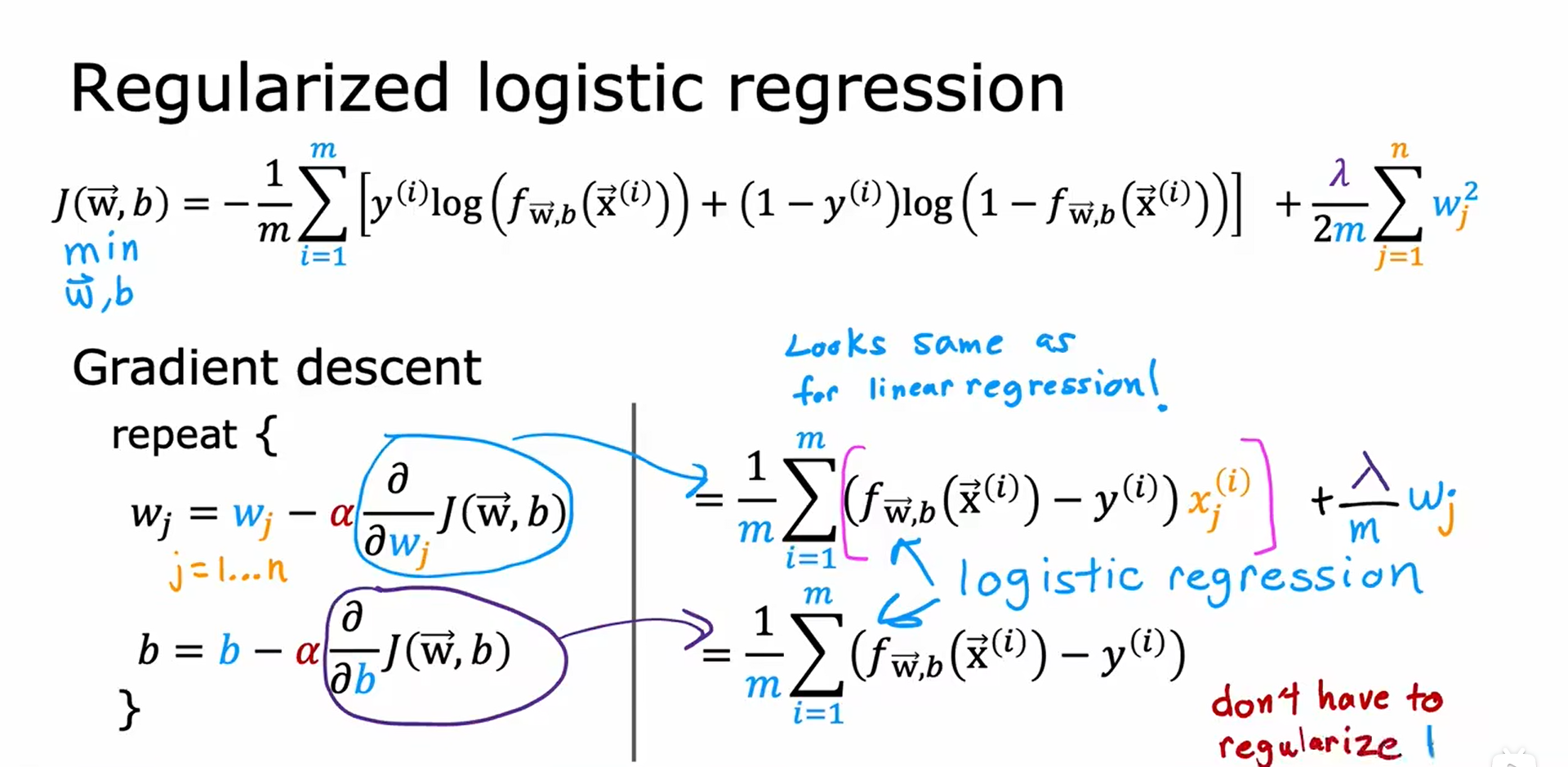### “机器学习（Machine Learning）”的评论:

##### 关于作者##### overfit同步小助手